### Still have math questions?

Q: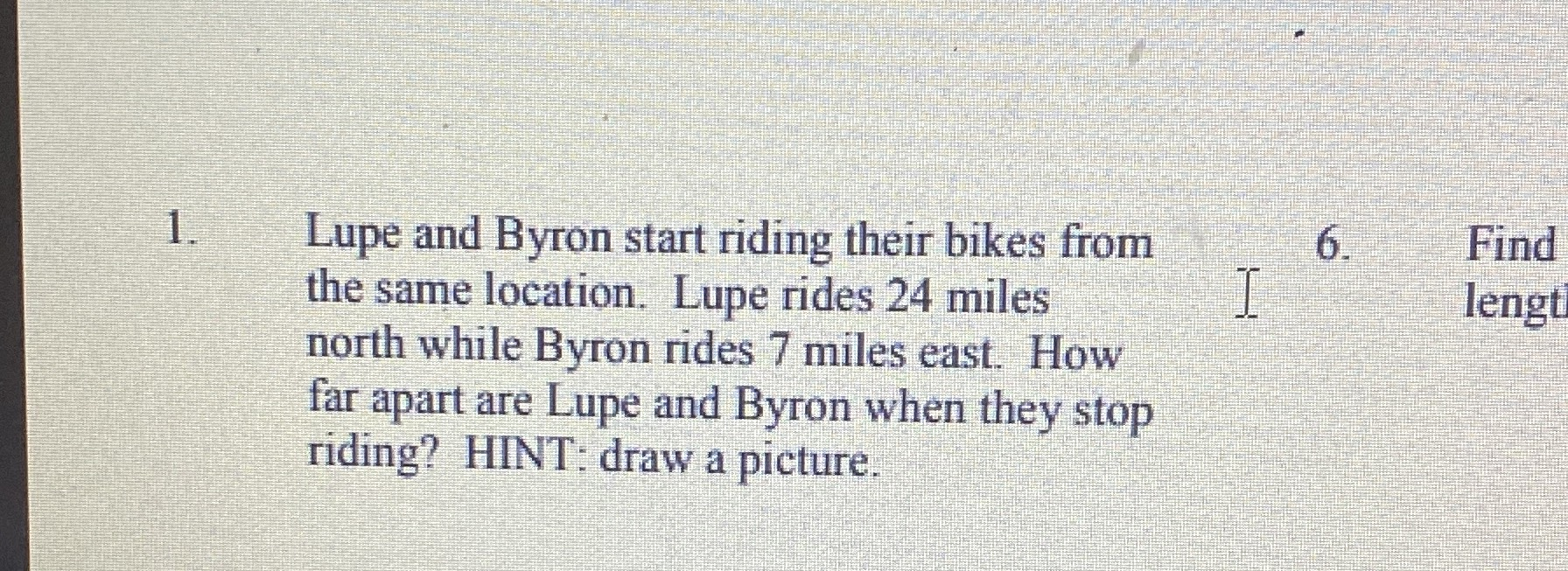Lupe and Byron start riding their bikes from the same location. Lupe rides $$24$$ miles north while Byron rides $$7$$ miles east. How far apart are Lupe and Byron when they stop riding? HINT: draw a picture.

Q: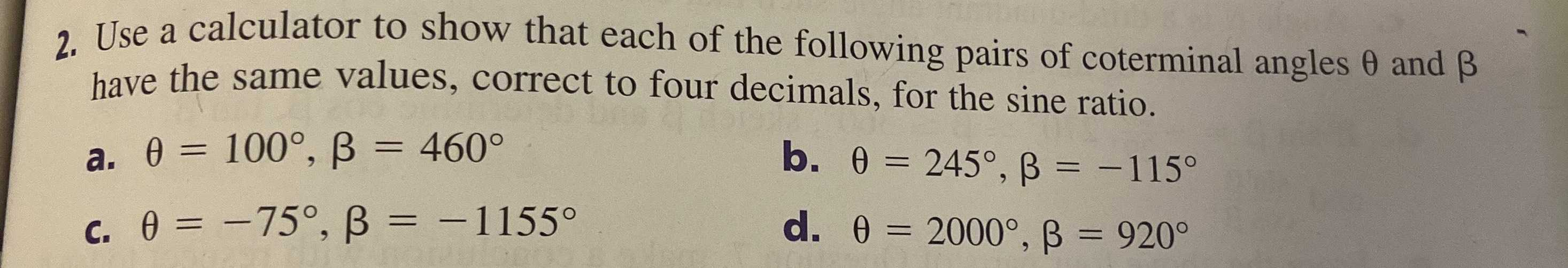Use a calculator to show that each of the following pairs of coterminal angles $$\theta$$ and $$\beta$$ have the same values, correct to four decimals, for the sine ratio.

a. $$\theta = 100 ^ { \circ } , \beta = 460 ^ { \circ }$$ b. $$\theta = 245 ^ { \circ } , \beta = - 115 ^ { \circ }$$

c. $$\theta = - 75 ^ { \circ } , \beta = - 1155 ^ { \circ }$$ d. $$\theta = 2000 ^ { \circ } , \beta = 920 ^ { \circ }$$

Q:A zip line is to be built between two towers $$A$$ and $$B$$ across a wetland area. To approximate the distance of the zip line, a surveyor marks a third point $$C$$ , a distance of $$225 ft$$ from one end of the zip line and perpendicular to the zip line. The measure of $$\angle A C B$$ is $$76.5 ^ { \circ }$$ . How long is the zip line? Round to the nearest foot.

A) $$53 ft$$

B) $$937 ft$$

C) $$219 ft$$

D) $$54 ft$$

Q: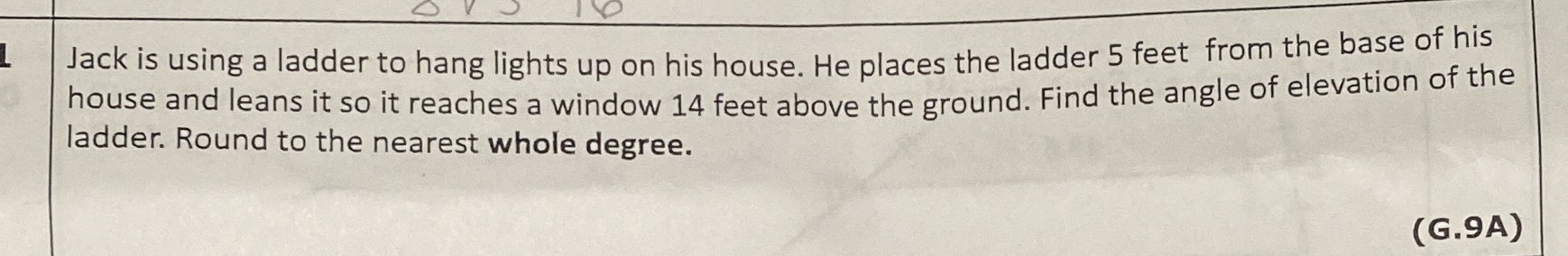Jack is using a ladder to hang lights up on his house. He places the ladder $$5$$ feet from the base of his house and leans it so it reaches a window $$14$$ feet above the ground. Find the angle of elevation of the ladder. Round to the nearest whole degree.

Q: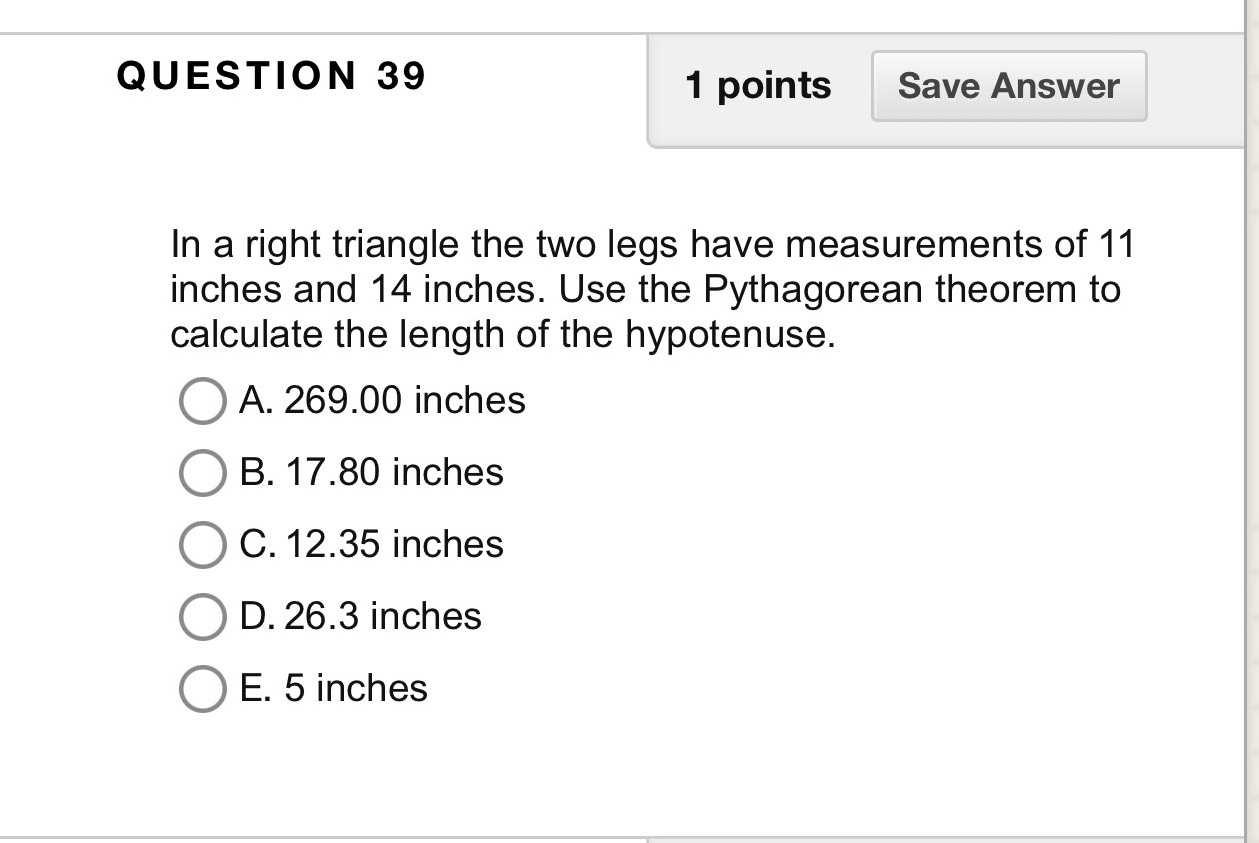In a right triangle, the two legs have measurements of $$11$$ inches and $$14$$ inches. Use the Pythagorean theorem to calculate the length of the hypotenuse.

A. $$269.00$$ inches

B. $$17.80$$ inches

C. $$12.35$$ inches

D. $$26.3$$ inches

E. $$5$$ inches

Q: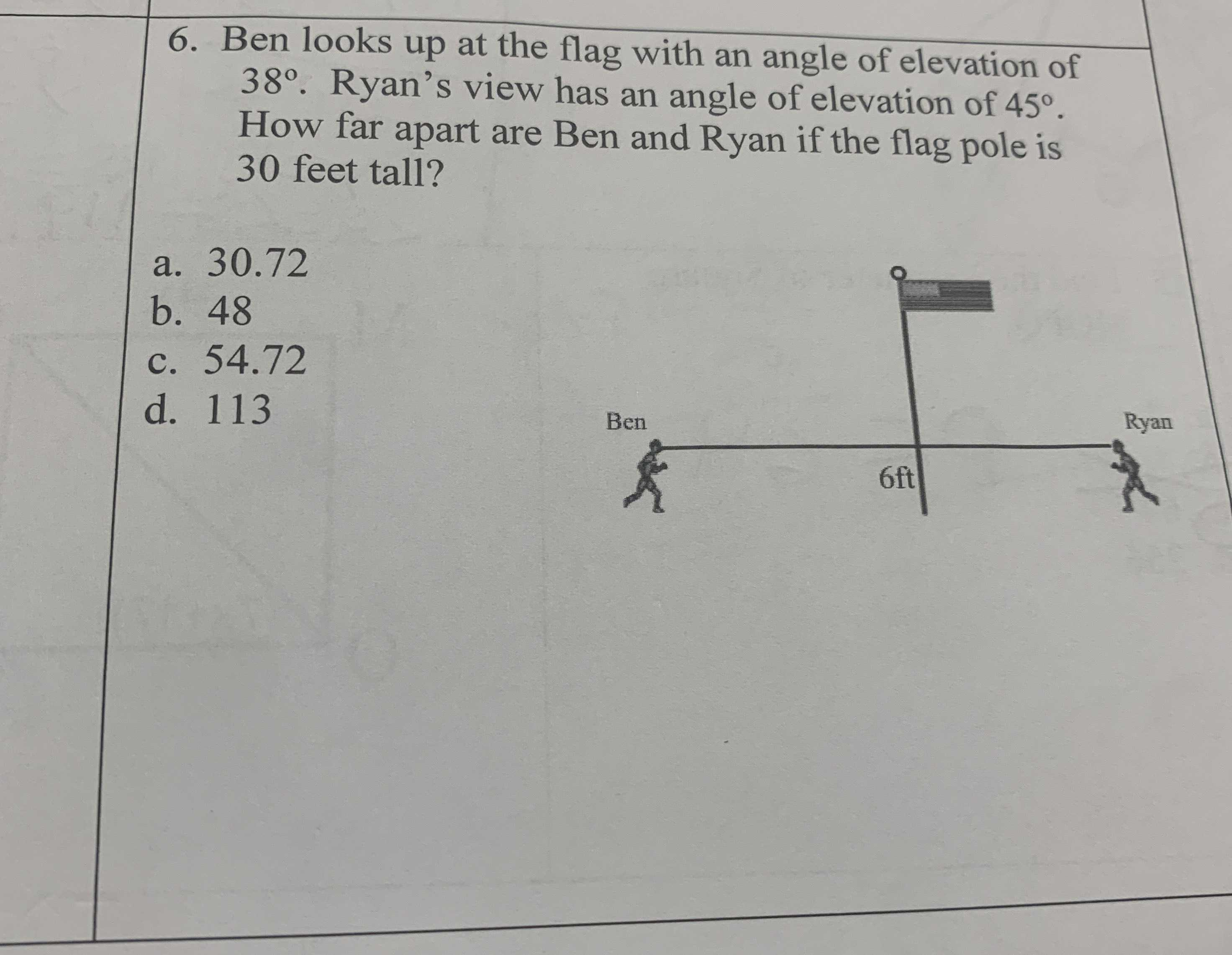Ben looks up at the flag with an angle of elevation of $$38 ^ { \circ }$$ . Ryan's view has an angle of elevation of $$45 ^ { \circ }$$ . How far apart are Ben and Ryan if the flag pole is $$30$$ feet tall?

a. $$30.72$$

b. $$48$$

c. $$54.72$$

d. $$113$$

Q: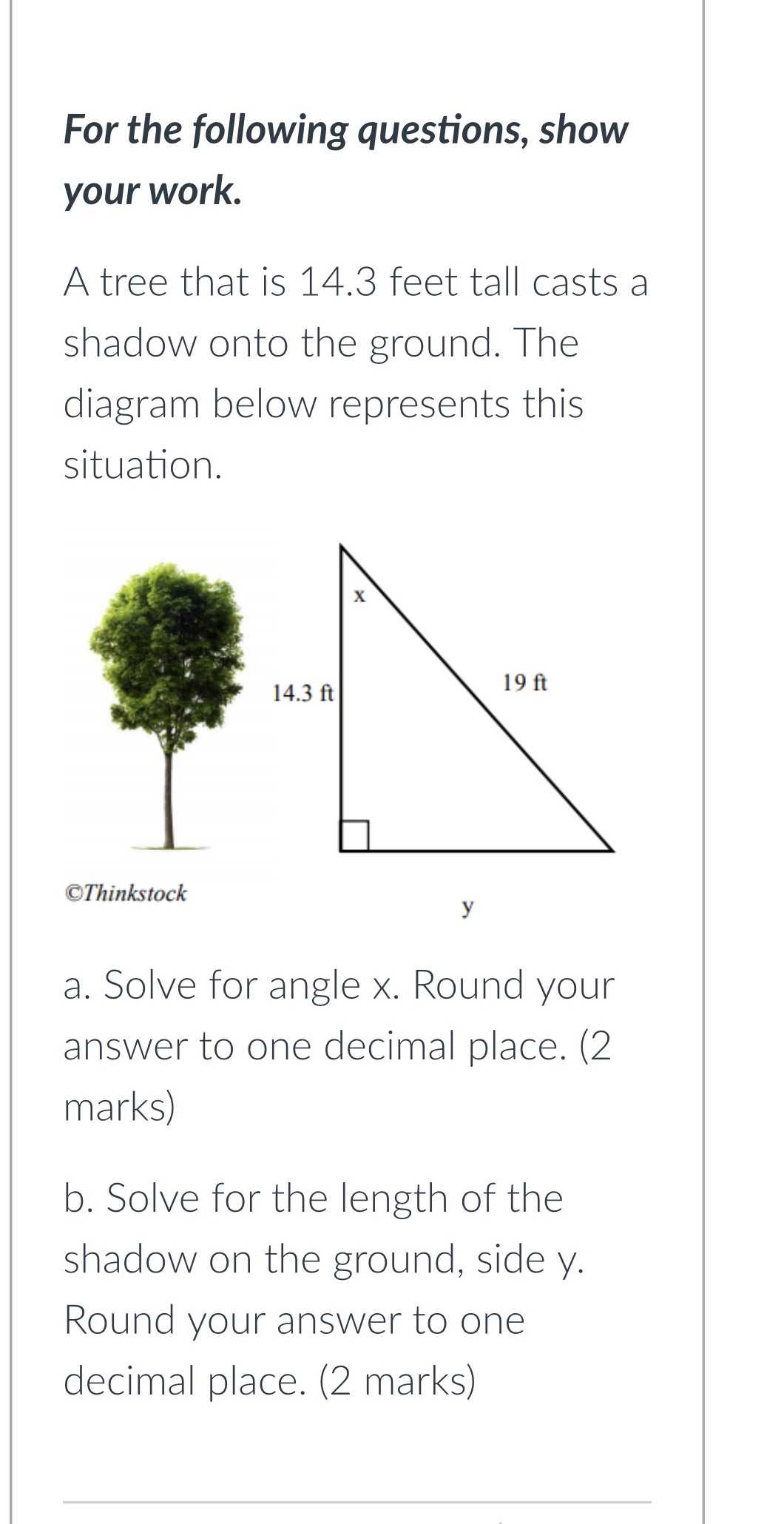A tree that is $$14.3$$ feet tall casts a shadow onto the ground. The diagram below represents this situation.

a. Solve for angle $$x$$ . Round your answer to one decimal place.

b. Solve for the length of the shadow on the ground, side $$y$$ . Round your answer to one decimal place.

Q: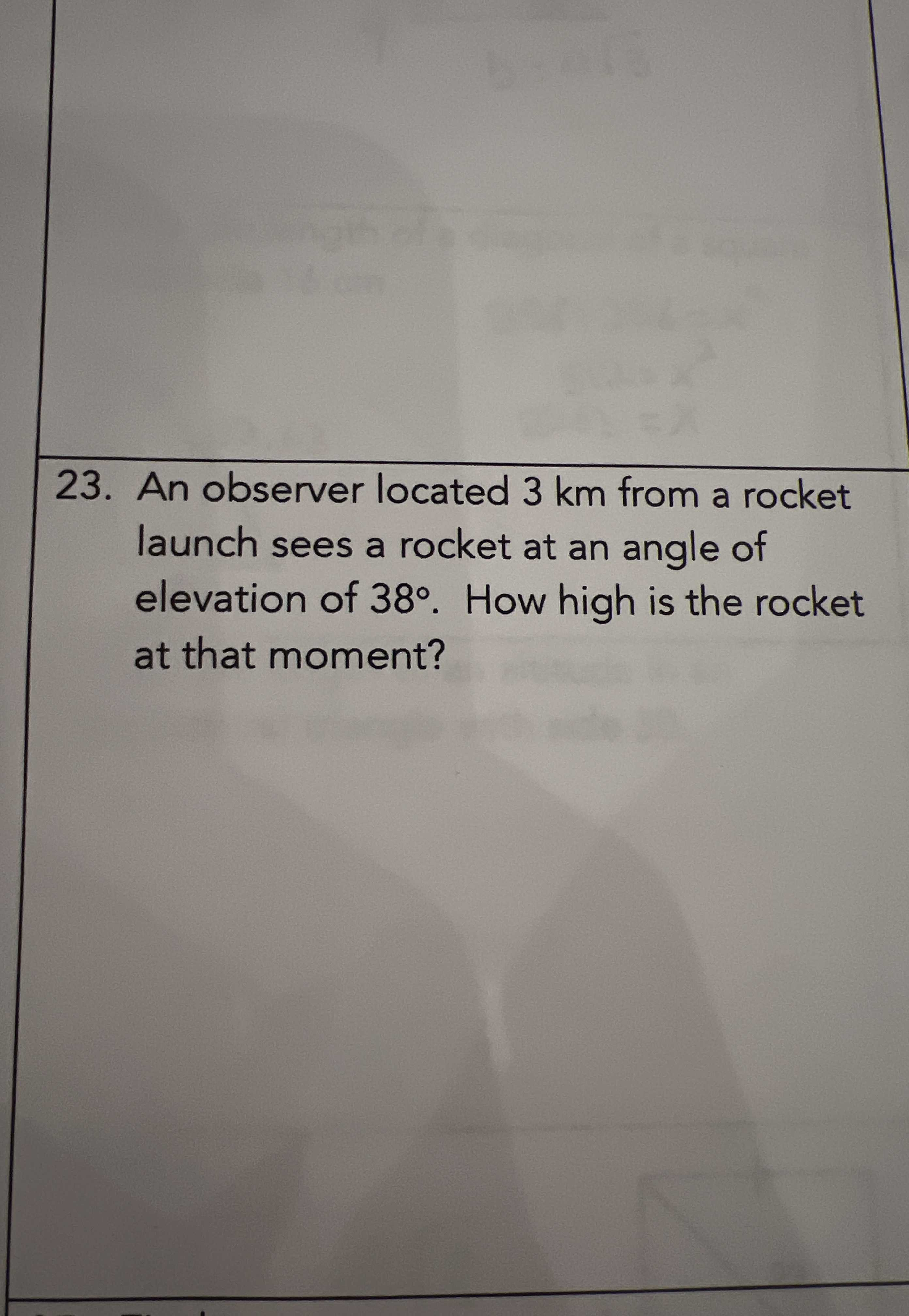An observer located $$3 km$$ from a rocket launch sees a rocket at an angle of elevation of $$38 ^ { \circ }$$ . How high is the rocket at that moment?

Q: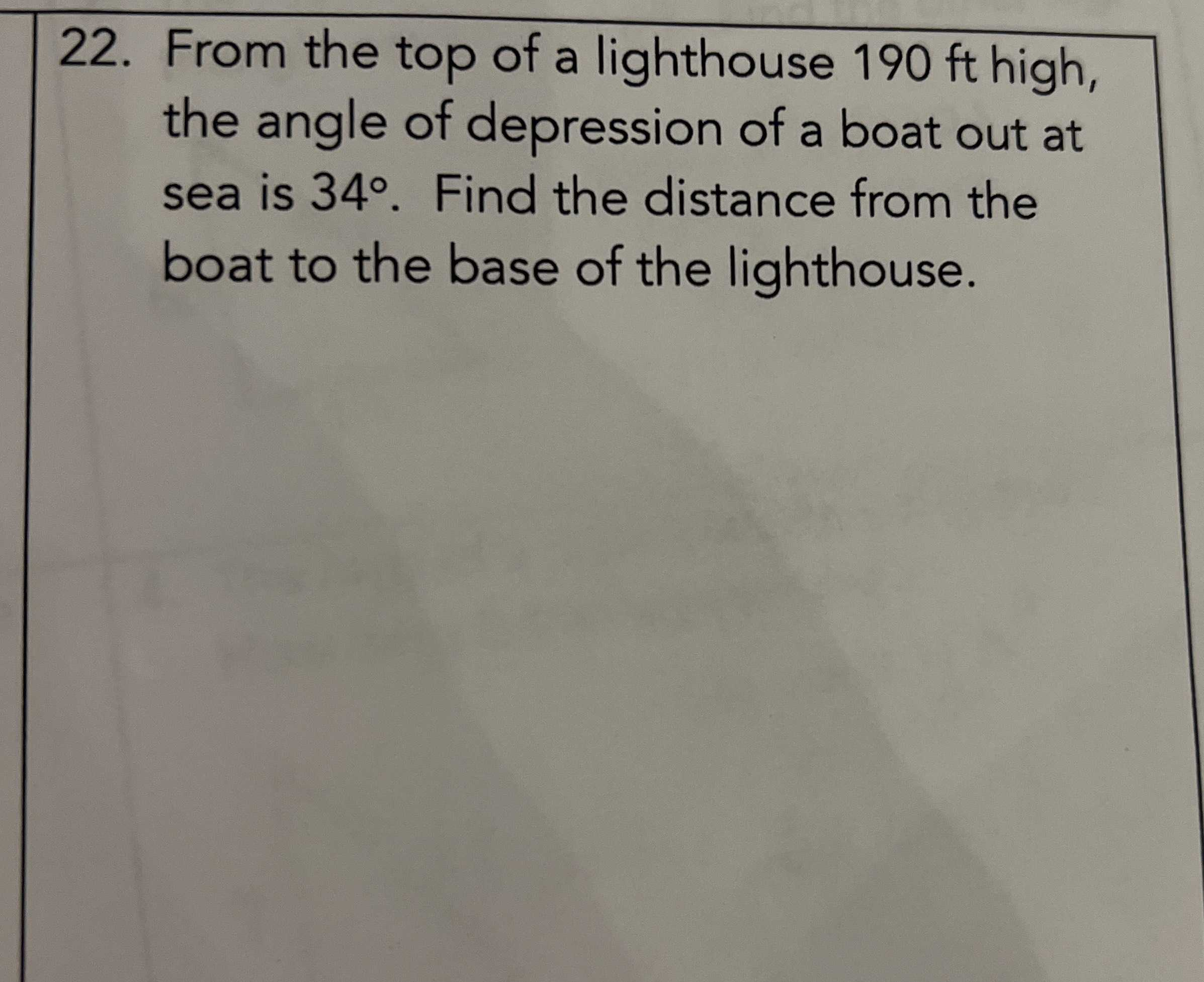From the top of a lighthouse $$190 ft$$ high, the angle of depression of a boat out at sea is $$34 ^ { \circ }$$ . Find the distance from the boat to the base of the lighthouse.

Q: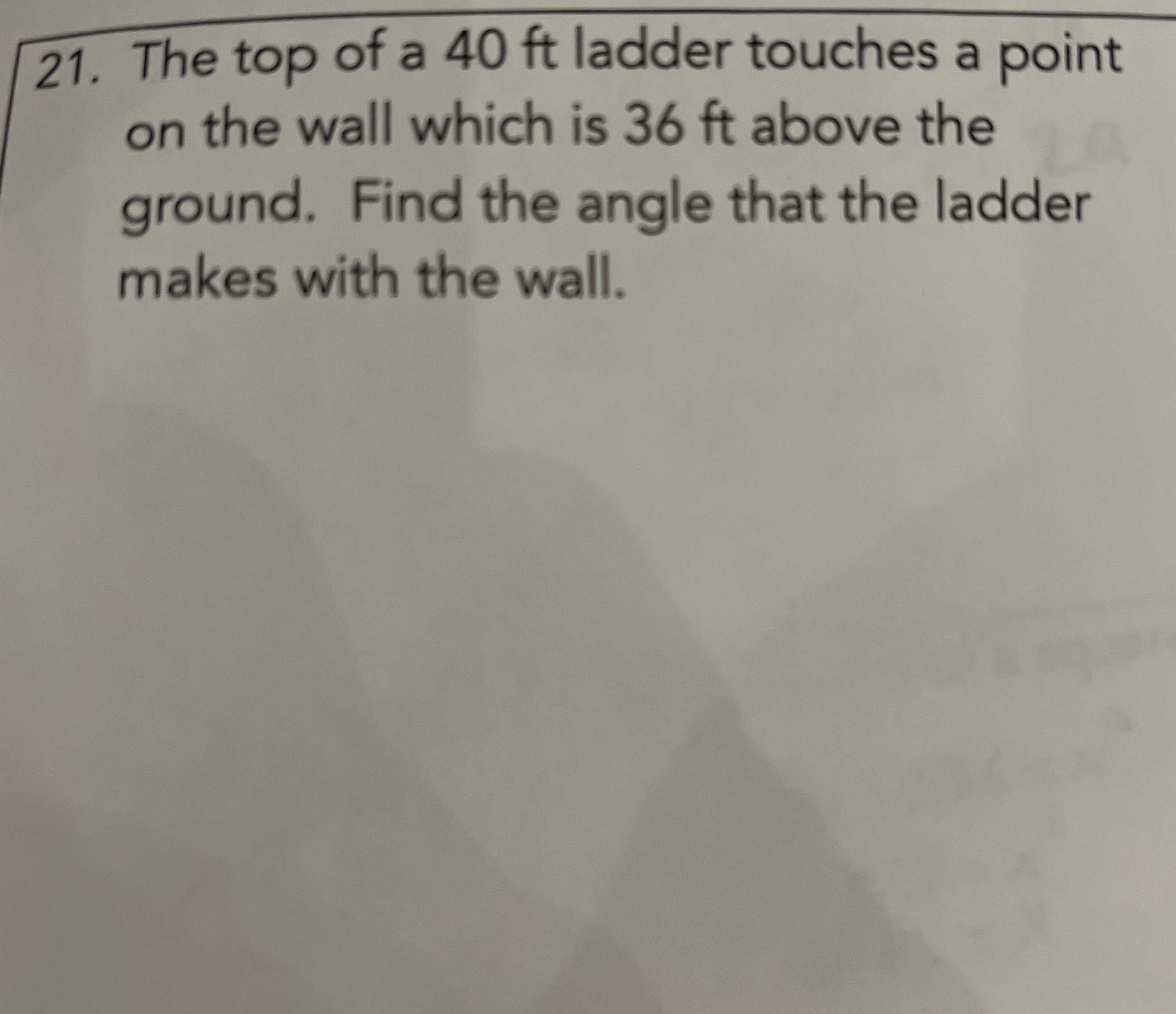The top of a $$40 ft$$ ladder touches a point on the wall which is $$36 ft$$ above the ground. Find the angle that the ladder makes with the wall.

Q: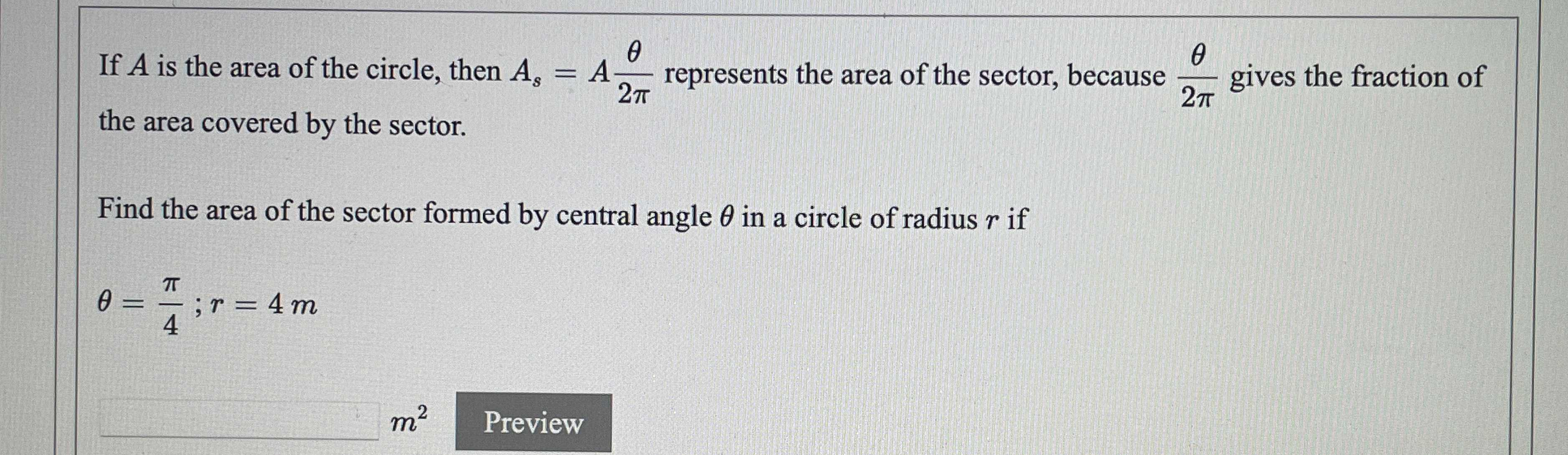If $$A$$ is the area of the circle, then $$A _ { s } = A \frac { \theta } { 2 \pi }$$ represents the area of the sector, because $$\frac { \theta } { 2 \pi }$$ gives the fraction of the area covered by the sector.

Find the area of the sector formed by central angle $$\theta$$ in a circle of radius $$r$$ if

$$\theta = \frac { \pi } { 4 } ; r = 4 m$$

Q: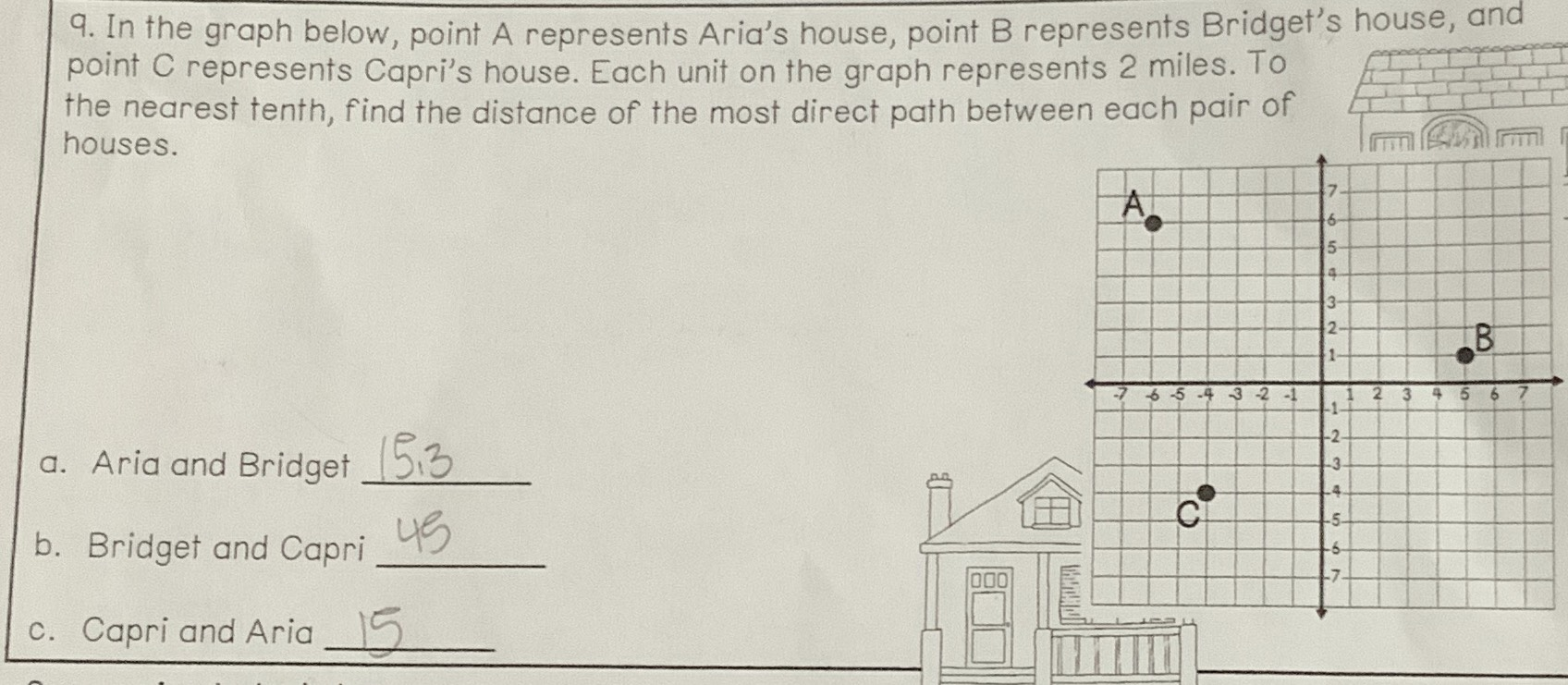point A(-6,6) represents Aria's house, point B(5,1) represents Bridget's house, and point C(-4,4) represents Capri's house. Each unit on the graph represents $$2$$ miles. To the nearest tenth, find the distance of the most direct path between each pair of houses.

a. Aria and Bridget

b. Bridget and Capri

c. Capri and Aria

Q: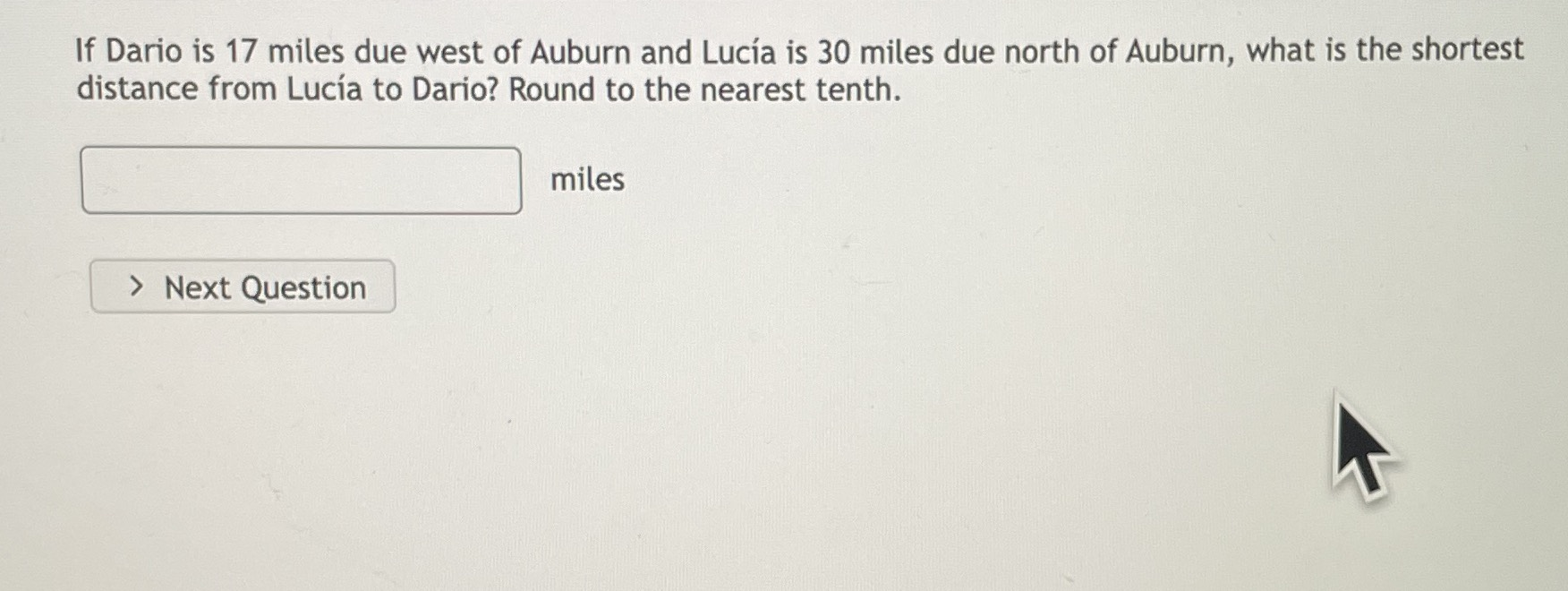If Dario is $$17$$ miles due west of Auburn and Lucía is $$30$$ miles due north of Auburn, what is the shortest distance from Lucía to Dario? Round to the nearest tenth.

Q: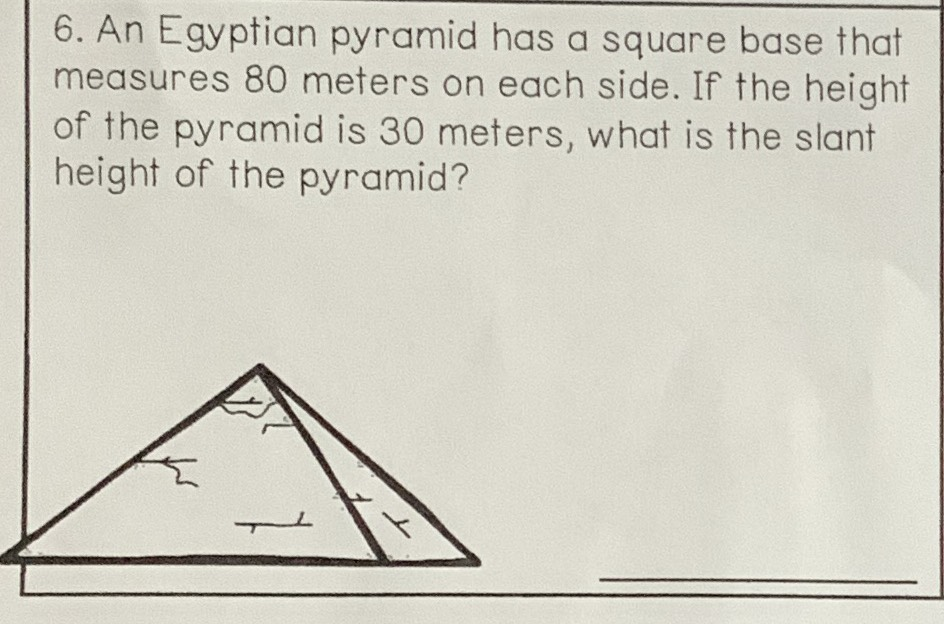An Egyptian pyramid has a square base that measures $$80$$ meters on each side. If the height of the pyramid is $$30$$ meters, what is the slant height of the pyramid?

Q: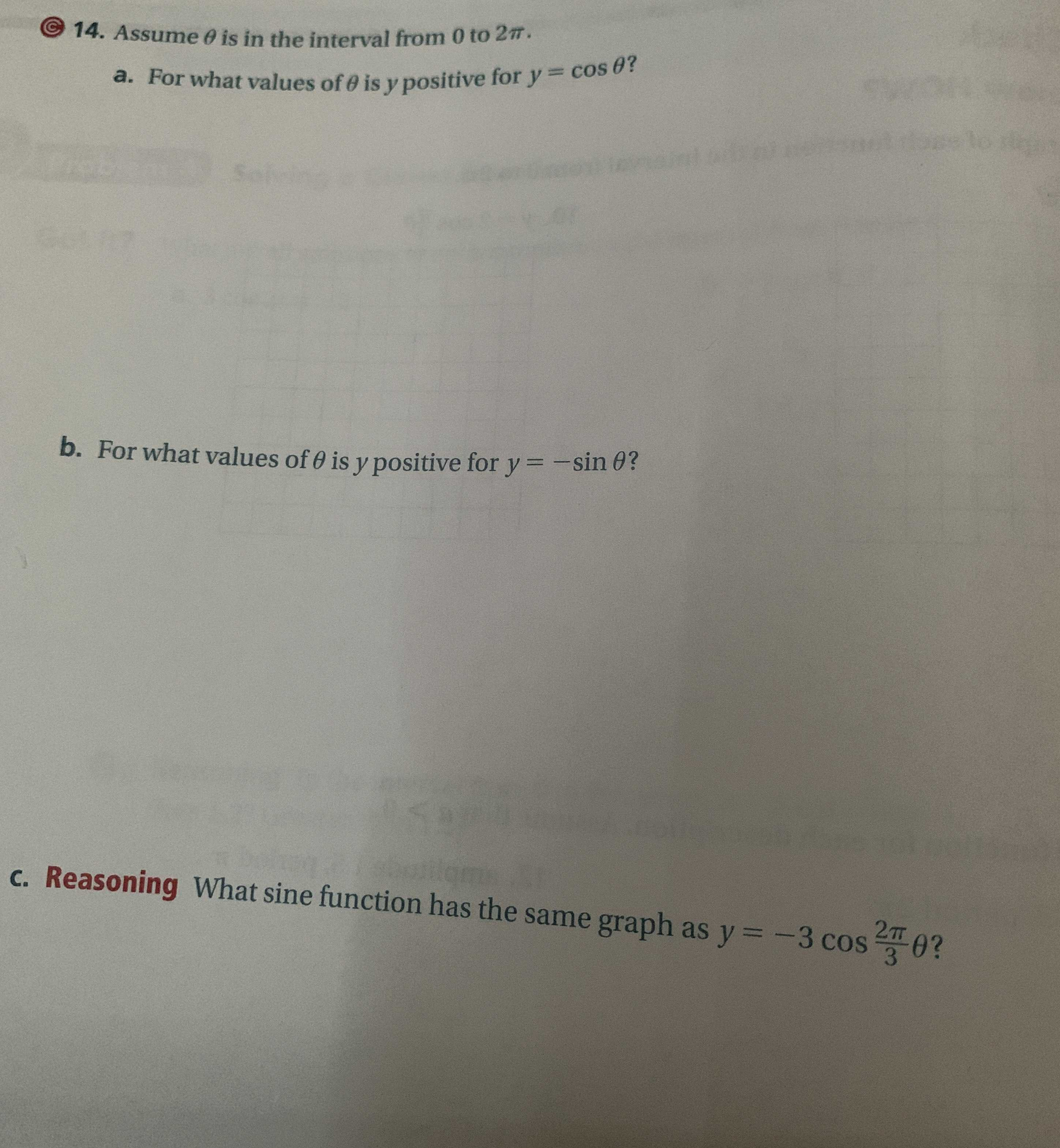Assume $$\theta$$ is in the interval from $$0$$ to $$2 \pi$$ .

a. For what values of $$\theta$$ is $$y$$ positive for $$y = \cos \theta$$ ?

b. For what values of $$\theta$$ is $$y$$ positive for $$y = - \sin \theta$$ ?

C. Reasoning What $$\operatorname { sine~function~has~the~same~graph~as~ } y = - 3 \cos \frac { 2 \pi } { 3 } \theta$$ ?

Q: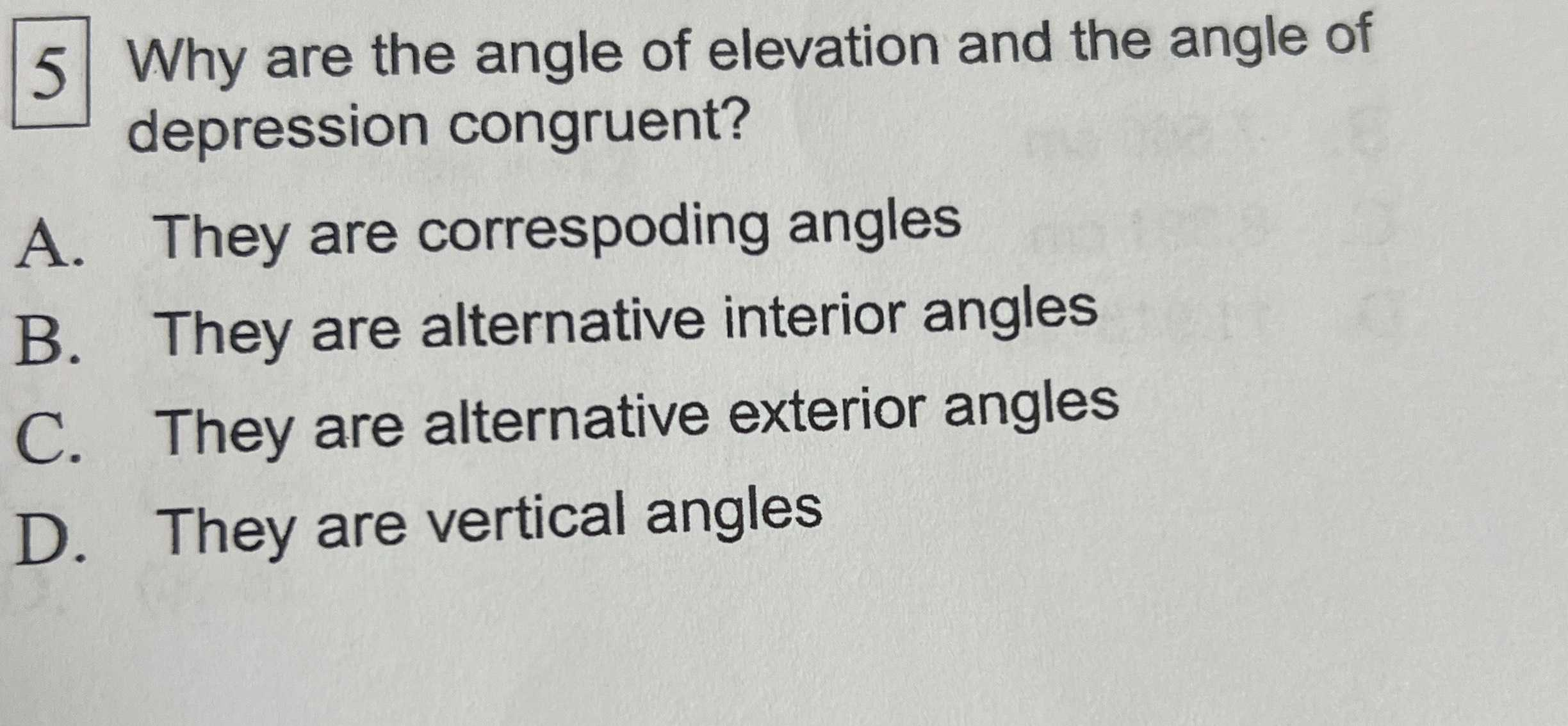Why are the angle of elevation and the angle of depression congruent?

A. They are corresponding angles

B. They are alternative interior angles

C. They are alternative exterior angles

D. They are vertical angles

Q: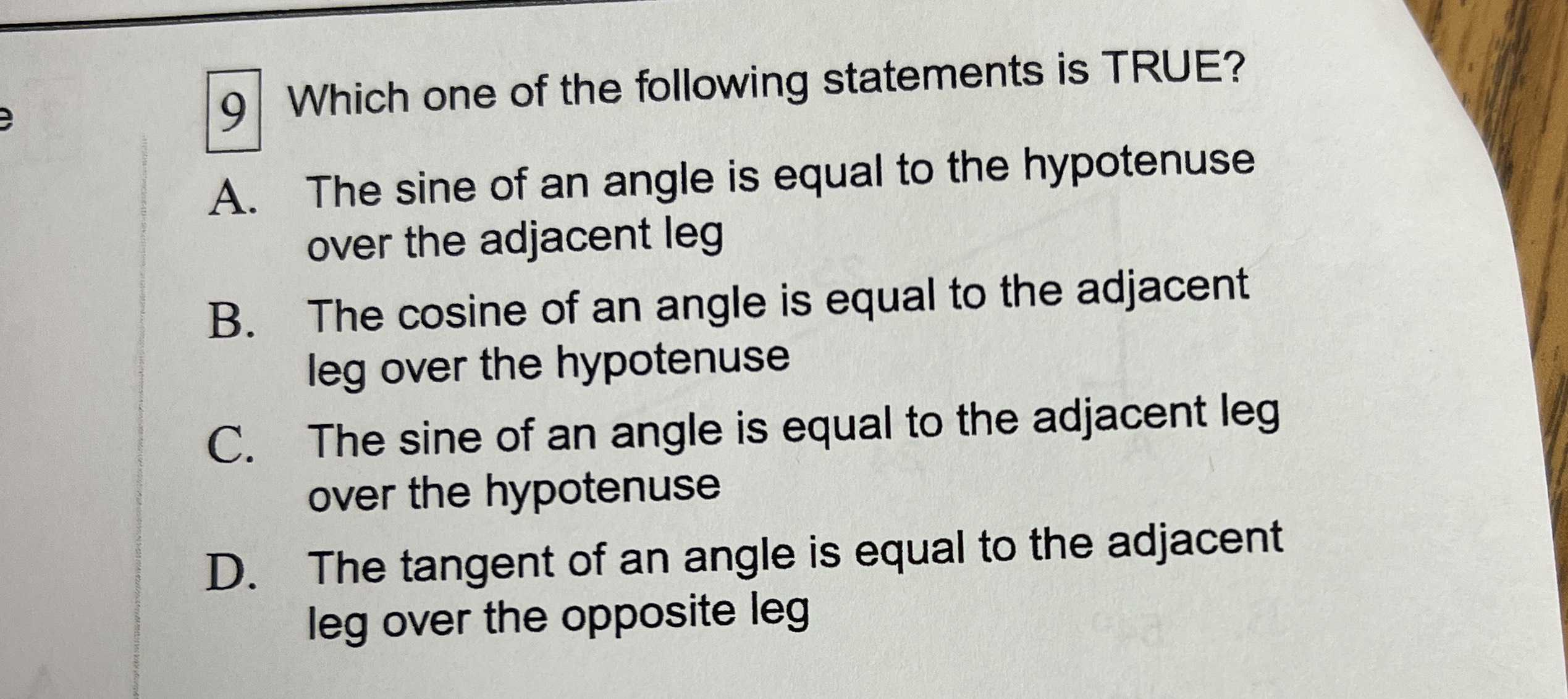Which one of the following statements is TRUE?

A. The sine of an angle is equal to the hypotenuse over the adjacent leg

B. The cosine of an angle is equal to the adjacent leg over the hypotenuse

C. The sine of an angle is equal to the adjacent leg over the hypotenuse

D. The tangent of an angle is equal to the adjacent leg over the opposite leg

Q: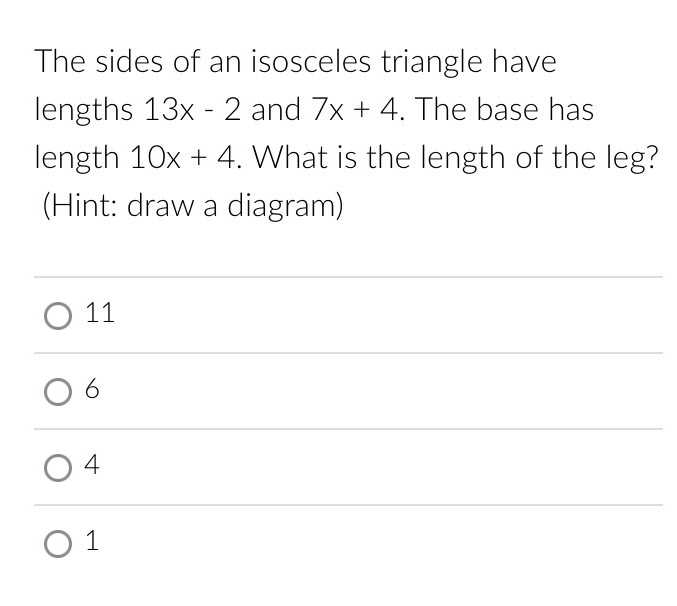The sides of an isosceles triangle have lengths $$13 x - 2$$ and $$7 x + 4$$ . The base has length $$10 x + 4$$ . What is the length of the leg? (Hint: draw a diagram)

A. $$11$$

B. $$6$$

C. $$4$$

D. $$1$$

Q: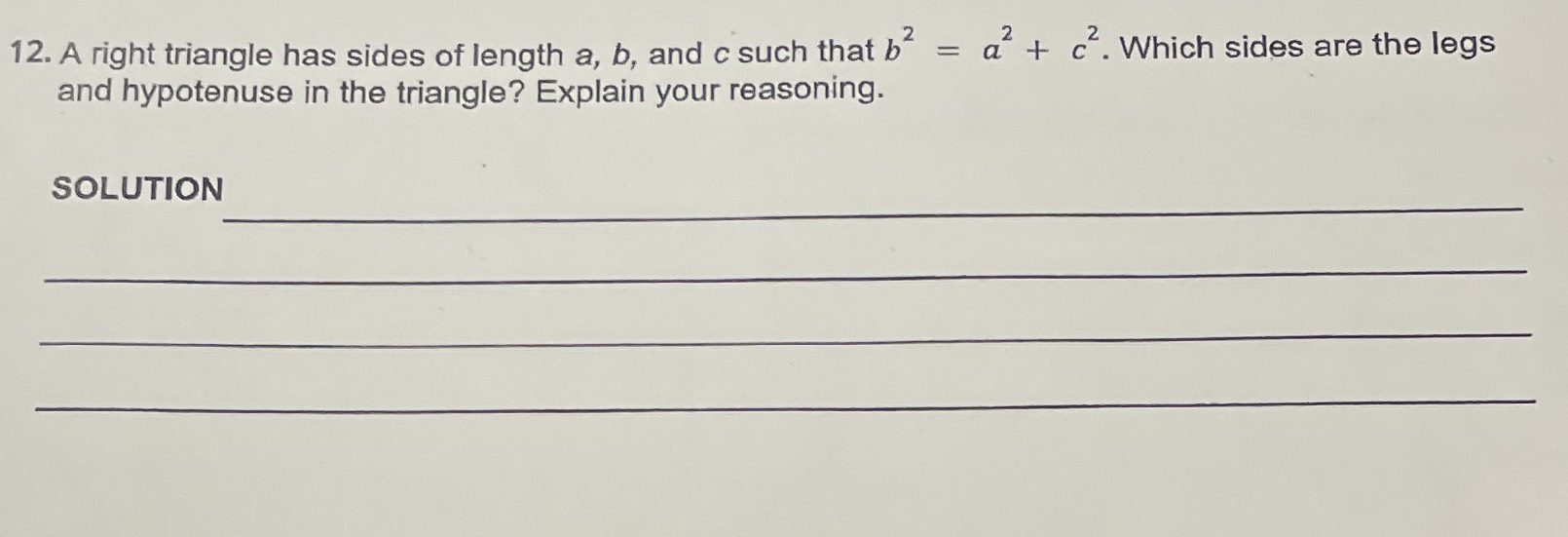A right triangle has sides of length $$a , b$$ , and $$c$$ such that $$b ^ { 2 } = a ^ { 2 } + c ^ { 2 }$$ . Which sides are the legs and hypotenuse in the triangle?

Q: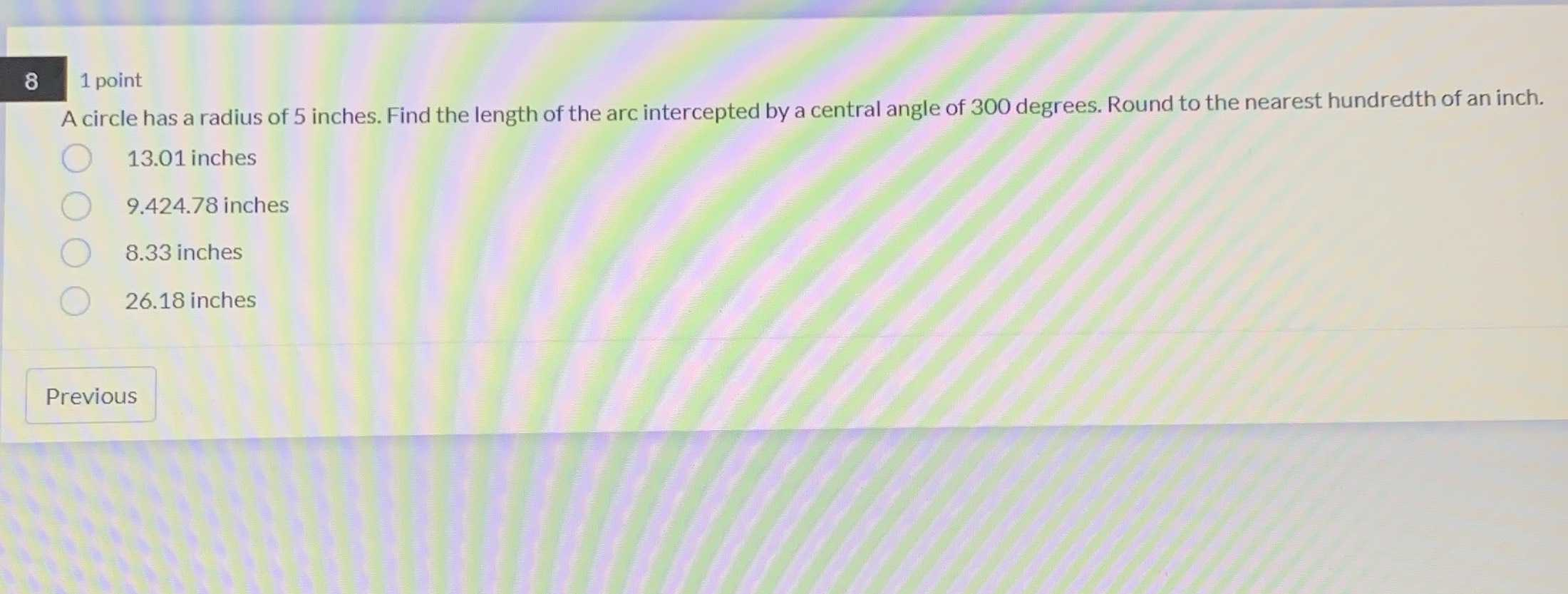A circle has a radius of $$5$$ inches. Find the length of the arc intercepted by a central angle of $$300$$ degrees. Round to the nearest hundredth of an inch.

$$13.01$$ inches

$$9.424 .78$$ inches

$$8.33$$ inches

$$26.18$$ inches Previous

Q: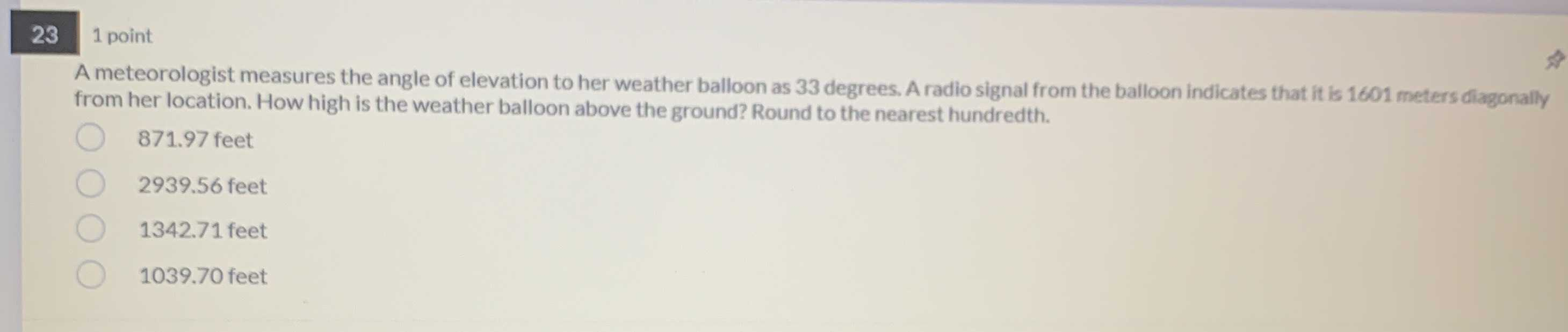A meteorologist measures the angle of elevation to her weather balloon as $$33$$ degrees. A radio signal from the balloon indicates that it is $$1601$$ meters diagonally from her location. How high is the weather balloon above the ground? Round to the nearest hundredth.

$$871.97$$ feet

$$2939.56$$ feet

$$1342.71$$ feet

$$1039.70$$ feet

Q: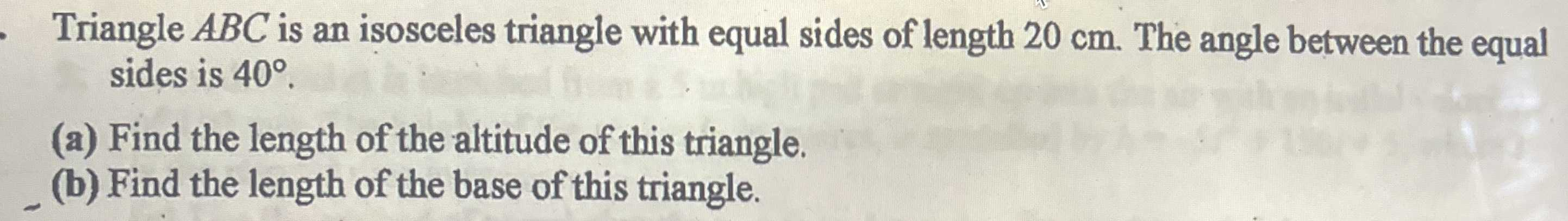Triangle $$A B C$$ is an isosceles triangle with equal sides of length $$20 cm$$ . The angle between the equal sides is $$40 ^ { \circ }$$ .

(a) Find the length of the altitude of this triangle.

(b) Find the length of the base of this triangle.

Q: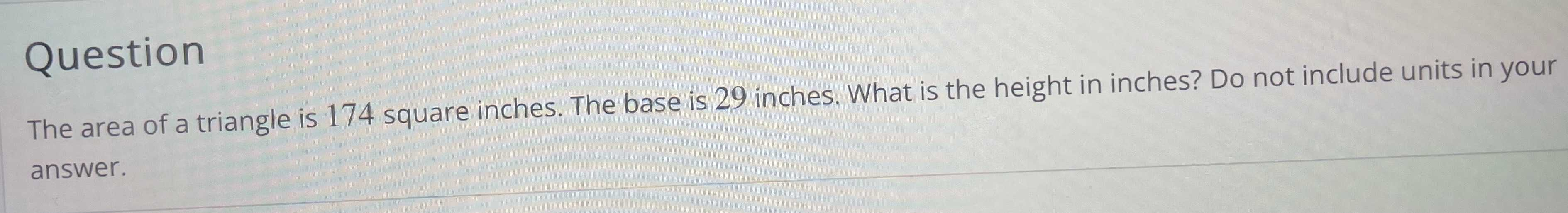The area of a triangle is $$174$$ square inches. The base is $$29$$ inches. What is the height in inches? Do not include units in your answer.

Q: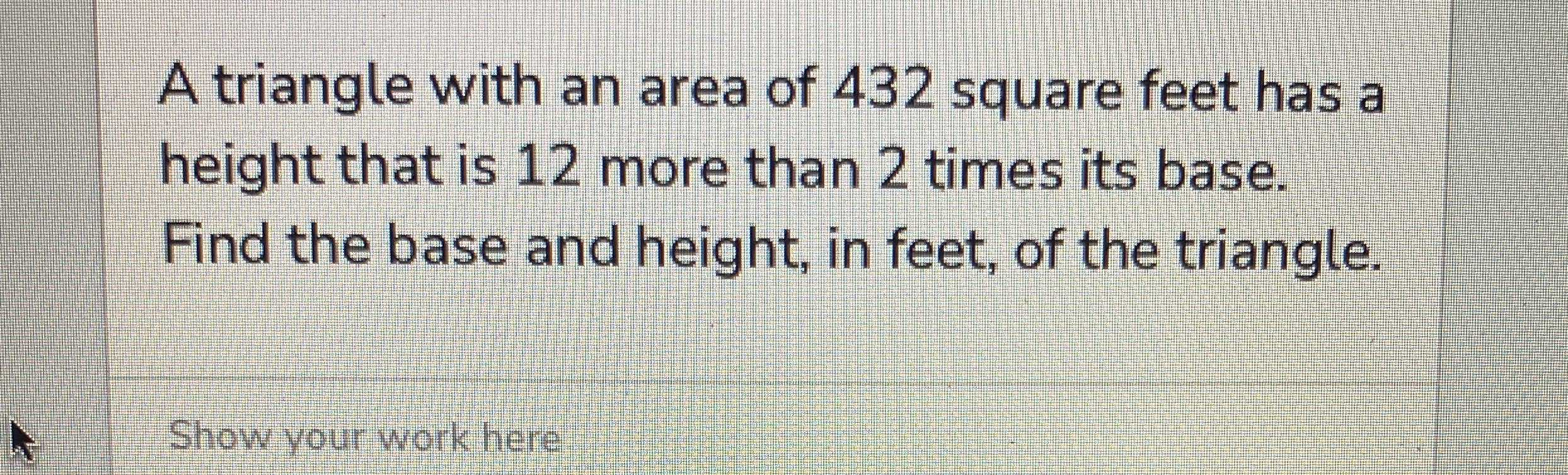A triangle with an area of $$432$$ square feet has a height that is $$12$$ more than $$2$$ times its base. Find the base and height, in feet, of the triangle.

Q: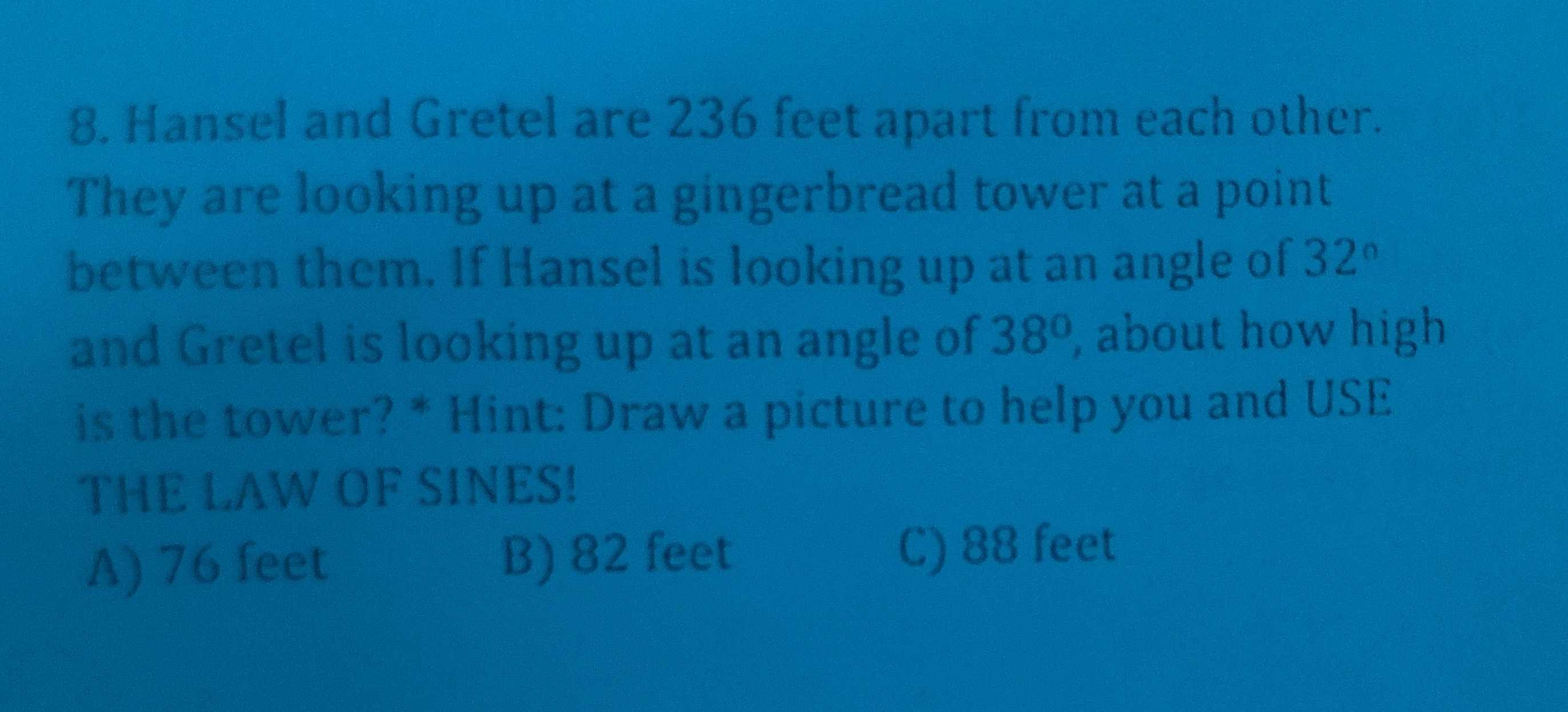Hansel and Gretel are $$236$$ feet apart from each other. They are looking up at a gingerbread tower at a point between them. If Hansel is looking up at an angle of $$32 ^ { \circ }$$ and Gretel is looking up at an angle of $$38 ^ { \circ }$$ , about how high is the tower? * Hint: Draw a picture to help you and USE THE LAW OF SINES!

A) $$76$$ feet                      B) $$82$$ feet                       C) $$88$$ feet

Q: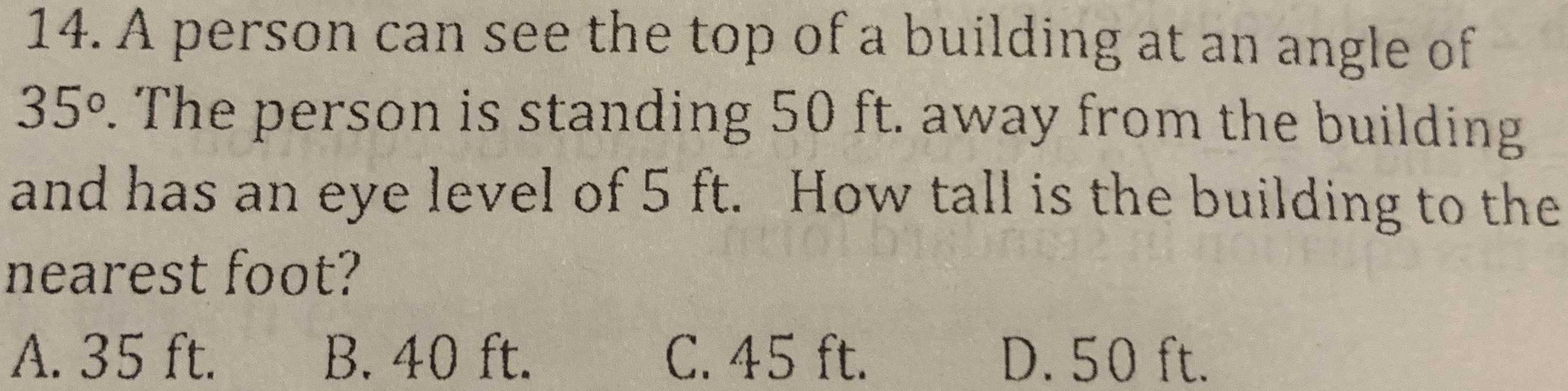A person can see the top of a building at an angle of $$35 ^ { \circ }$$ . The person is standing $$50 ft$$ . away from the building and has an eye level of $$5 ft$$ . How tall is the building to the nearest foot?

A. $$35 ft$$ .      B. $$40 ft$$       C. $$45 ft$$        D. $$50 ft$$

Q: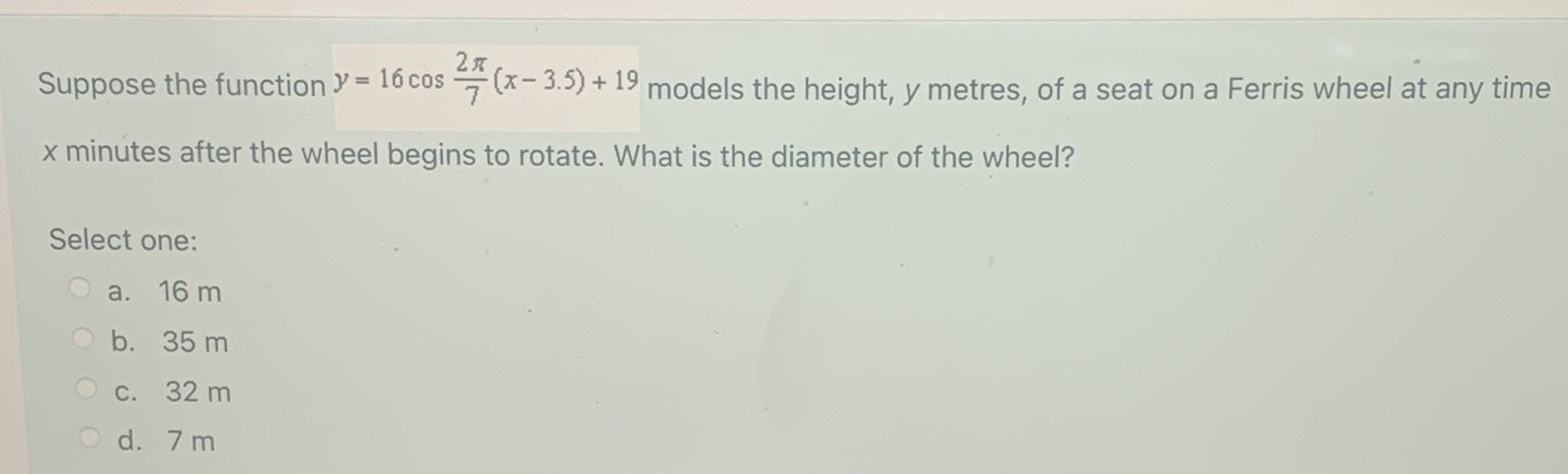Suppose the function $$y = 16 \cos \frac { 2 \pi } { 7 } ( x - 3.5 ) + 19$$ models the height, $$y$$ metres, of a seat on a Ferris wheel at any time $$x$$ minutes after the wheel begins to rotate. What is the diameter of the wheel?

Select one: a. $$16 m$$ b. $$35 m$$ c. $$32 m$$ d. $$7 m$$

Q: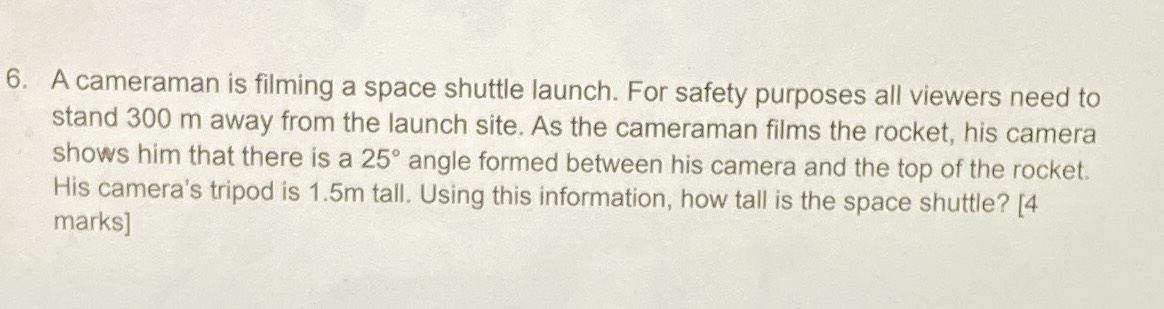A cameraman is filming a space shuttle launch. For safety purposes all viewers need to stand $$300 m$$ away from the launch site. As the cameraman films the rocket, his camera shows him that there is a $$25 ^ { \circ }$$ angle formed between his camera and the top of the rocket. His camera's tripod is $$1.5 m$$ tall. Using this information, how tall is the space shuttle?

Q:A bottle is riding the waves at a beach. The bottle's up and down motion with the waves can be described using the formula $$h = 1.4 \sin ( \frac { \pi t } { 3 } )$$ , where $$h$$ is the height, in metres, above the flat water surface and $$t$$ is the time, in seconds. When is the first time, to the nearest tenth of a second, that the height of the bottle will be $$1.4 m$$ ?

Select one:

a. $$1.5 s$$

b. $$0.9 s$$

c. $$1.1 s$$

d. $$14.8 s$$

Q: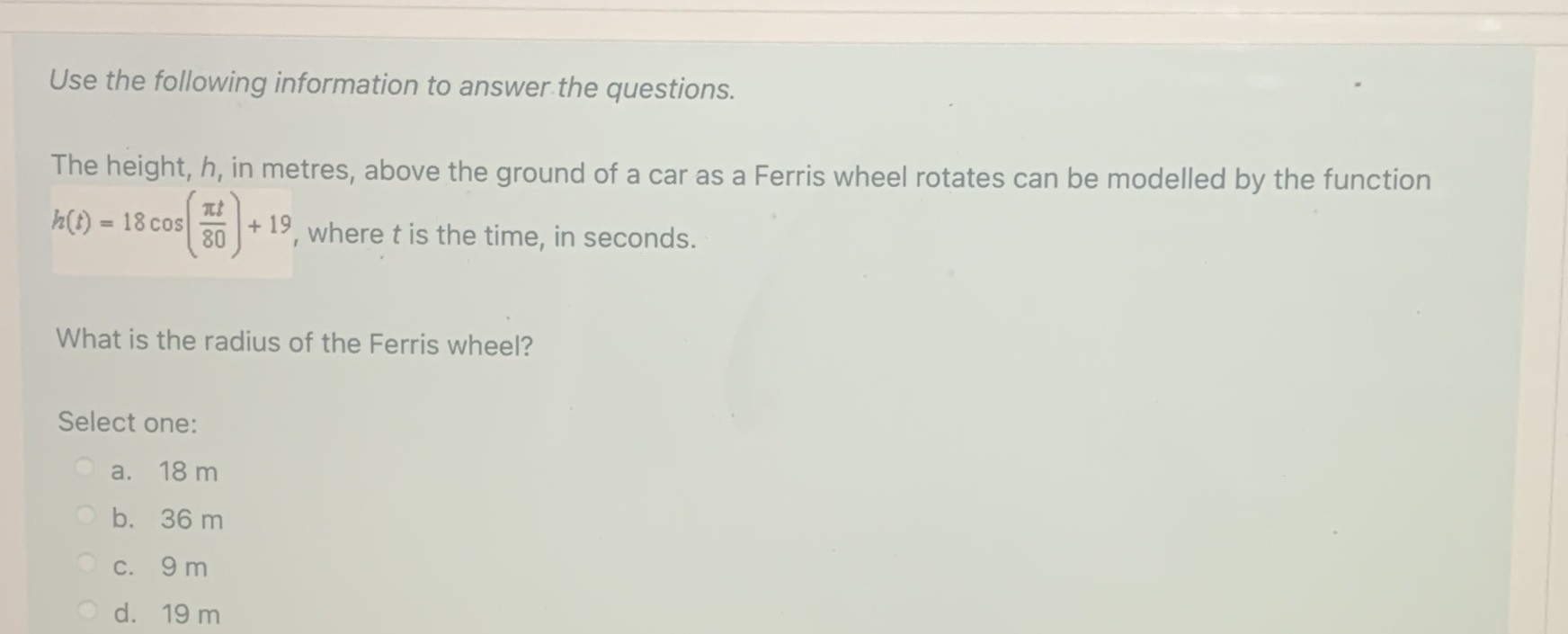Use the following information to answer the questions.

The height, $$h$$ , in metres, above the ground of a car as a Ferris wheel rotates can be modelled by the function $$h ( t ) = 18 \cos ( \frac { \pi t } { 80 } ) + 19$$ , where $$t$$ is the time, in seconds.

What is the radius of the Ferris wheel?

Select one:

a. $$18 m$$

b. $$36 m$$

c. $$9 m$$

d. $$19 m$$

Q: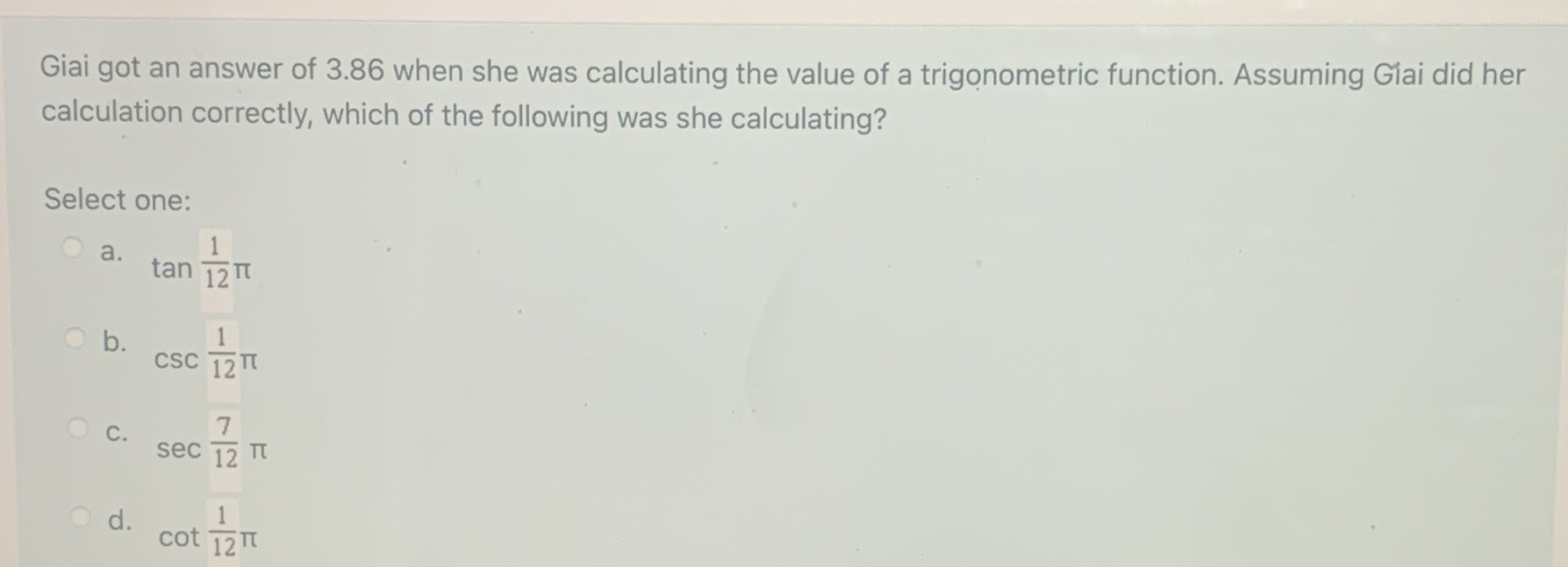Giai got an answer of $$3.86$$ when she was calculating the value of a trigonometric function. Assuming Giai did her calculation correctly, which of the following was she calculating?

Select one:

a. $$\tan \frac { 1 } { 12 } \pi$$

b. $$\csc \frac { 1 } { 12 } \pi$$

c. $$\sec \frac { 7 } { 12 } \pi$$

d. $$\cot \frac { 1 } { 12 } \pi$$

Q: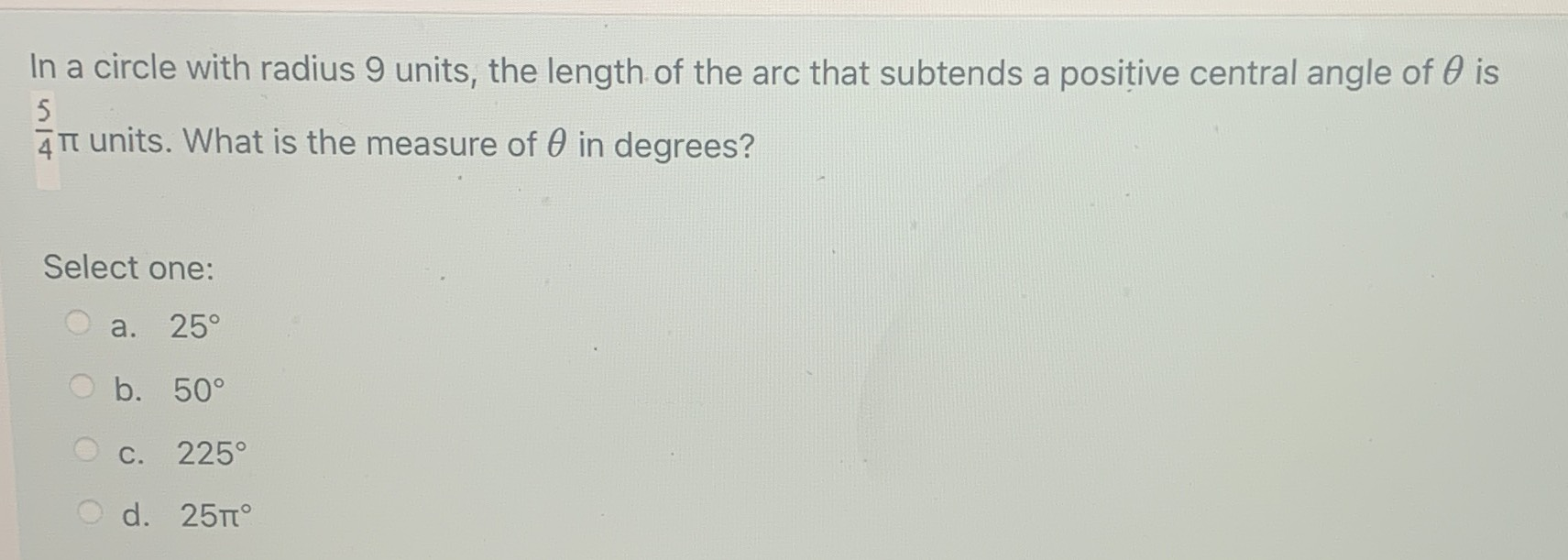In a circle with radius $$9$$ units, the length of the arc that subtends a positive central angle of $$\theta$$ is $$\frac { 5 } { 4 } \pi$$ units. What is the measure of $$\theta$$ in degrees?

Select one:

a. $$25 ^ { \circ }$$

b. $$50 ^ { \circ }$$

c. $$225 ^ { \circ }$$

d. $$25 \pi ^ { \circ }$$

Q: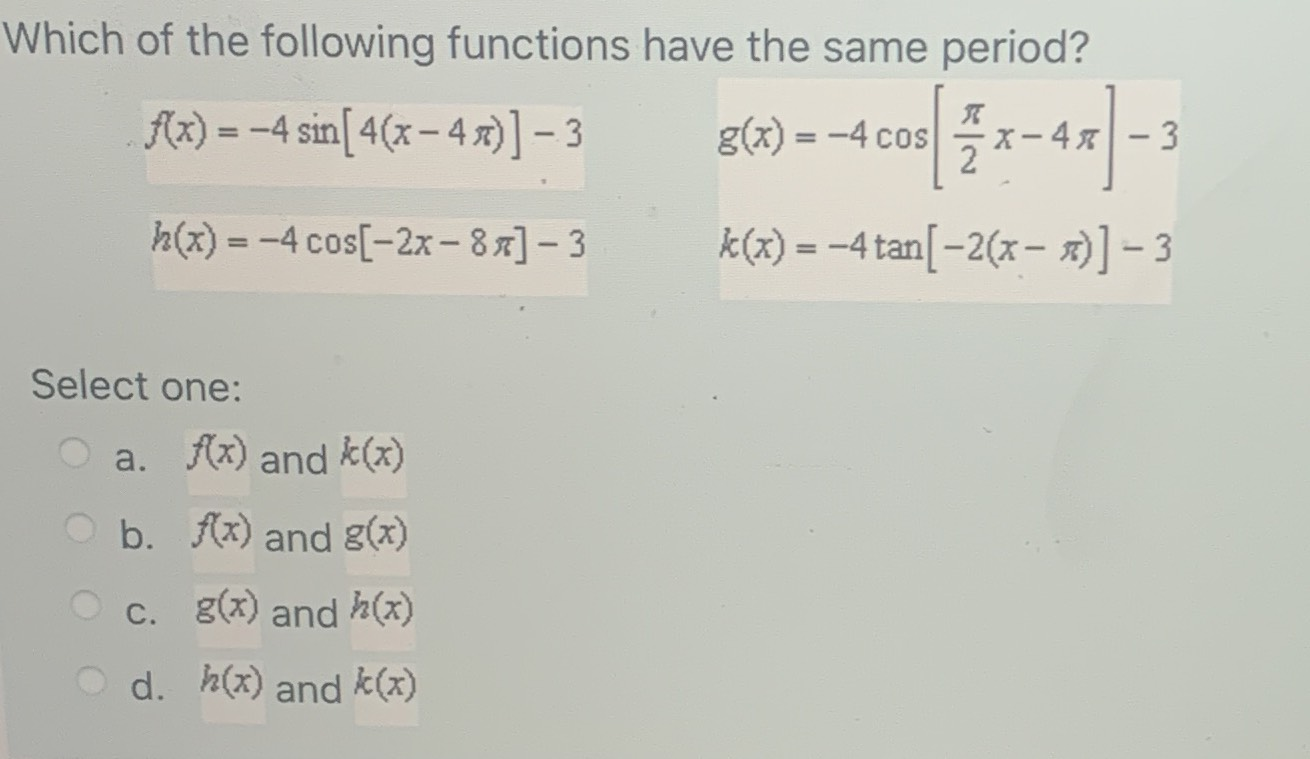Which of the following functions have the same period?

$$f ( x ) = - 4 \sin [ 4 ( x - 4 \pi ) ] - 3 g ( x ) = - 4 \cos [ \frac { \pi } { 2 } x - 4 \pi ] - 3$$

$$h ( x ) = - 4 \cos [ - 2 x - 8 \pi ] - 3 k ( x ) = - 4 \tan [ - 2 ( x - \pi ) ] - 3$$

Select one:

a. $$f ( x )$$ and $$k ( x )$$

b. $$f ( x )$$ and $$g ( x )$$

c. $$g ( x )$$ and $$h ( x )$$

d. $$h ( x )$$ and $$k ( x )$$

Q: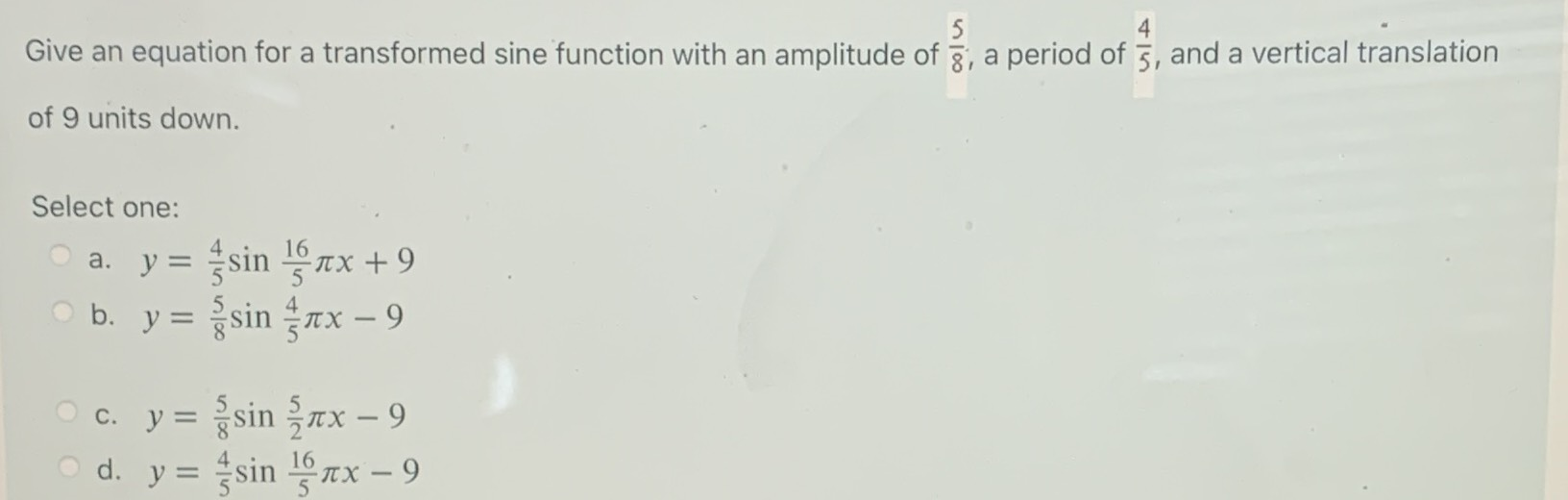Give an equation for a transformed sine function with an amplitude of $$\frac { 5 } { 8 }$$ , a period of $$\frac { 4 } { 5 }$$ , and a vertical translation of $$9$$ units down.

Select one:

a. $$y = \frac { 4 } { 5 } \sin \frac { 16 } { 5 } \pi x + 9$$

b. $$y = \frac { 5 } { 8 } \sin \frac { 4 } { 5 } \pi x - 9$$

c. $$y = \frac { 5 } { 8 } \sin \frac { 5 } { 2 } \pi x - 9$$

d. $$y = \frac { 4 } { 5 } \sin \frac { 16 } { 5 } \pi x - 9$$

Q: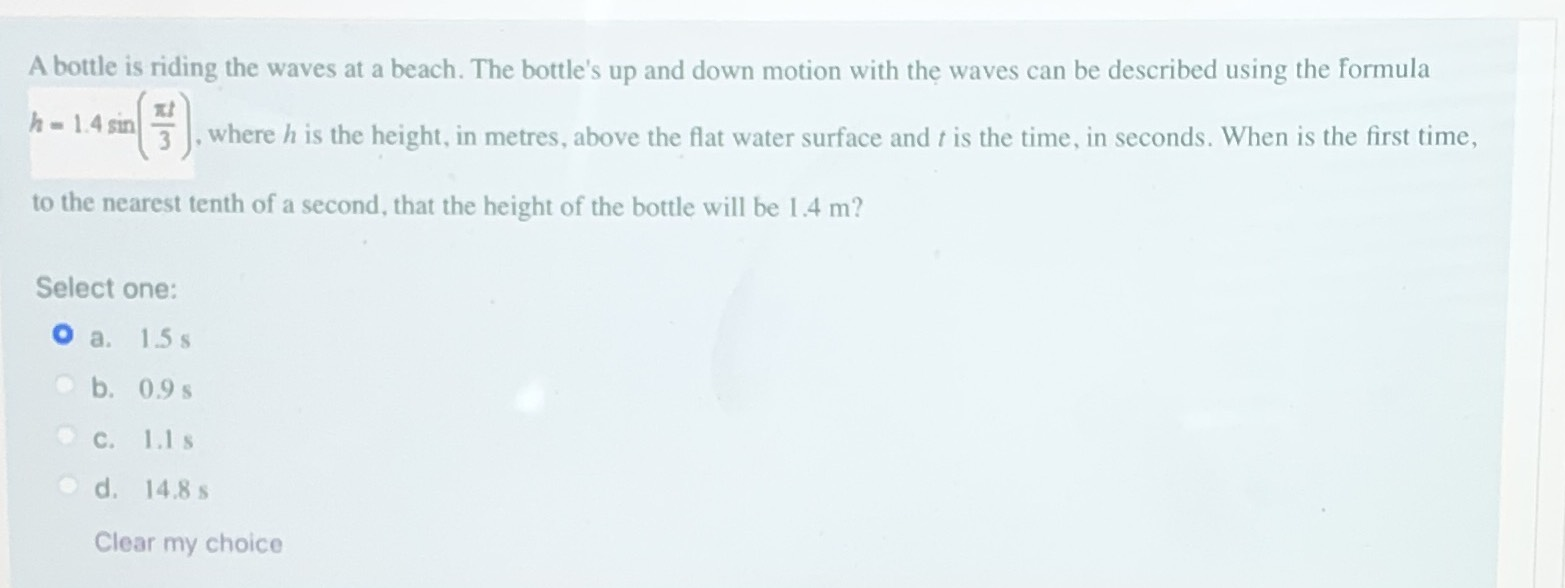A bottle is riding the waves at a beach. The bottle's up and down motion with the waves can be described using the formula $$h = 1.4 \sin ( \frac { \pi t } { 3 } )$$ , where $$h$$ is the height, in metres, above the flat water surface and $$t$$ is the time, in seconds. When is the first time, to the nearest tenth of a second, that the height of the bottle will be $$1.4 m$$ ?

Select one:

a. $$1.5 s$$

b. $$0.9 s$$

c. $$1.1 s$$

d. $$14.8 s$$

Q: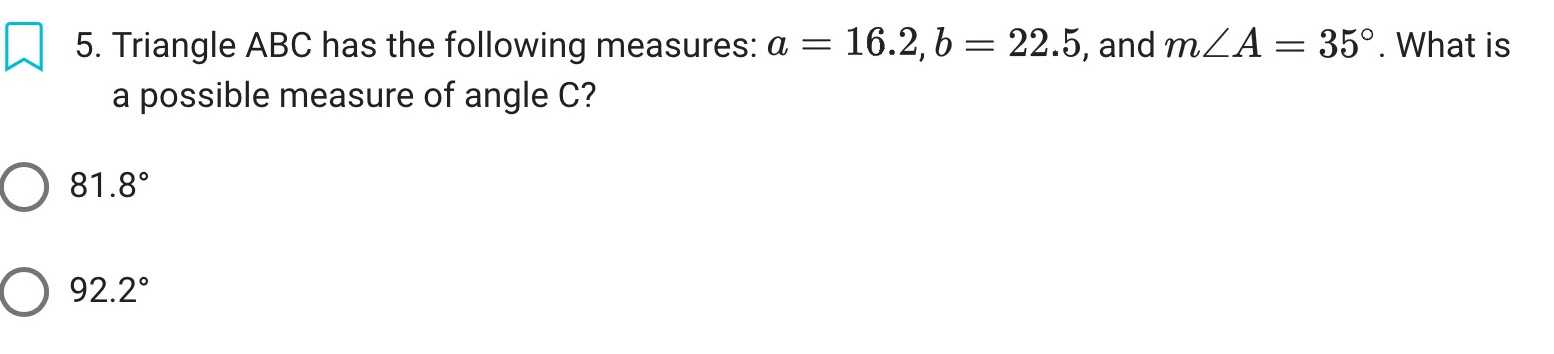Triangle $$ABC$$ has the following measures: $$a = 16.2 , b = 22.5$$ , and $$m \angle A = 35 ^ { \circ }$$ . What is a possible measure of angle $$C$$ ?

$$81.8 ^ { \circ }$$

$$92.2 ^ { \circ }$$

Q: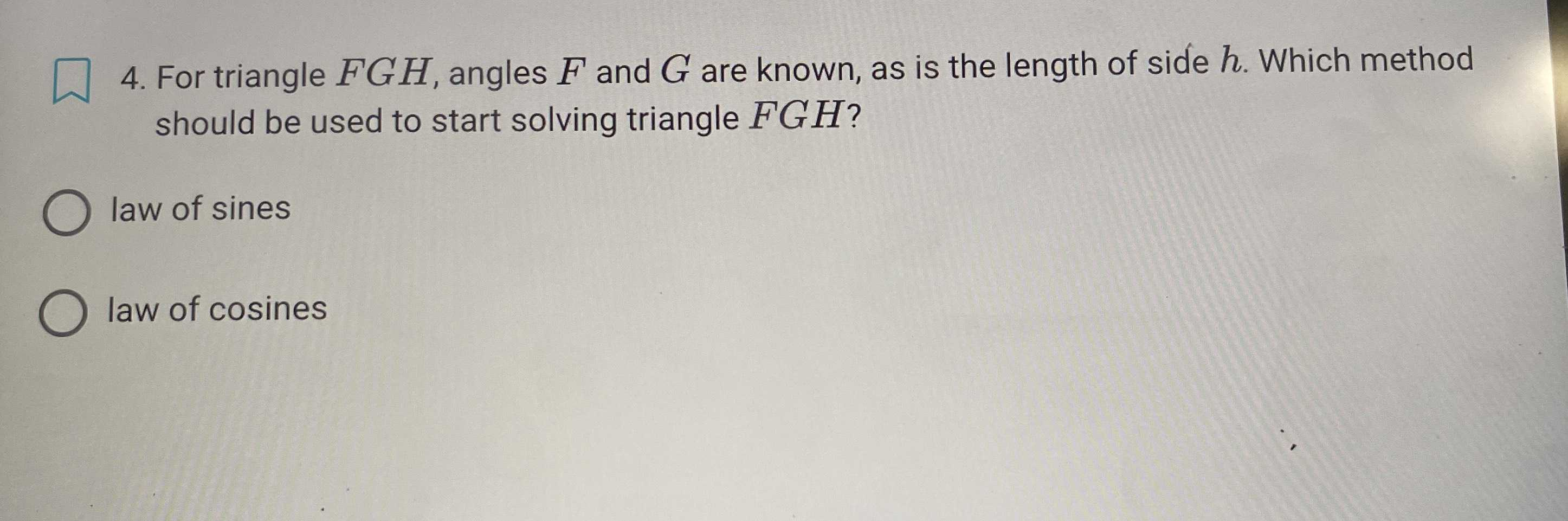For triangle $$F G H$$ , angles $$F$$ and $$G$$ are known, as is the length of side $$h$$ . Which method should be used to start solving triangle $$F G H ?$$

law of sines

law of cosines

Q:

$$\left. \begin{array} { l } { \text { 3. Create a diagram for each of the following scenarios. } } \\ { \text { a) Keisha is helping her neighbour move. The ramp to the moving } } \\ { \text { truck is } 3 m \text { long, and makes an angle of } 32 ^ { \circ } \text { with the ground. } } \\ { \text { Keisha wants to know if she can lift boxes from the ground } } \\ { \text { into the truck without using the ramp. How high is the loading } } \\ { \text { door of the truck off the ground? } } \\ { \text { b) Aaron is a leisure pilot. His plane takes off from an airport at } } \\ { \text { an angle of } 6 ^ { \circ } \text { with the ground. He flies } 5.4 km \text { until he is over } } \end{array} \right.$$

Create a diagram for each of the following scenarios. a) Keisha is helping her neighbour move. The ramp to the moving truck is $$3 m$$ long, and makes an angle of $$32 ^ { \circ }$$ with the ground. Keisha wants to know if she can lift boxes from the ground into the truck without using the ramp. How high is the loading door of the truck off the ground? b) Aaron is a leisure pilot. His plane takes off from an airport at an angle of $$6 ^ { \circ }$$ with the ground. He flies $$5.4 km$$ until he is over

Q: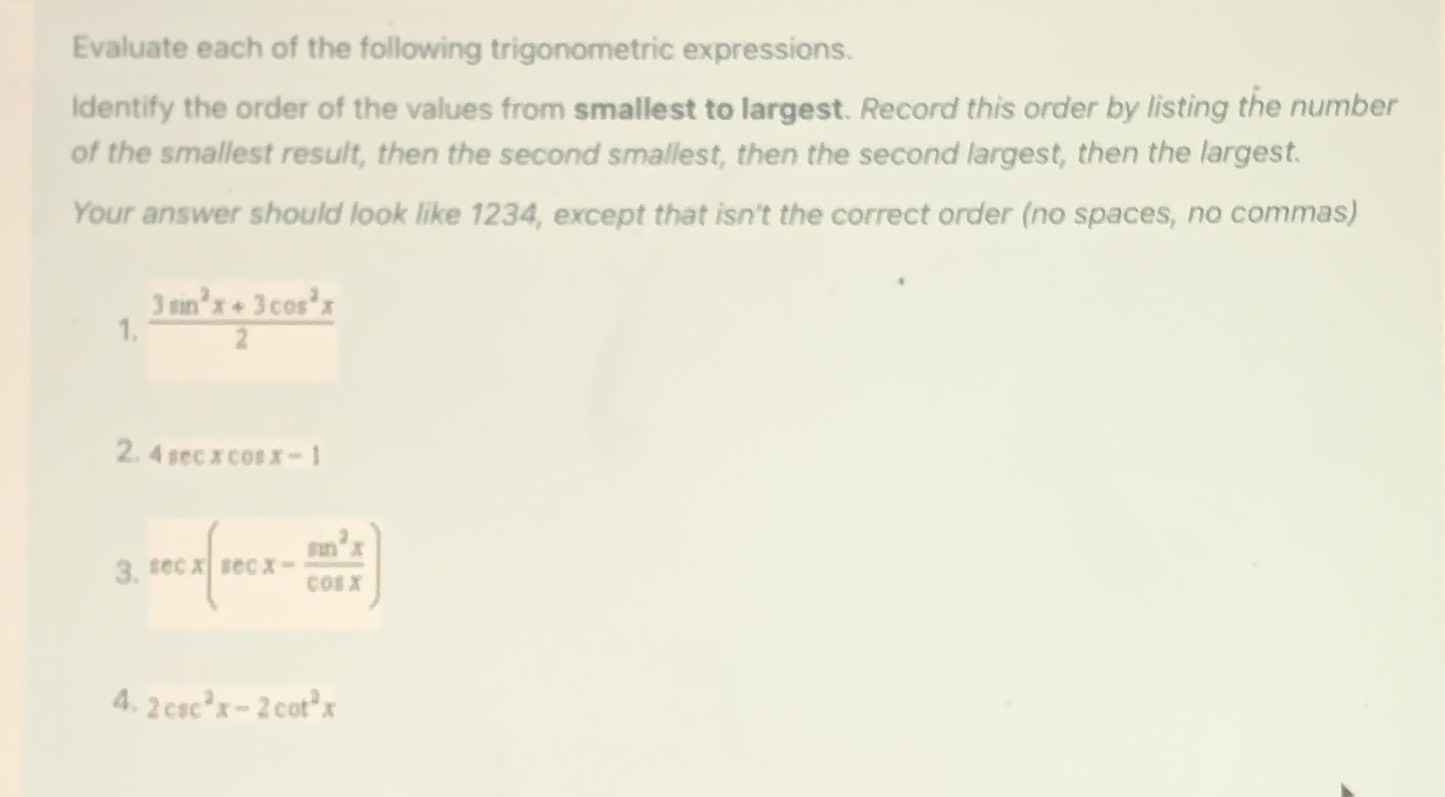Evaluate each of the following trigonometric expressions. Identify the order of the values from smallest to largest. Record this order by listing the number of the smallest result, then the second smallest, then the second largest, then the largest. Your answer should look like 1234, except that isn't the correct order (no spaces, no commas)

1. $$\frac { 3 \sin ^ { 2 } x + 3 \cos ^ { 2 } x } { 2 }$$

2. $$4 \sec x \cos x - 1$$

3. $$\sec x ( \sec x - \frac { \sin ^ { 2 } x } { \cos ^ { 2 } x } )$$

4. $$2 \csc ^ { 2 } x - 2 \cot ^ { 2 } x$$

Q: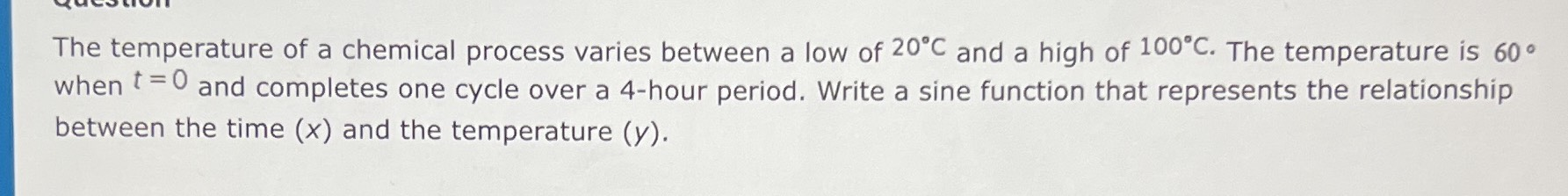The temperature of a chemical process varies between a low of $$20 ^ { \circ } C$$ and a high of $$100 ^ { \circ } C$$ . The temperature is $$60 ^ { \circ }$$

when $$t = 0$$ and completes one cycle over a $$4$$ -hour period. Write a sine function that represents the relationship between the time $$( x )$$ and the temperature $$( y )$$ .

Q: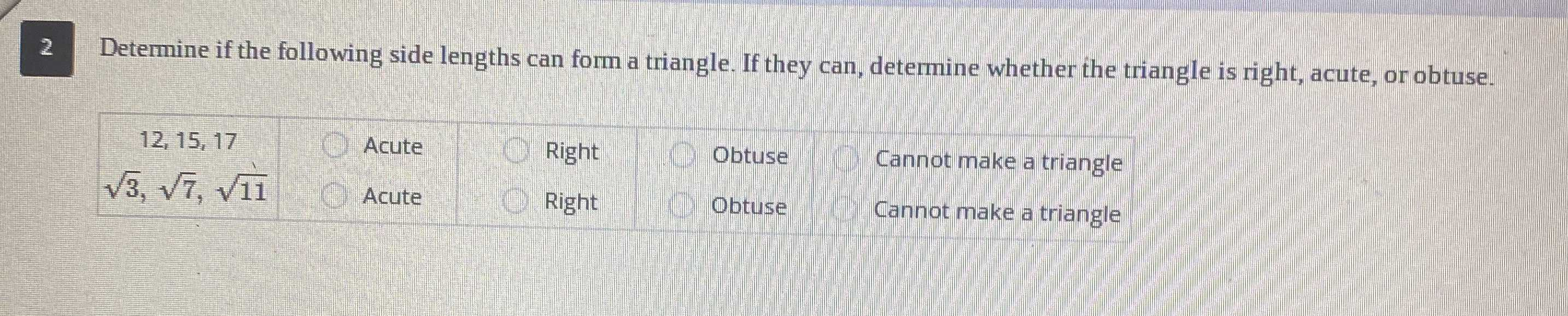Determine if the following side lengths can form a triangle. If they can, determine whether the triangle is right, acute, or obtuse.

1. $$12,15,17$$

A. Acute

B. Right

C. Obutuse

D. Cannot make a triangle

2. $$\sqrt { 3 } , \sqrt { 7 } , \sqrt { 11 }$$

A. Acute

B. Right

C. Obutuse

D. Cannot make a triangle

Q: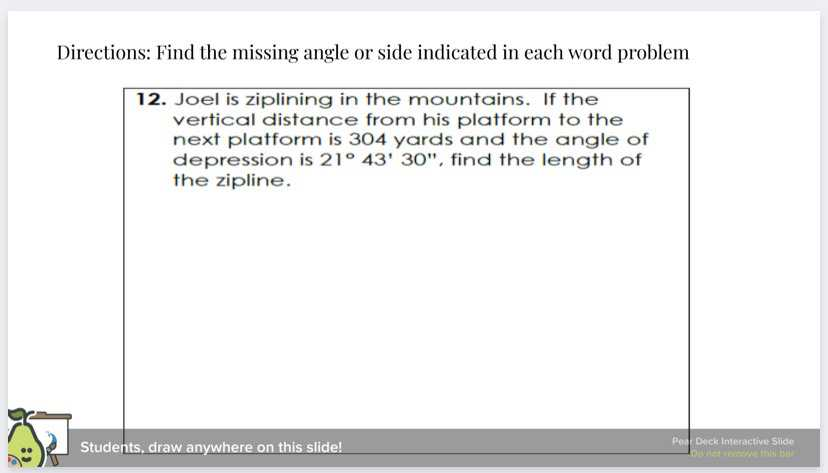Joel is ziplining in the mountains. If the vertical distance from his platform to the next platform is $$304$$ yards and the angle of depression is $$21 \circ 43 ^ { \circ } 30 ^ { \prime \prime }$$ , find the length of the zipline.

Q: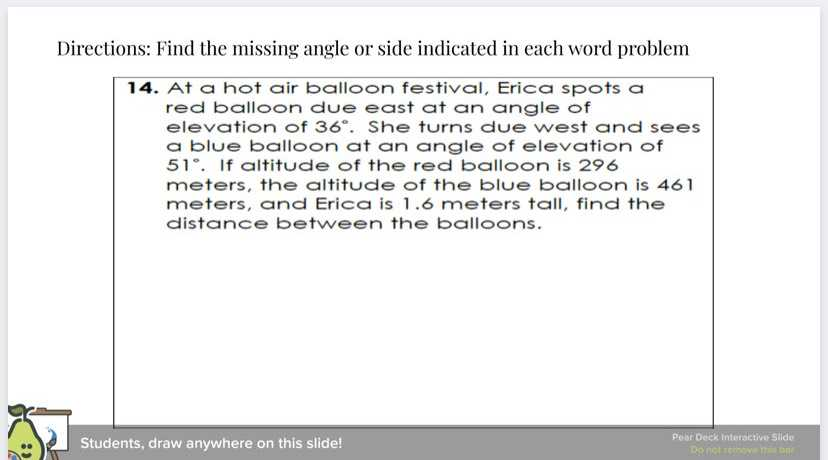At a hot air balloon festival, Erica spots a red balloon due east at an angle of elevation of $$36 ^ { \circ }$$ . She turns due west and sees a blue balloon at an angle of elevation of $$51 ^ { \circ }$$ . If altitude of the red balloon is $$296$$ meters, the altitude of the blue balloon is $$461$$ meters, and Erica is $$1.6$$ meters tall, find the distance between the balloons.

Q: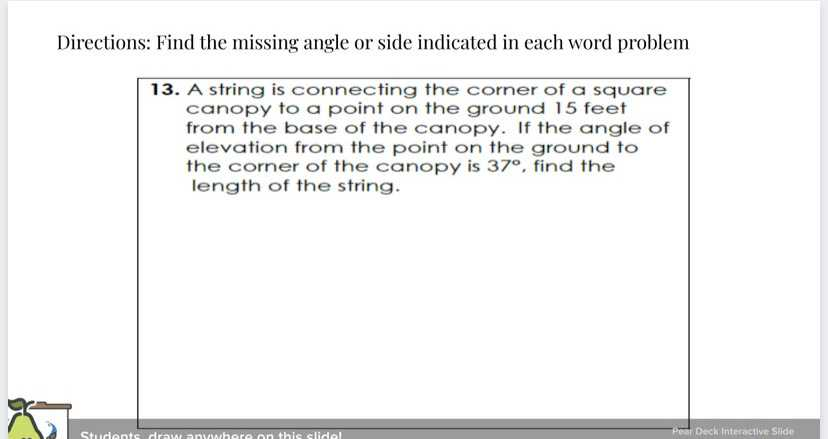A string is connecting the corner of a square canopy to a point on the ground $$15$$ feet from the base of the canopy. If the angle of elevation from the point on the ground to the corner of the canopy is $$37 ^ { \circ }$$ , find the length of the string.

Q: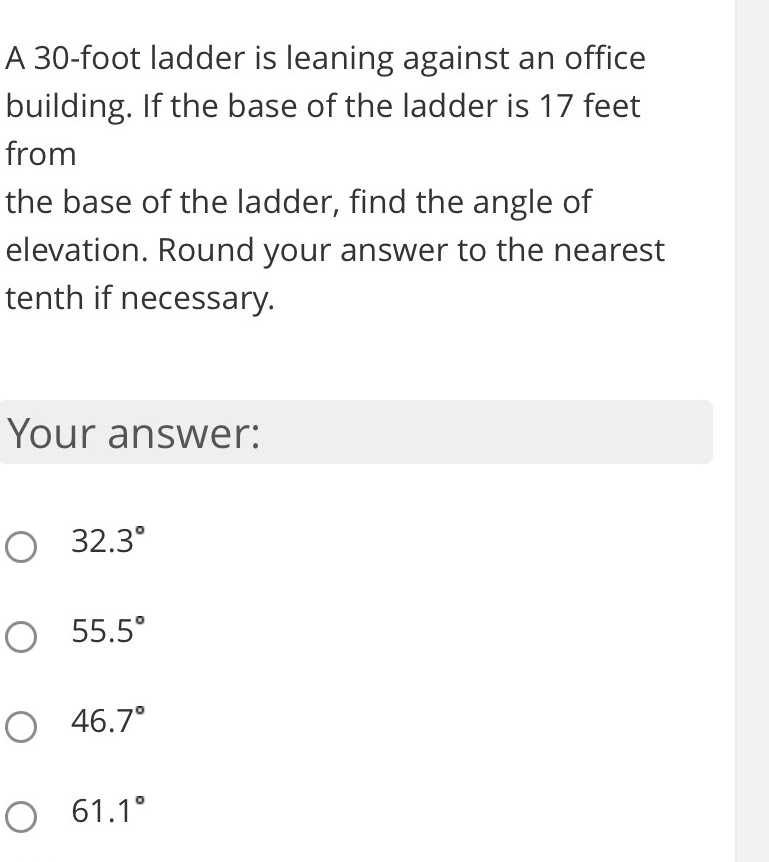$$30$$ -foot ladder is leaning against an office building. If the base of the ladder is $$17$$ feet from the base of the ladder, find the angle of elevation. Round your answer to the nearest tenth if necessary. Your answer:

$$32.3 ^ { \circ }$$

$$55.5 ^ { \circ }$$

$$46.7 ^ { \circ }$$

$$61.1 ^ { \circ }$$

Q: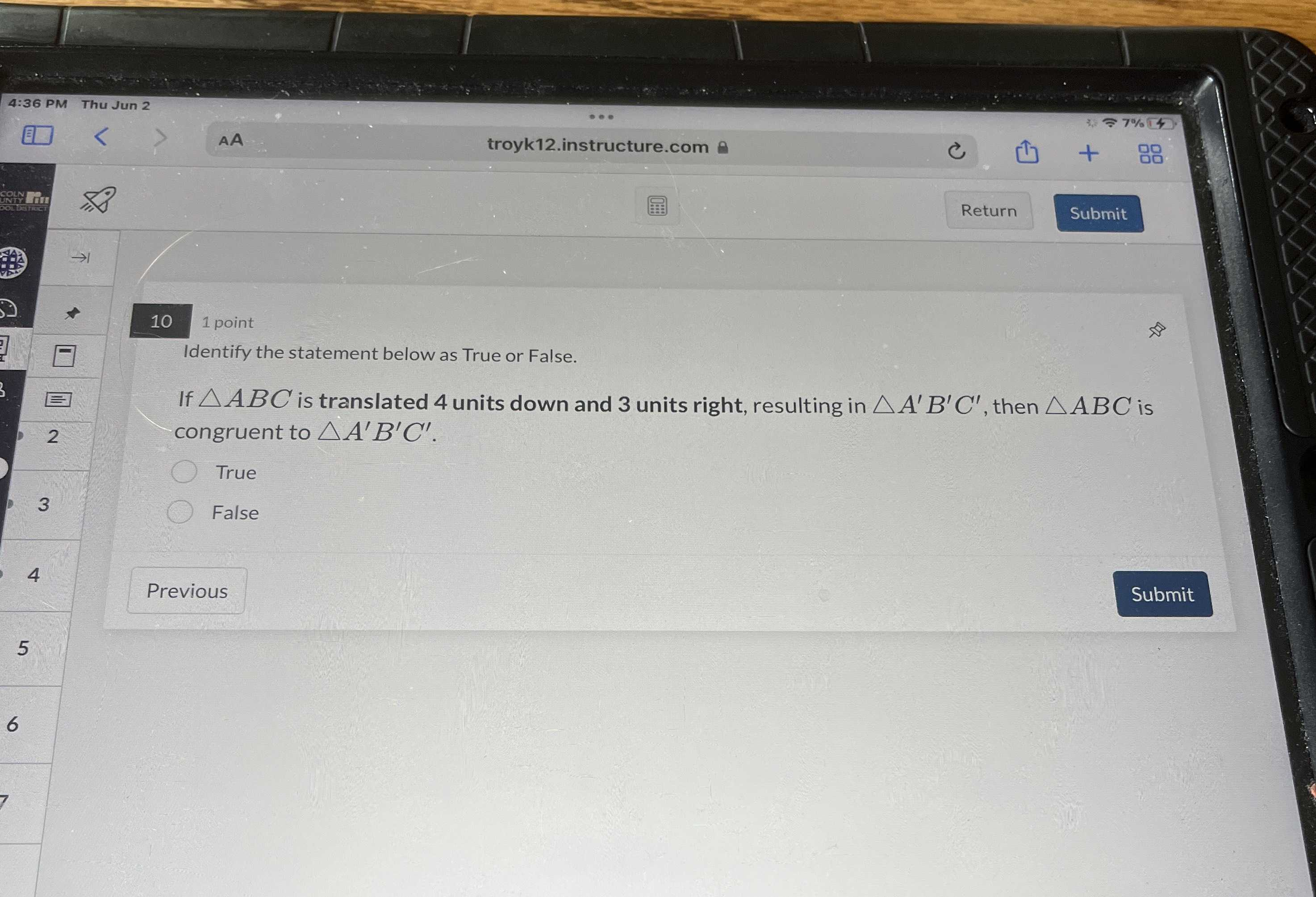Identify the statement below as True or False.

If $$\triangle A B C$$ is translated $$4$$ units down and $$3$$ units right, resulting in $$\triangle A ^ { \prime } B ^ { \prime } C ^ { \prime }$$ , then $$\triangle A B C$$ is congruent to $$\triangle A ^ { \prime } B ^ { \prime } C ^ { \prime }$$ .

Q: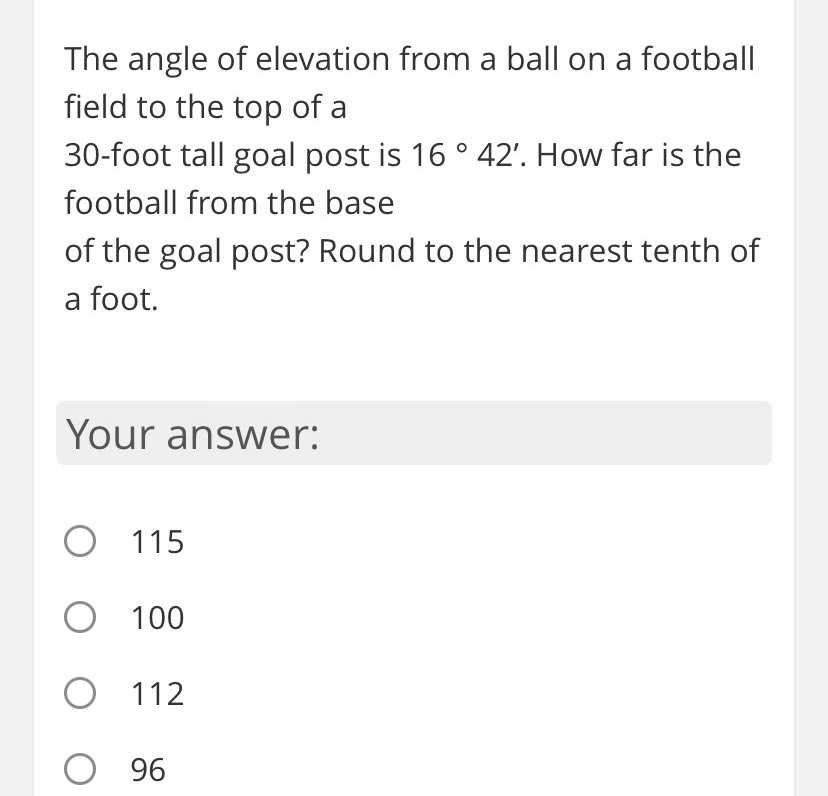The angle of elevation from a ball on a football field to the top of a $$30$$ -foot tall goal post is $$16 ^ { \circ } 42 ^ { \prime }$$ . How far is the football from the base of the goal post? Round to the nearest tenth of a foot. Your answer:

A. $$115$$

B. $$100$$

C. $$112$$

D. $$96$$

Q: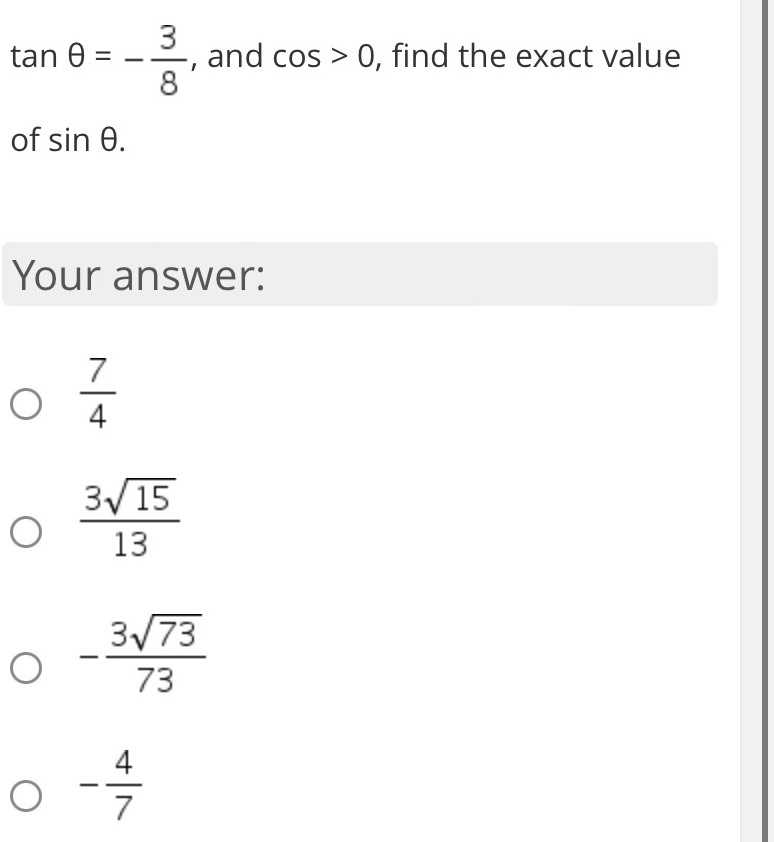$$\tan \theta = - \frac { 3 } { 8 } ,$$ and $$\cos > 0 ,$$ find the exact value of $$\sin \theta$$

$$\frac { 7 } { 4 }$$

$$\frac { 3 \sqrt { 15 } } { 13 }$$

$$- \frac { 3 \sqrt { 73 } } { 73 }$$

$$- \frac { 4 } { 7 }$$

Q: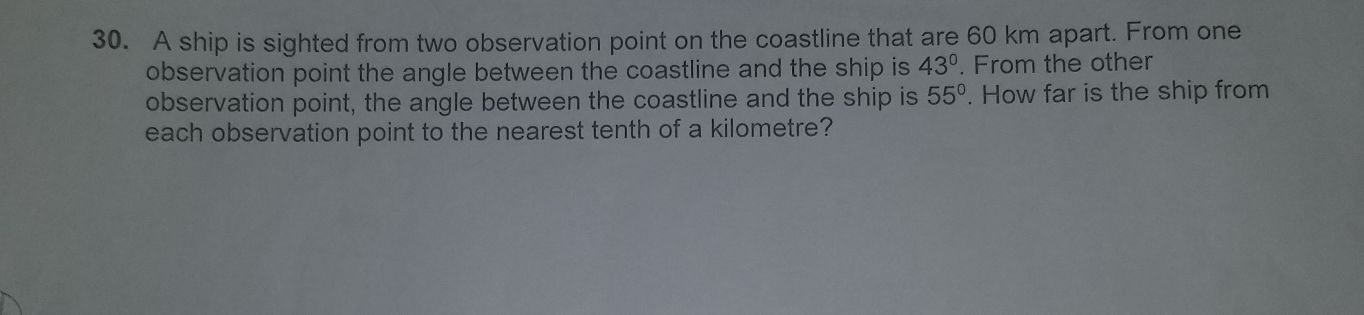A ship is sighted from two observation point on the coastline that are $$60 km$$ apart. From one observation point the angle between the coastline and the ship is $$43 ^ { \circ }$$ . From the other observation point, the angle between the coastline and the ship is $$55 ^ { \circ }$$ . How far is the ship from each observation point to the nearest tenth of a kilometre?

Q: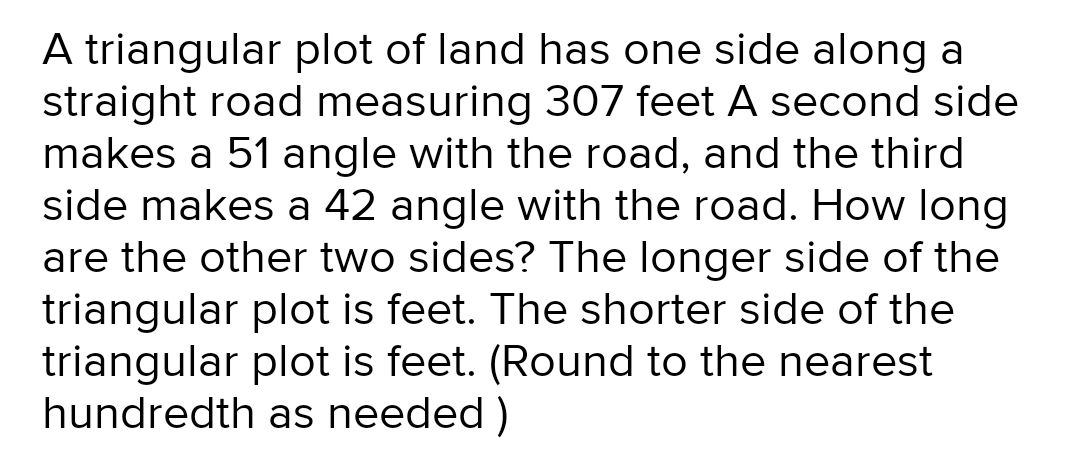A triangular plot of land has one side along a straight road measuring $$307$$ feet $$A$$ second side makes a $$51$$ angle with the road, and the third side makes a $$42$$ angle with the road. How long are the other two sides? The longer side of the triangular plot is feet. The shorter side of the triangular plot is feet. (Round to the nearest hundredth as needed)

Q: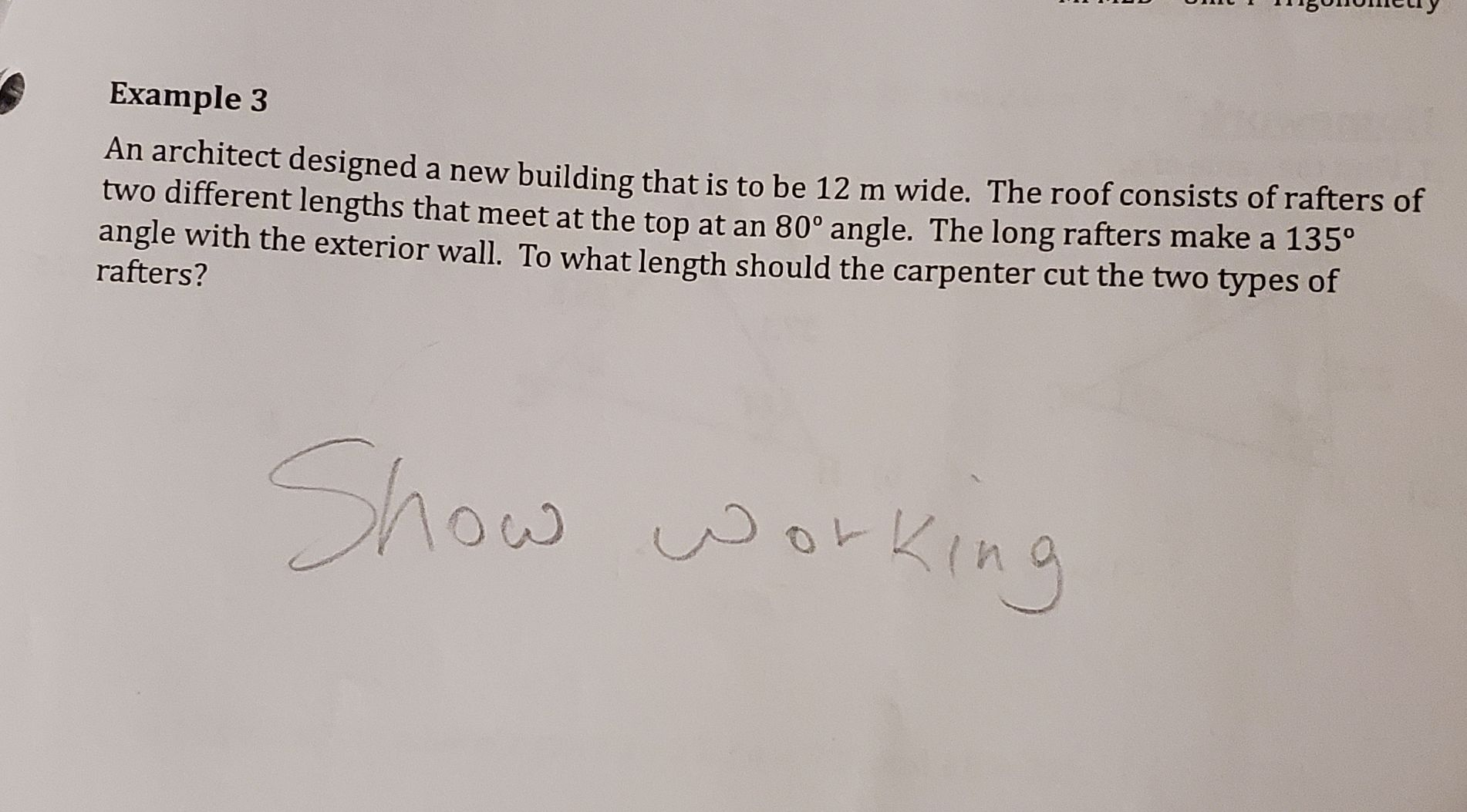An architect designed a new building that is to be $$12 m$$ wide. The roof consists of rafters of two different lengths that meet at the top at an $$80 ^ { \circ }$$ angle. The long rafters make a $$135 ^ { \circ }$$

angle with the exterior wall. To what length should the carpenter cut the two types of rafters?

Q: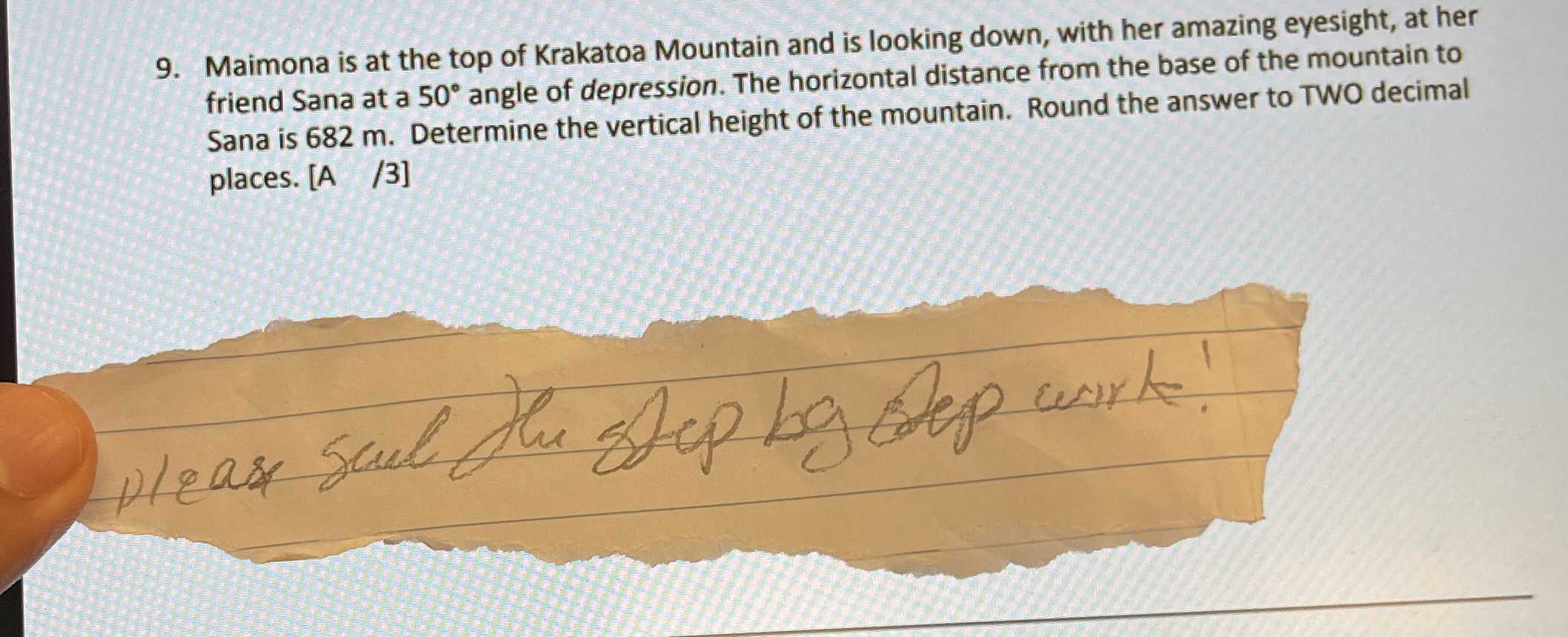Maimona is at the top of Krakatoa Mountain and is looking down, with her amazing eyesight, at her friend Sana at a $$50 ^ { \circ }$$ angle of depression. The horizontal distance from the base of the mountain to Sana is $$682 m$$ . Determine the vertical height of the mountain. Round the answer to TWO decimal places.

Q: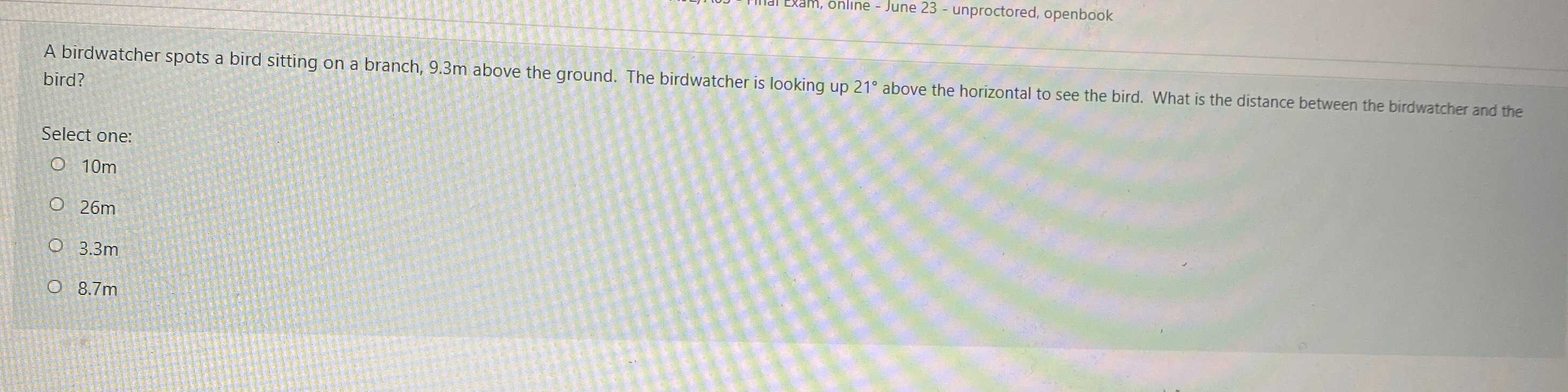A birdwatcher spots a bird sitting on a branch, $$9.3 m$$ above the ground. The birdwatcher is looking up $$21 ^ { \circ }$$ above the horizontal to see the bird. What is the distance between the birdwatcher and the bird?

Select one:

A. $$010 m$$

B. $$026 m$$

C. $$0.3 .3 m$$

D. $$08.7 m$$

Q: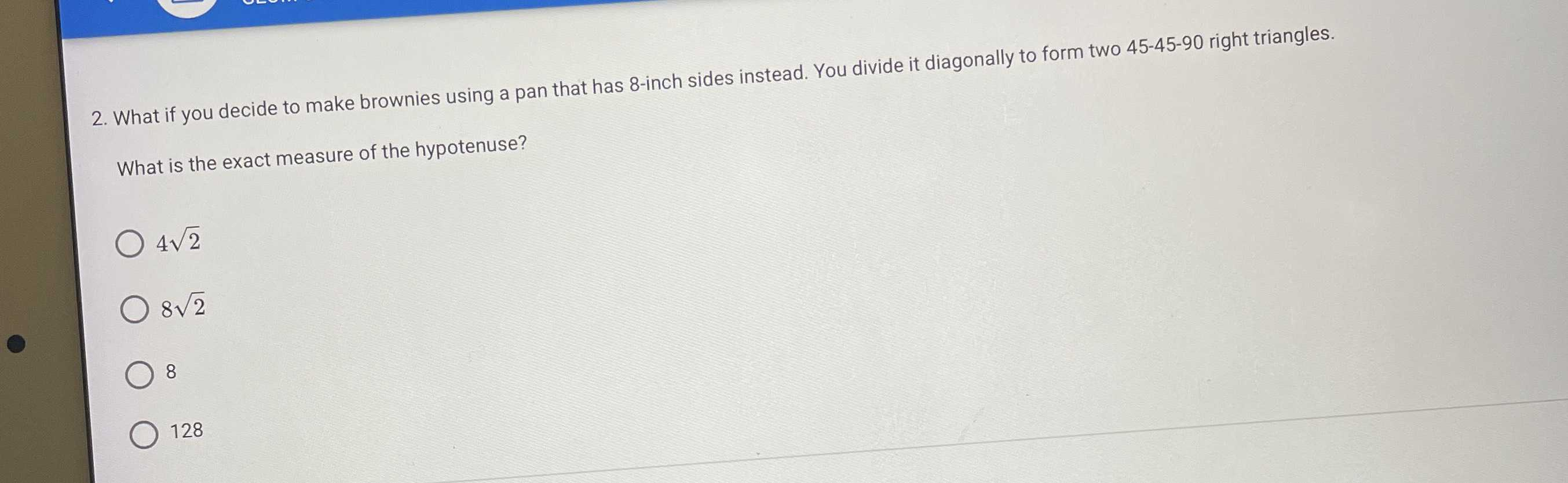What if you decide to make brownies using a pan that has $$8$$ -inch sides instead. You divide it diagonally to form two $$45 - 45 - 90$$ right triangles. What is the exact measure of the hypotenuse?

A. $$4 \sqrt { 2 }$$

B. $$8 \sqrt { 2 }$$

C. $$8$$

D. $$128$$

Q: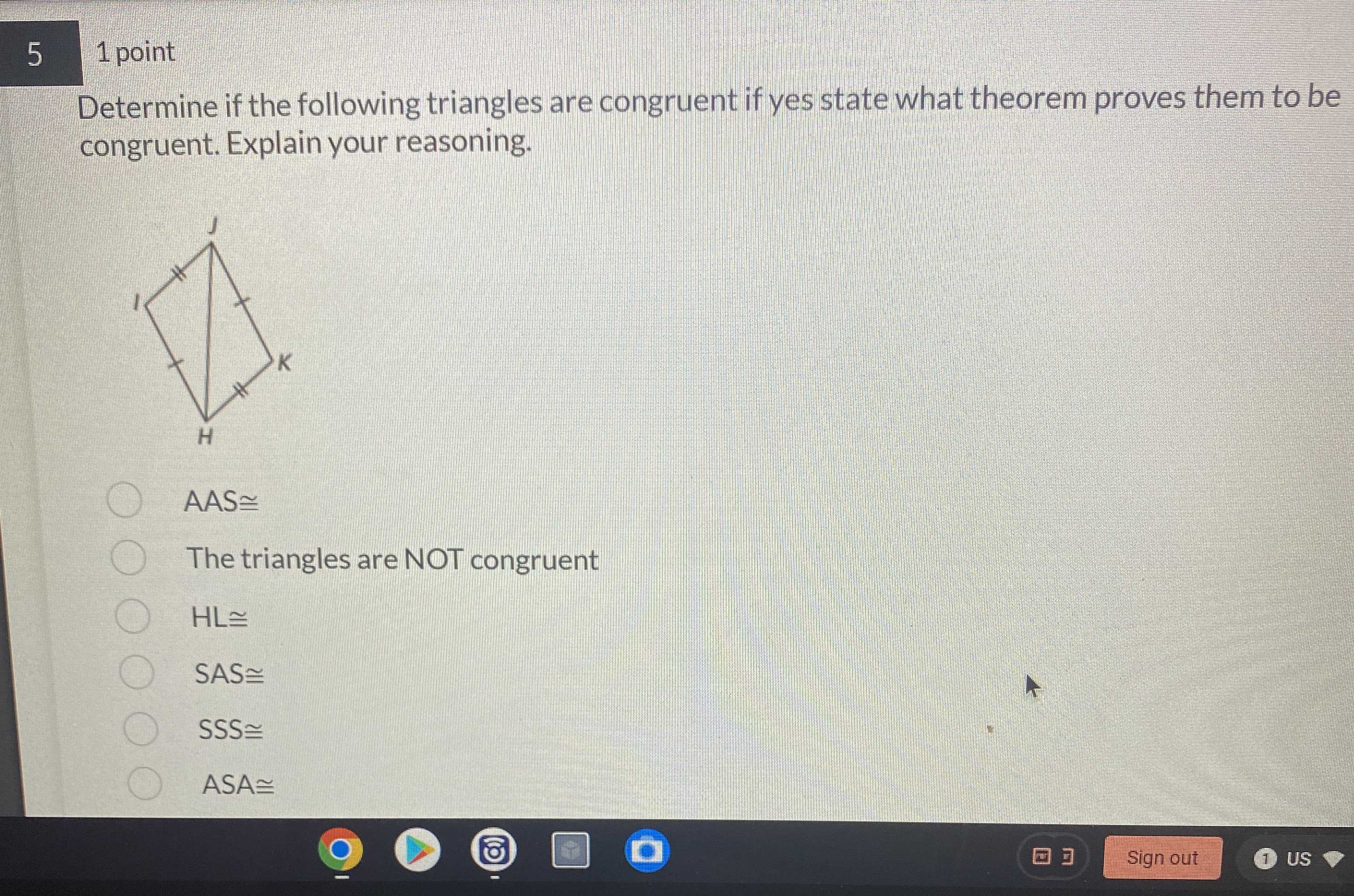Determine if the following triangles are congruent if yes state what theorem proves them to be congruent. Explain your reasoning.

A. $$AAS \cong$$

B. The triangles are NOT congruent

C. $$HL \cong$$

D. $$SAS \cong$$

E. $$SSS \cong$$

F. $$ASA \cong$$

Q: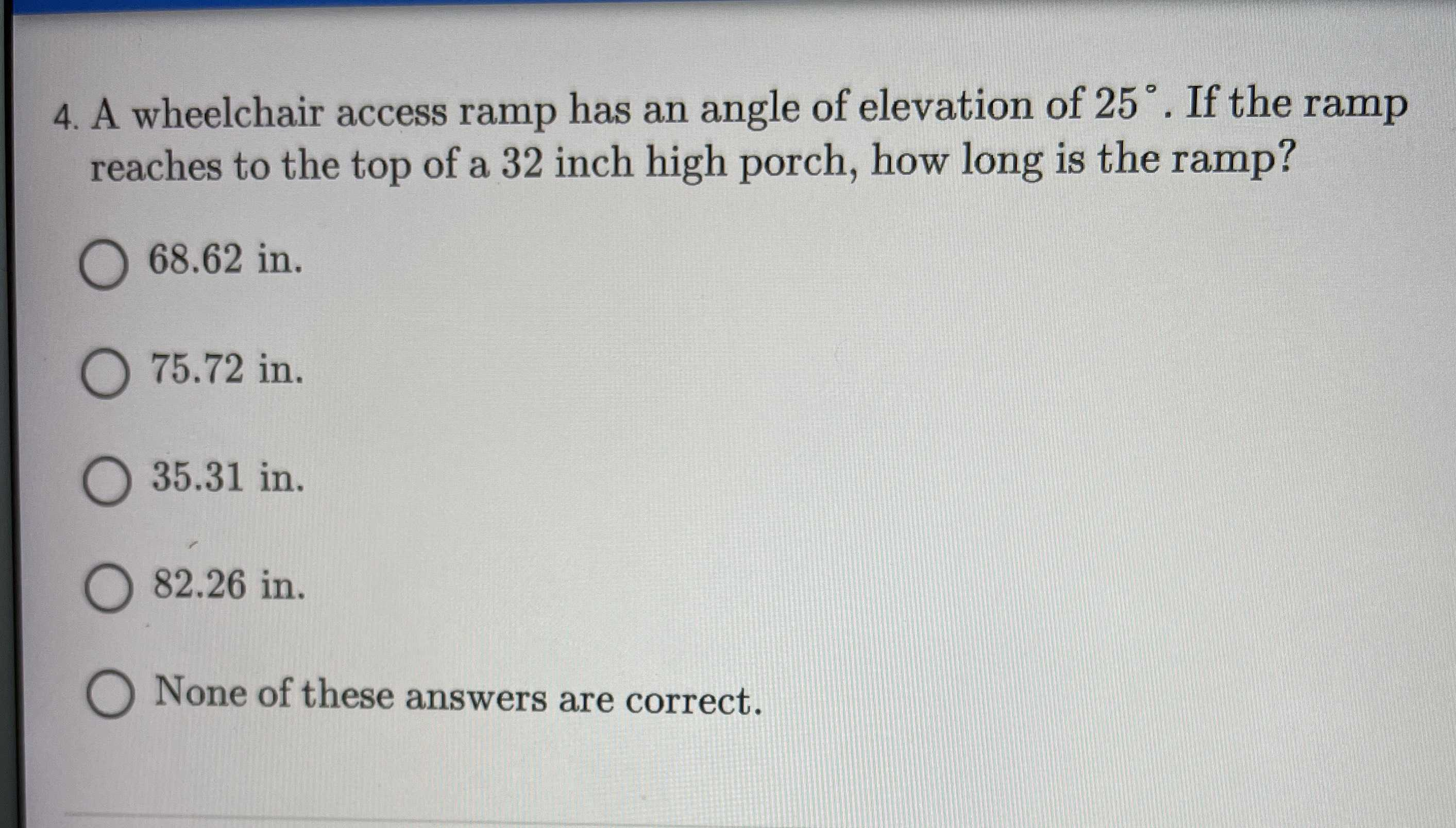A wheelchair access ramp has an angle of elevation of $$25 ^ { \circ }$$ . If the ramp reaches to the top of a $$32$$ inch high porch, how long is the ramp?

$$68.62 in .$$

$$75.72 in.$$

$$35.31 in.$$

$$82.26 in$$ . None of these answers are correct.

Q: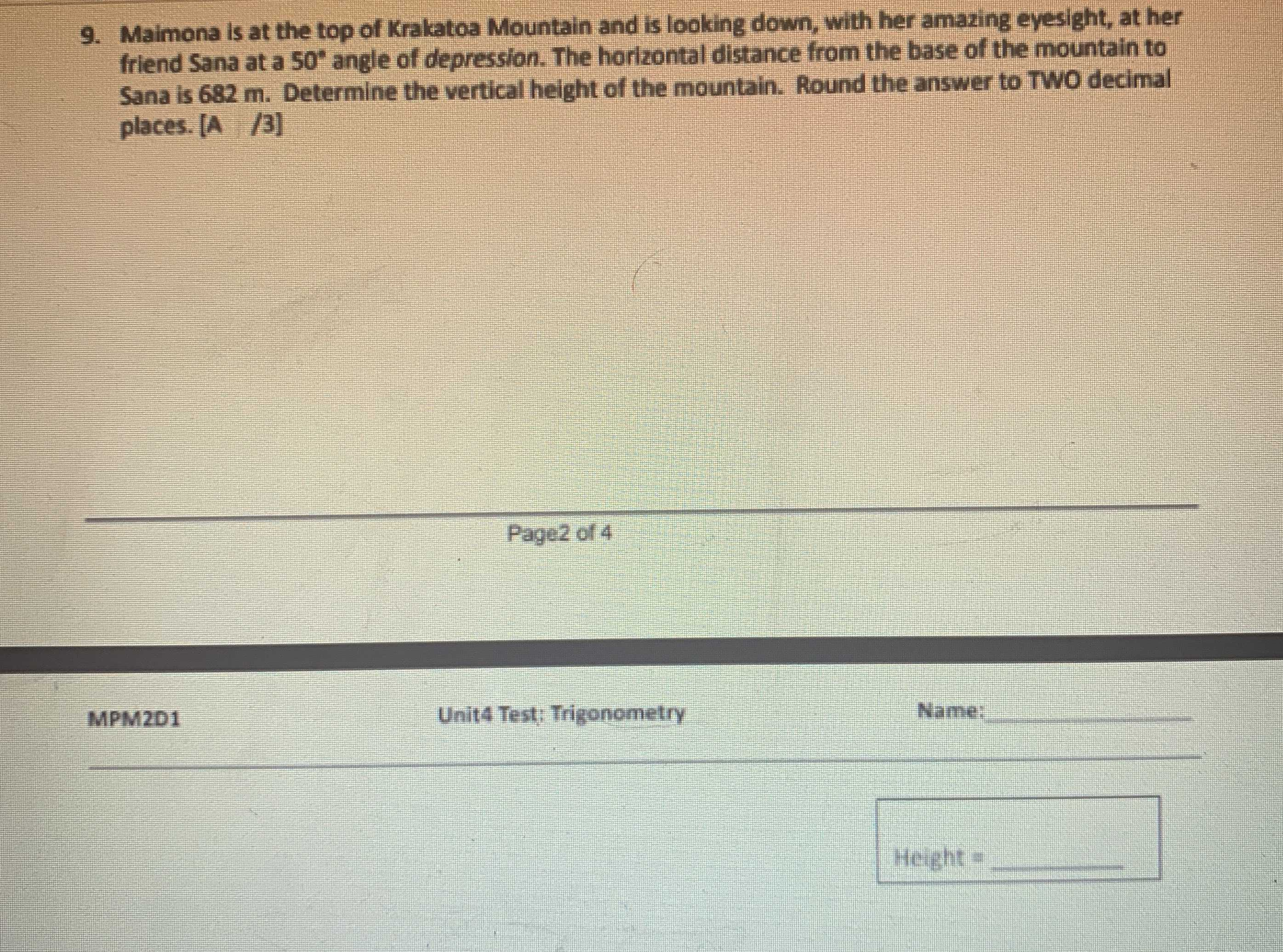Maimona is at the top of Kralatoa Mountain and is looking down, with her amazing eyesight, at her friend Sana at a $$50 ^ { \circ }$$ angle of depression. The horizontal distance from the base of the mountain to Sana is $$682 m$$ . Determine the vertical height of the mountain. Round the answer to TOO decimal places. $$[ A / 3 ]$$

Q: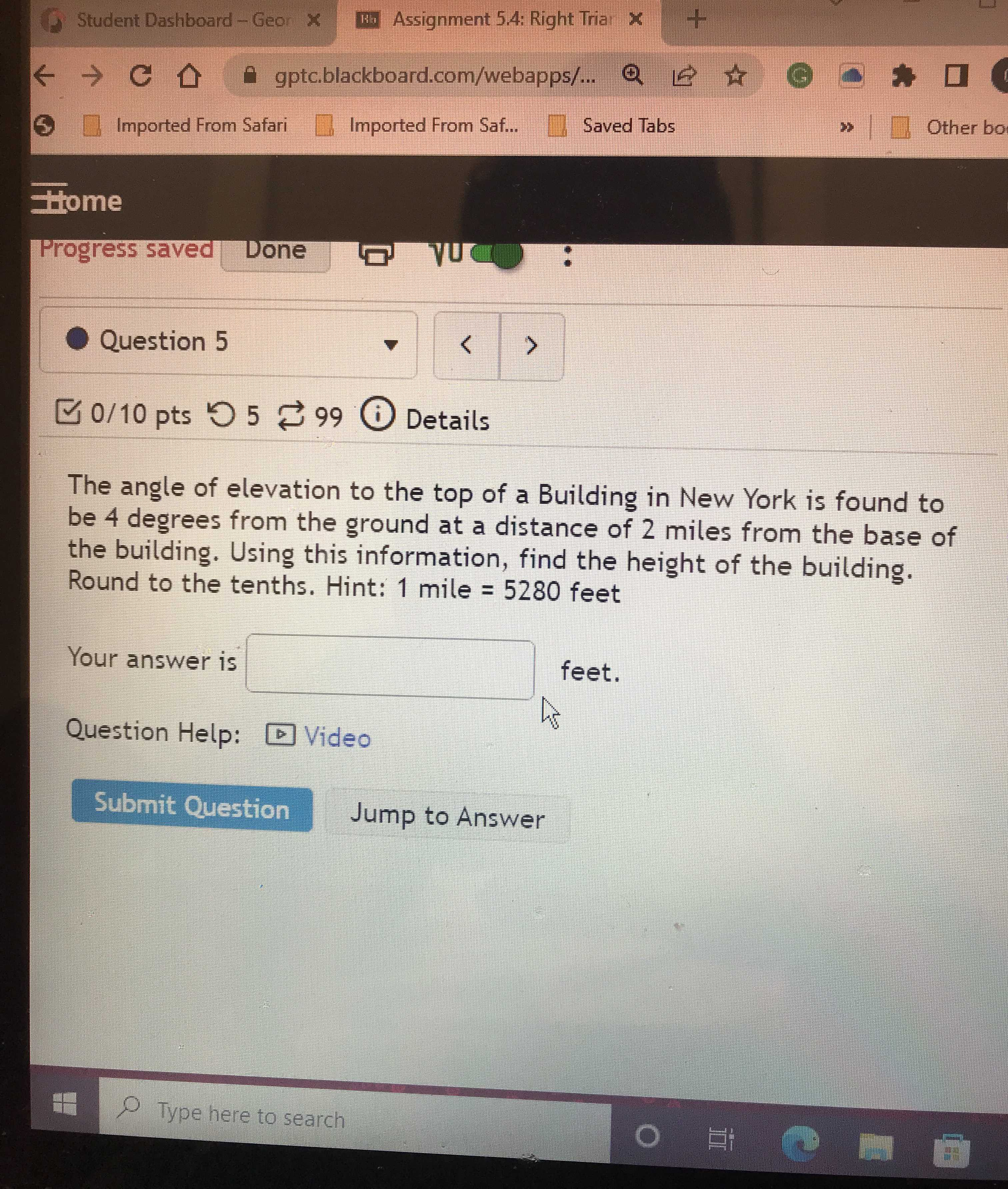The angle of elevation to the top of a Building in New York is found to be $$4$$ degrees from the ground at a distance of $$2$$ miles from the base of the building. Using this information, find the height of the building. Round to the tenths. Hint: $$1$$ mile $$= 5280$$ feet

Q: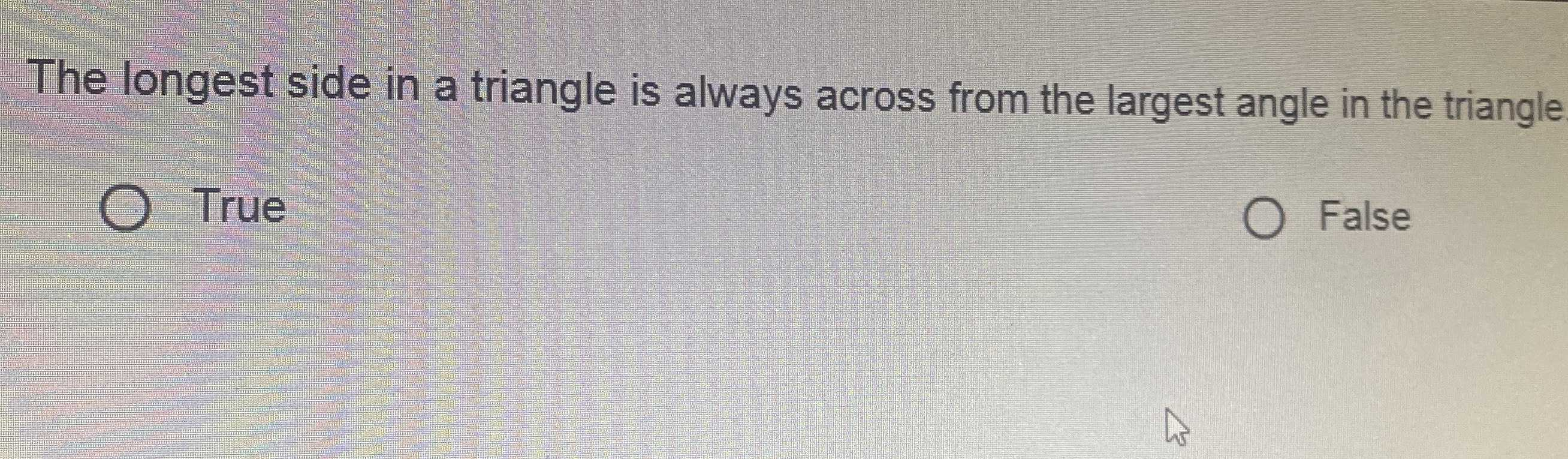The longest side in a triangle is always across from the largest angle in the triangle True

Judge whether the statement is True or False?

Q: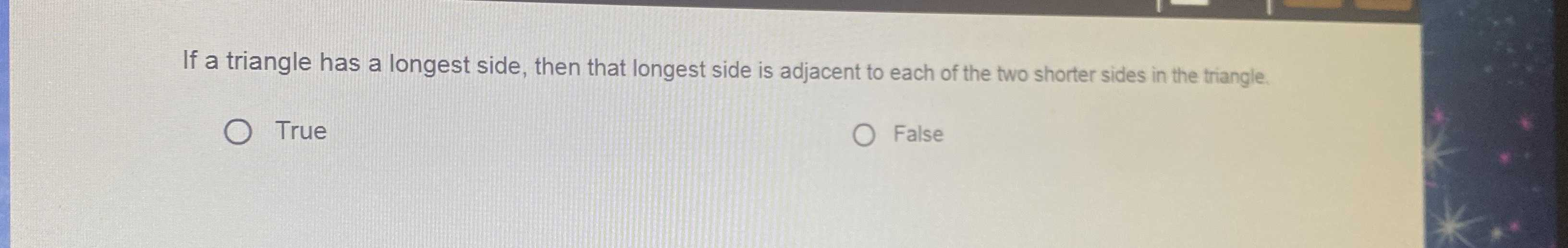If a triangle has a longest side, then that longest side is adjacent to each of the two shorter sides in the triangle.

Judge whether the statement is True or False.

Q: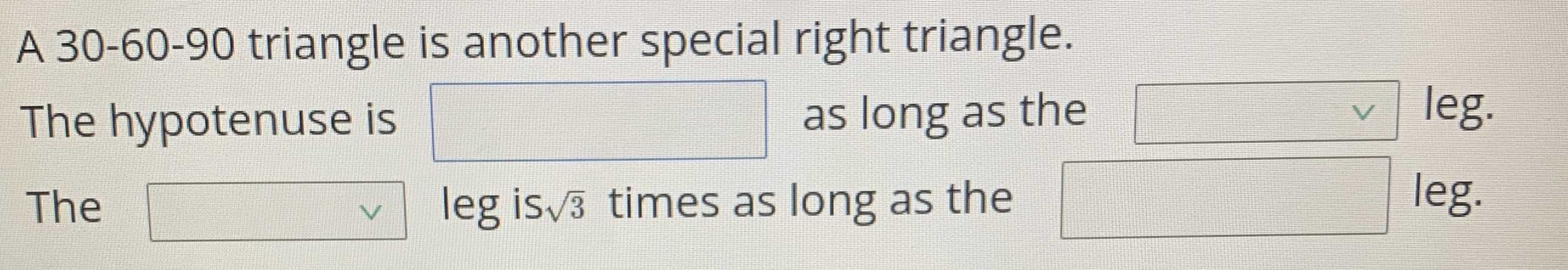$$30 - 60 - 90$$ triangle is another special right triangle. The hypotenuse is $$\square$$ as long as the $$\square$$ leg. The $$\square$$ leg is $$\sqrt { 3 }$$ times as long as the $$\square$$

Q:

The perimeter of an equilateral triangle is 39 centimeters. Find the length of the altitude of the triangle.

Q: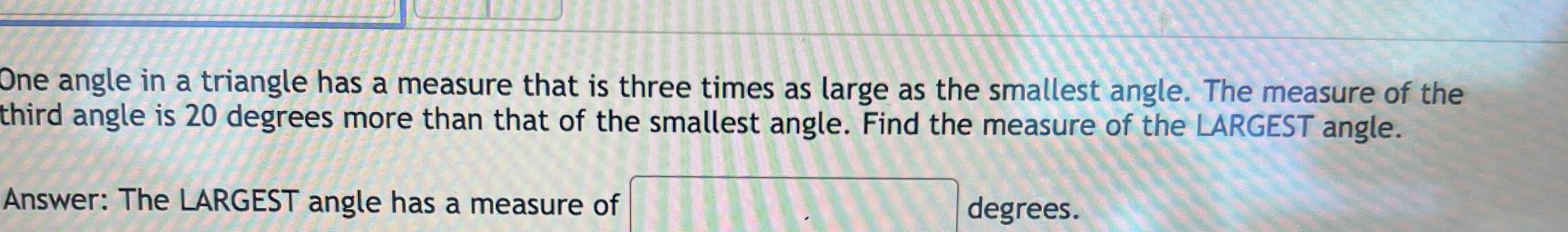One angle in a triangle has a measure that is three times as large as the smallest angle. The measure of the third angle is $$20$$ degrees more than that of the smallest angle. Find the measure of the LARGEST angle.

Q: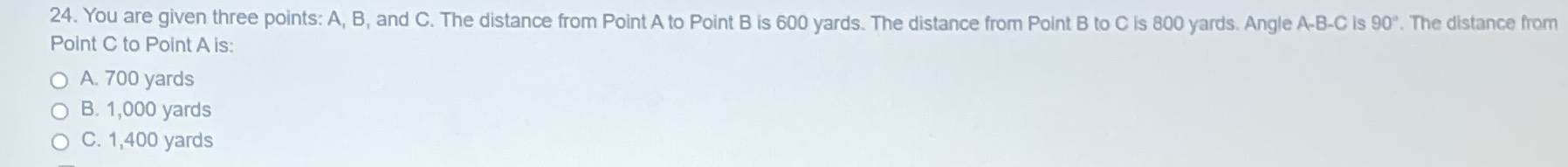You are given three points: A, B, and C. The distance from Point $$A$$ to Point $$B$$ is $$600$$ yards. The distance from Point $$B$$ to C is $$800$$ yards. Angle A-B-C is $$90 ^ { \circ }$$ . The distance from Point $$C$$ to Point $$A$$ is:

A. $$700$$ yards

B. $$1,000$$ yards

C. $$1,400$$ yards

Q: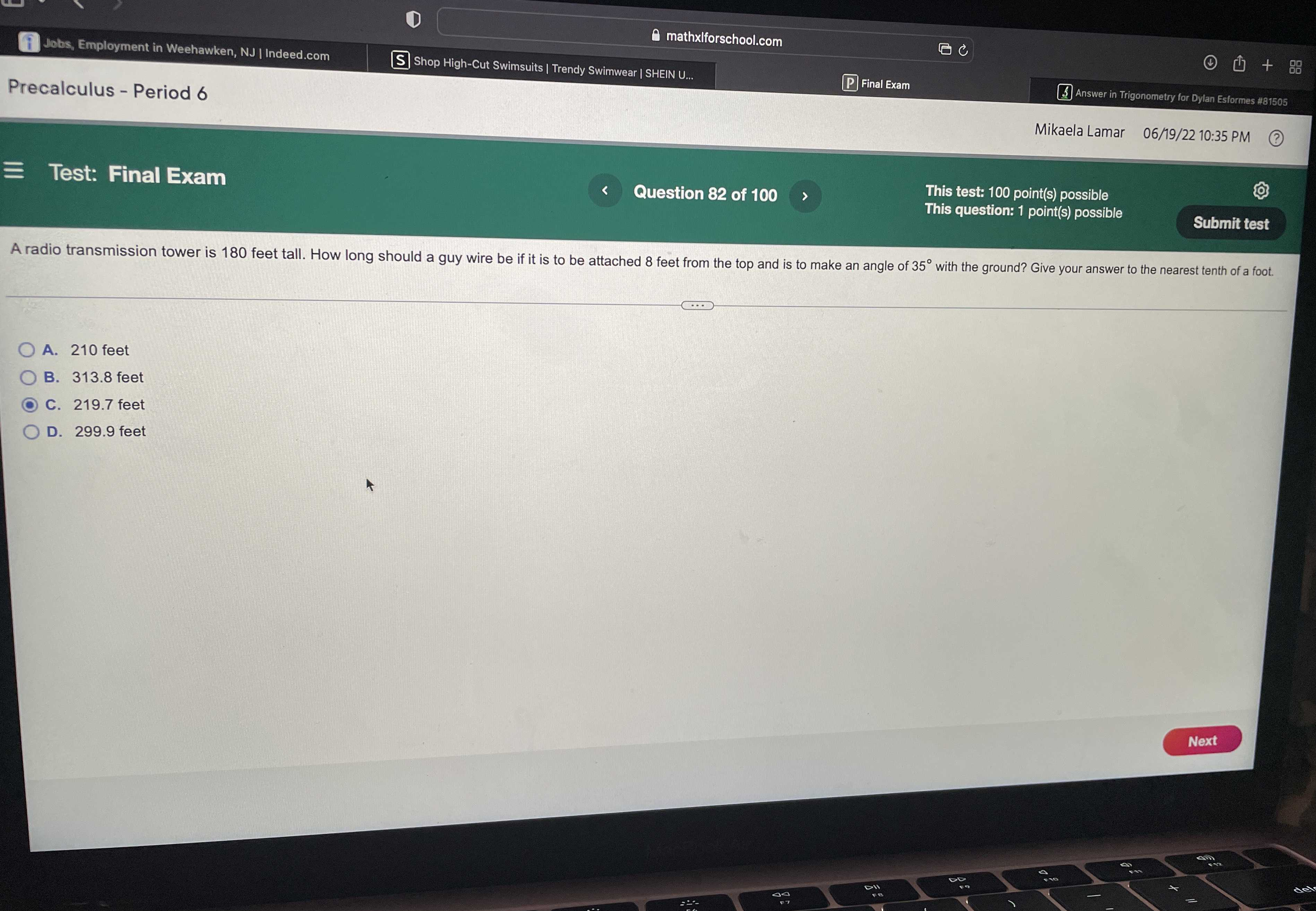A radio transmission tower is 180 feet tall. How long should a guy wire be if it is to be attached 8 feet from the top and is to make an angle of 35 degrees with the ground? Give your answer to the nearest tenth of a foot.

A. $$210$$ feet

B. $$313.8$$ feet

C. $$219.7$$ feet

D. $$299.9$$ feet

Q: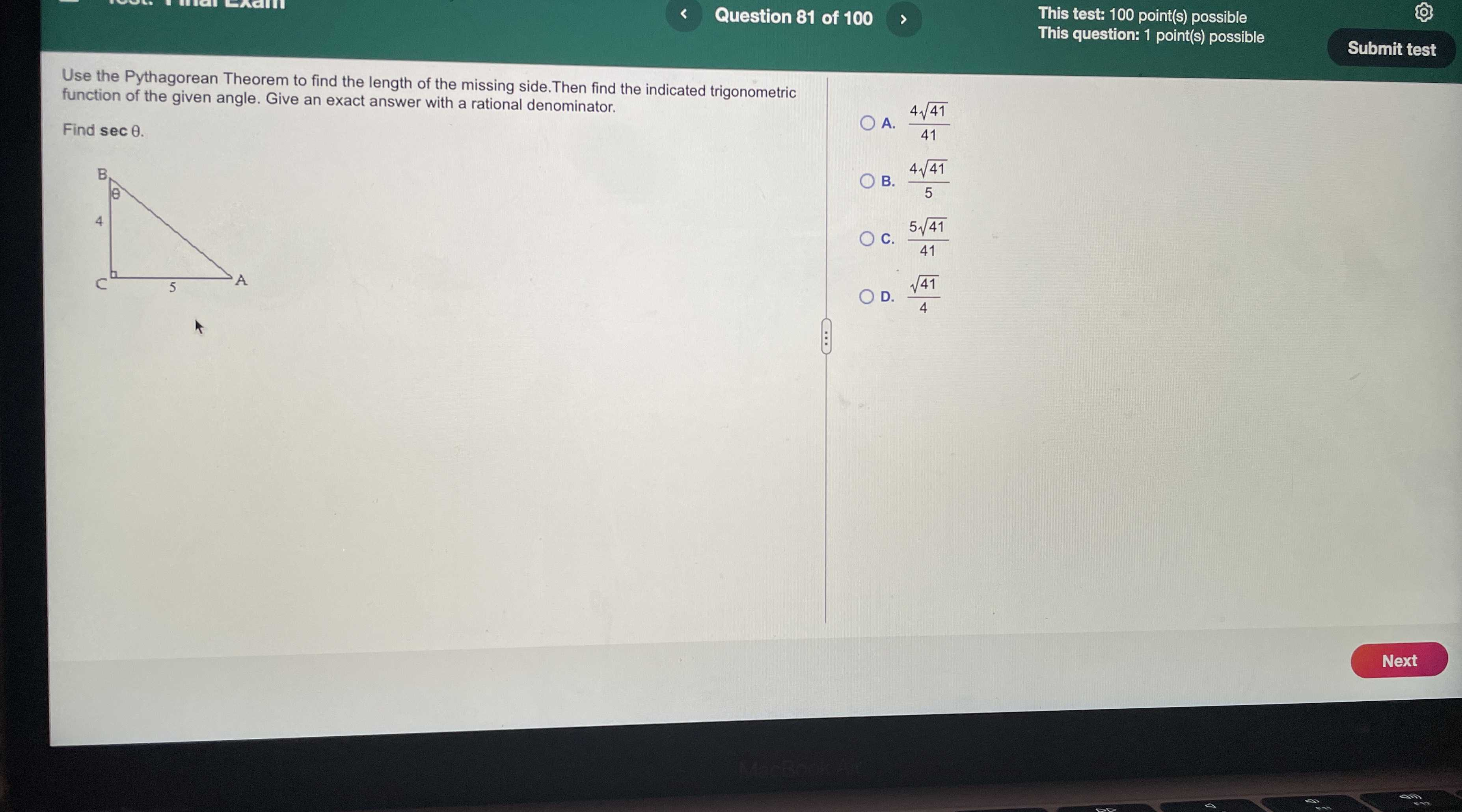Use the Pythagorean Theorem to find the length of the missing side. Then find the indicated trigonometric function of the given angle. Give an exact answer with a rational denominator. Find $$\sec \theta$$ .

A. $$\frac { 4 \sqrt { 41 } } { 41 }$$

B. $$\frac { 4 \sqrt { 41 } } { 5 }$$

C. $$\frac { 5 \sqrt { 41 } } { 41 }$$

D. $$\frac { \sqrt { 41 } } { 4 }$$

Q: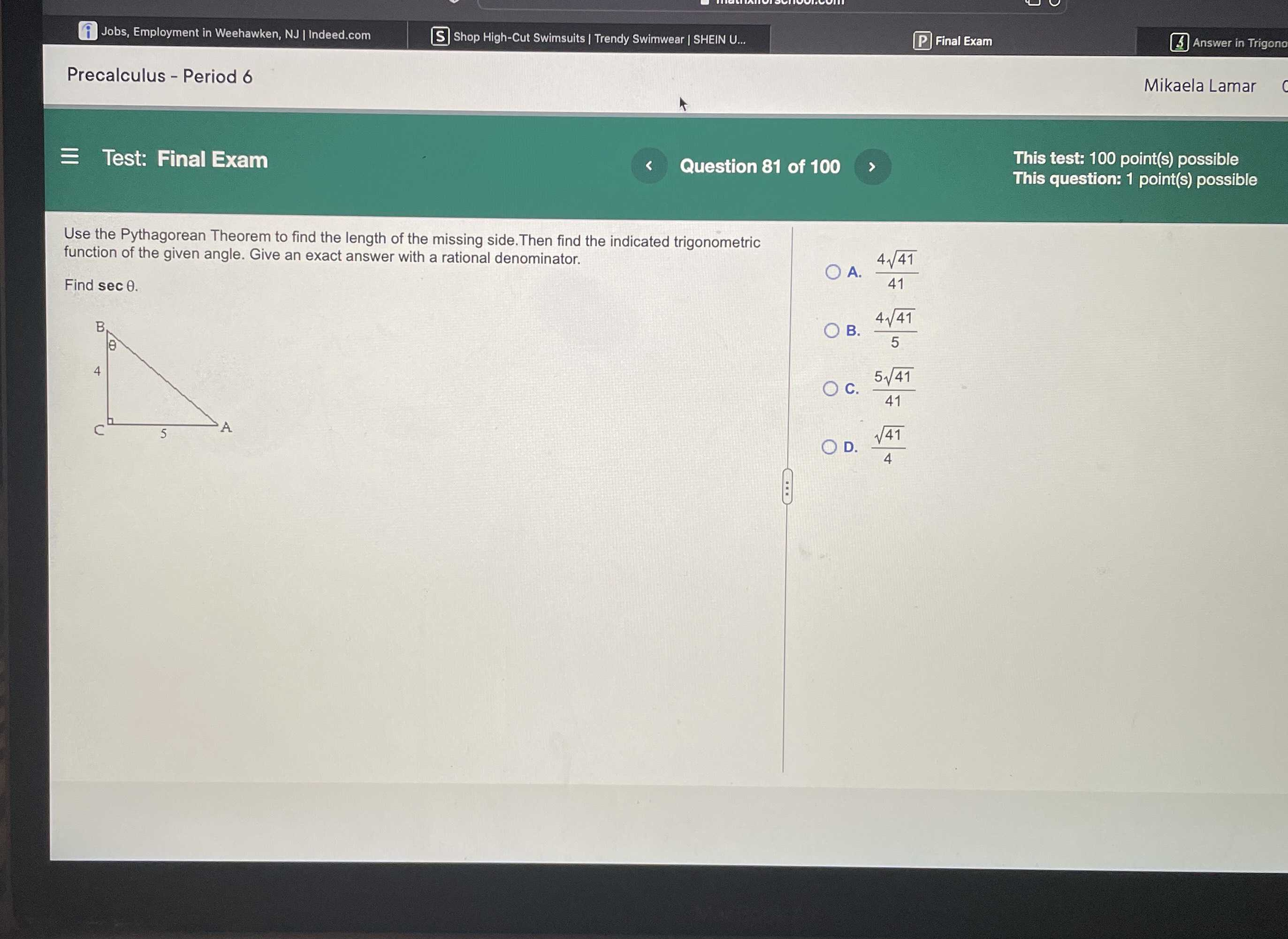Use the Pythagorean Theorem to find the length of the missing side. Then find the indicated trigonometric function of the given angle. Give an exact answer with a rational denominator. Find $$\sec \theta$$ .

A. $$\frac { 4 \sqrt { 41 } } { 41 }$$

B. $$\frac { 4 \sqrt { 41 } } { 5 }$$

C. $$\frac { 5 \sqrt { 41 } } { 41 }$$

D. $$\frac { \sqrt { 41 } } { 4 }$$

Q: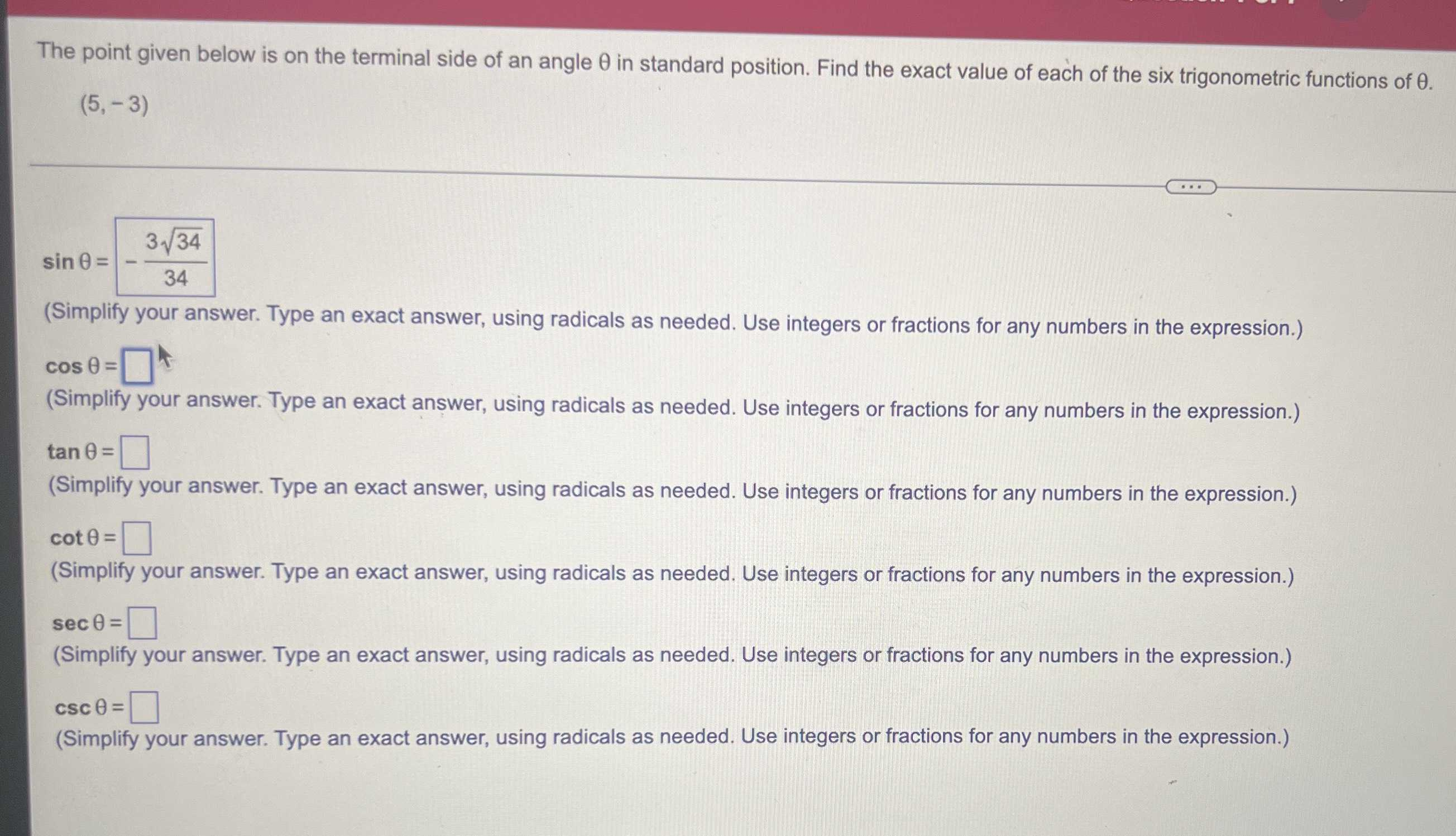The point given below is on the terminal side of an angle $$\theta$$ in standard position. Find the exact value of each of the six trigonometric functions of $$\theta$$ .

$$( 5 , - 3 )$$

$$\sin \theta =$$_______                       $$\cos \theta =$$ _______

$$\tan \theta =$$_______                      $$\cot \theta =$$_______

$$\sec \theta =$$_______                       $$\csc \theta =$$_______

Q: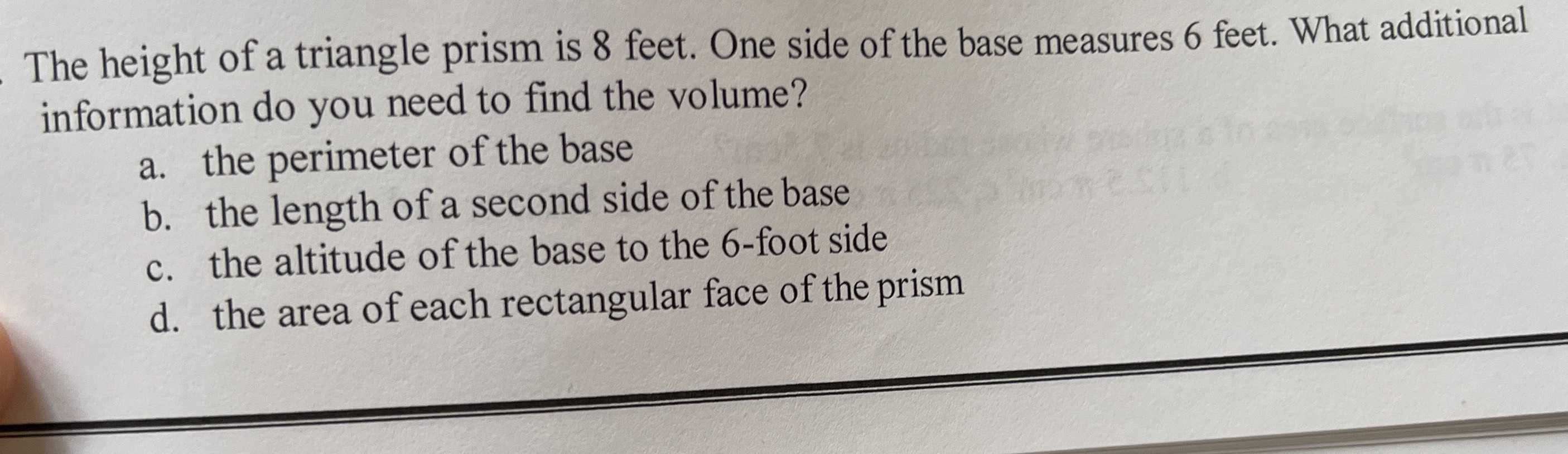The height of a triangle prism is $$8$$ feet. One side of the base measures $$6$$ feet. What additional information do you need to find the volume?

a. the perimeter of the base

b. the length of a second side of the base

c. the altitude of the base to the $$6$$ -foot side

d. the area of each rectangular face of the prism

Q: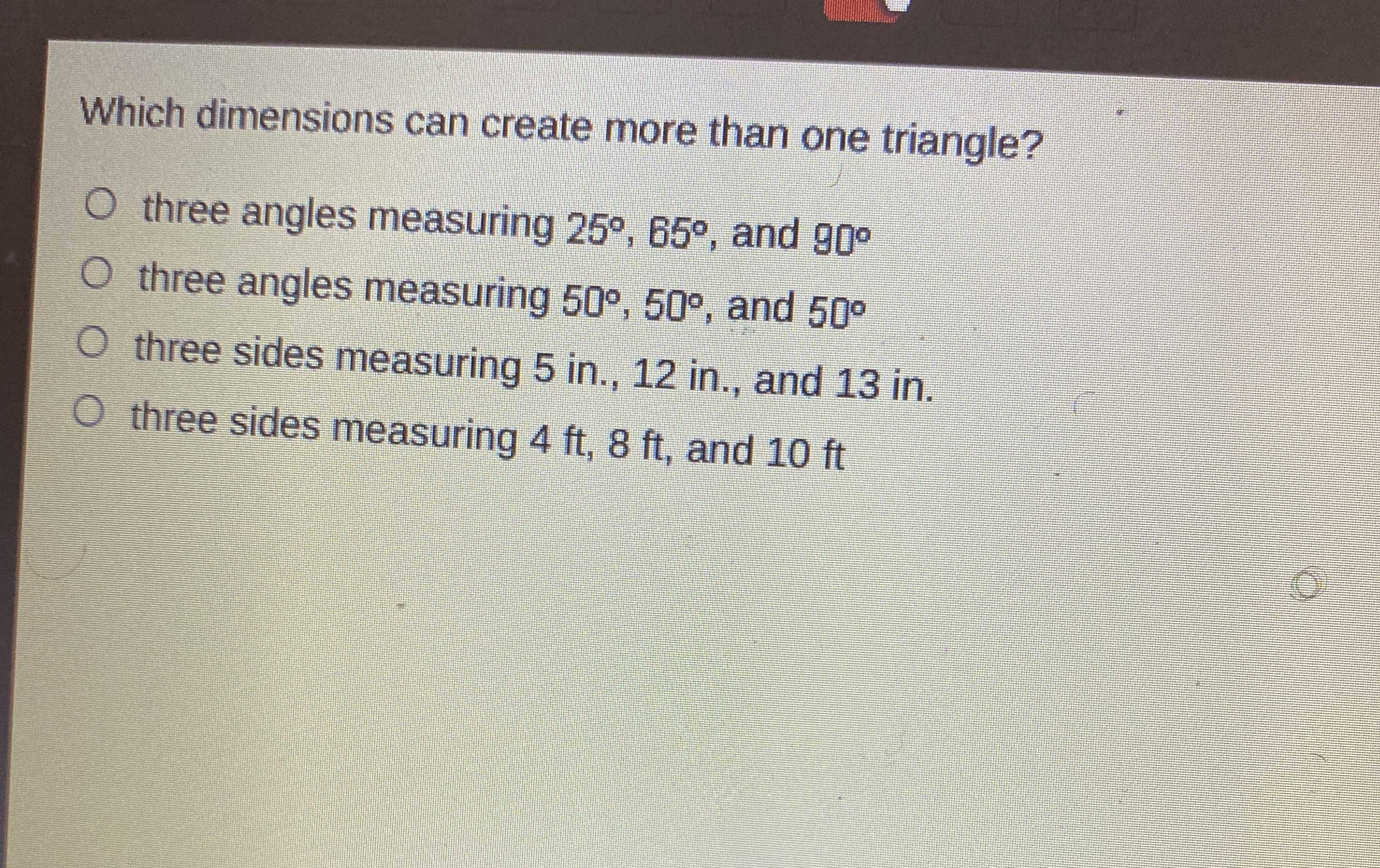Which dimensions can create more than one triangle?

A. three angles measuring $$25 ^ { \circ } , 65 ^ { \circ }$$ , and $$90 ^ { \circ }$$

B. three angles measuring $$50 ^ { \circ } , 50 ^ { \circ }$$ , and $$50 ^ { \circ }$$

C. three sides measuring $$5$$ in., $$12$$ in., and $$13$$ in.

D. three sides measuring $$4 ft , 8 ft$$ , and $$10 ft$$

Q: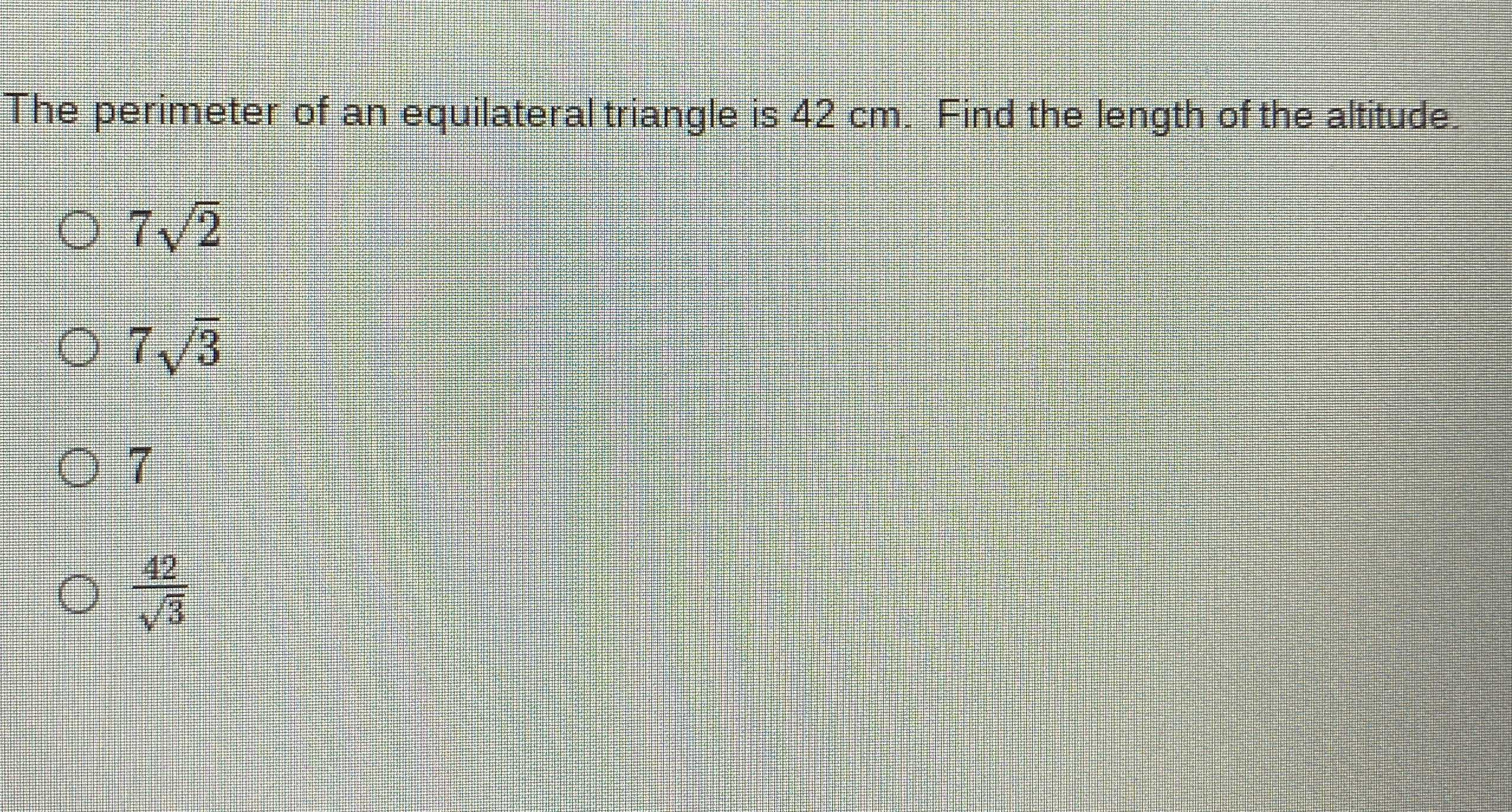The perimeter of an equilateral triangle is $$42 cm$$ . Find the length of the altitude.

A. $$7 \sqrt { 2 }$$

B. $$7 \sqrt { 3 }$$

C. $$7$$

D, $$\frac { 42 } { \sqrt { 3 } }$$

Q: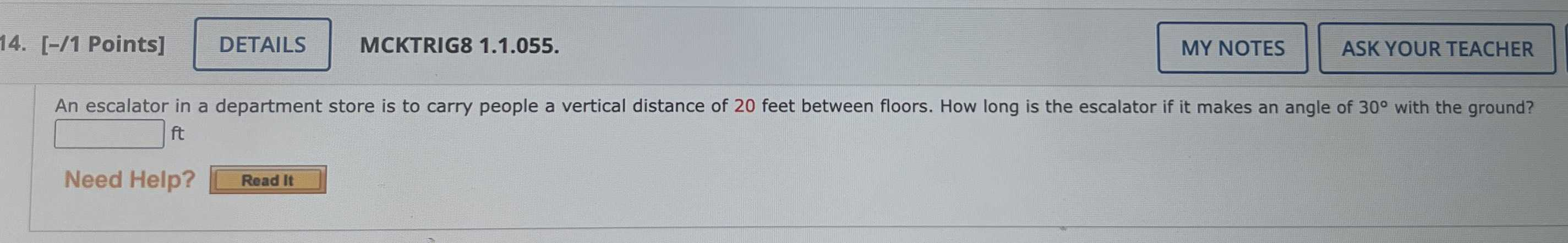An escalator in a department store is to carry people a vertical distance of $$20$$ feet between floors. How long is the escalator if it makes an angle of $$30 ^ { \circ }$$ with the ground?

Q: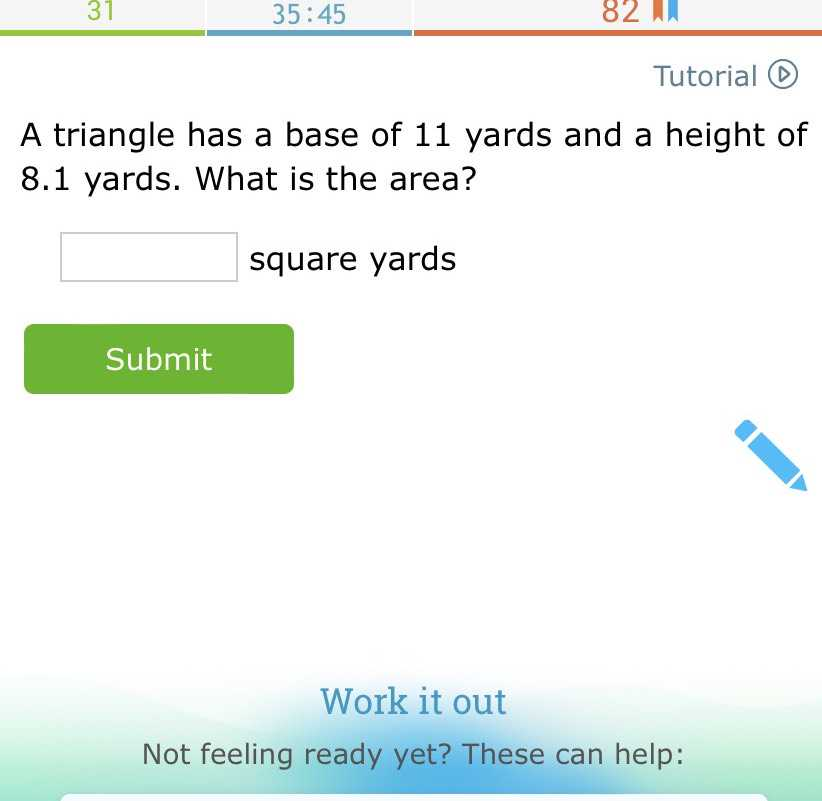A triangle has a base of $$11$$ yards and a height of $$8.1$$ yards. What is the area?

Q: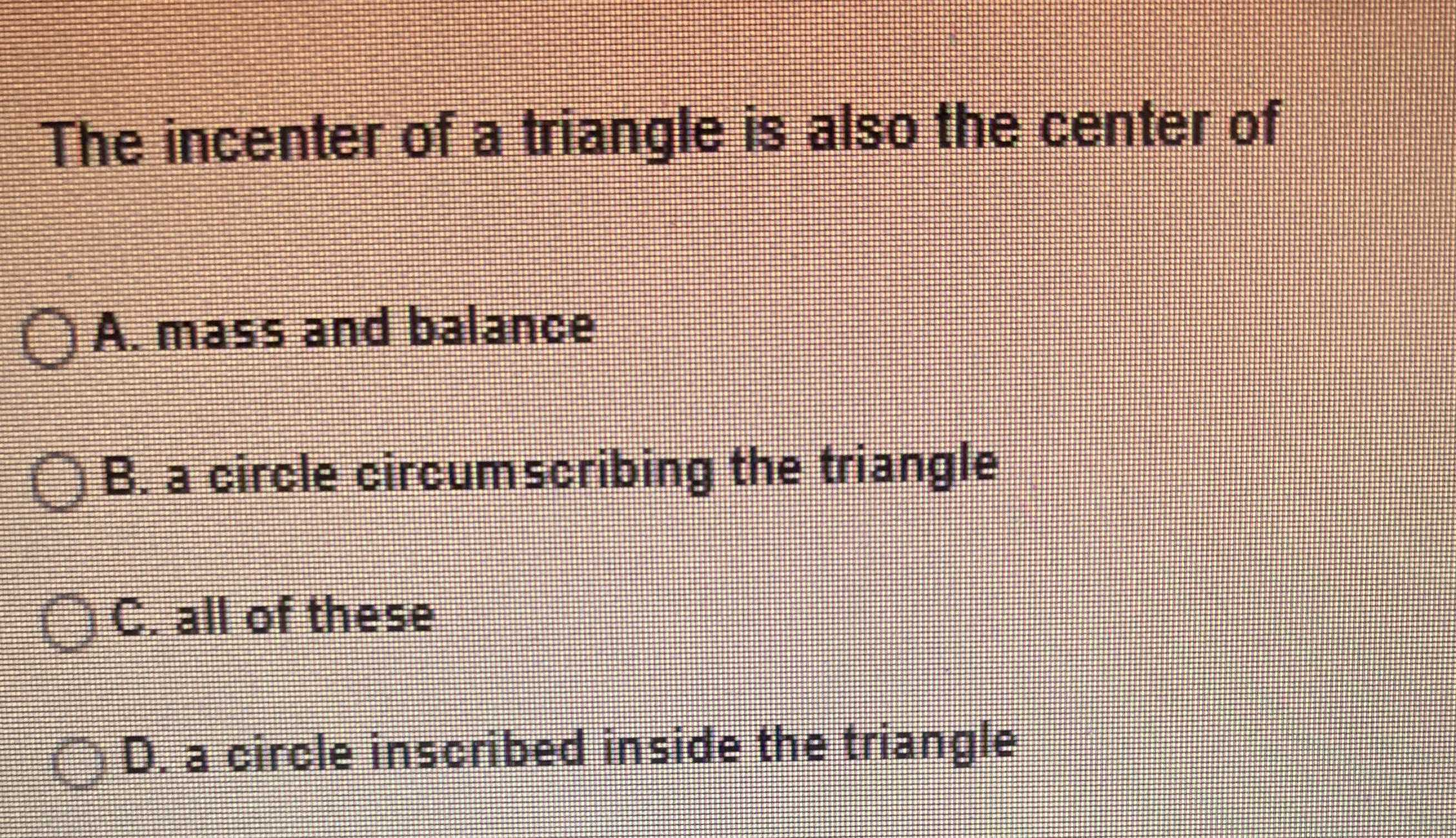The incenter of a tiangle is also the center of

A. mass and balance

B. a circle circumscribing the triangle

C. all of these

D. a circle inscribed inside the triangle

Q: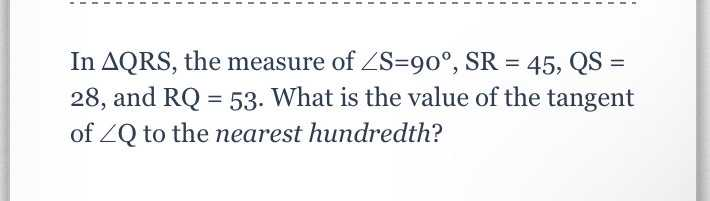In $$\triangle QRS$$ , the measure of $$\angle S = 90 ^ { \circ } , SR = 45 , QS =$$ $$28 ,$$ and $$RQ = 53 .$$ What is the value of the tangent of $$\angle Q$$ to the nearest hundredth?

Q: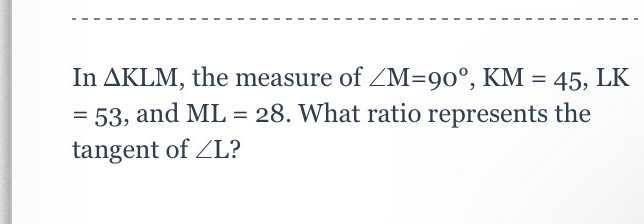In $$\Delta KLM$$ , the measure of $$\angle M = 90 ^ { \circ } , KM = 45 , LK$$ $$= 53 ,$$ and $$ML = 28$$ . What ratio represents the tangent of $$\angle L$$ ?

Q: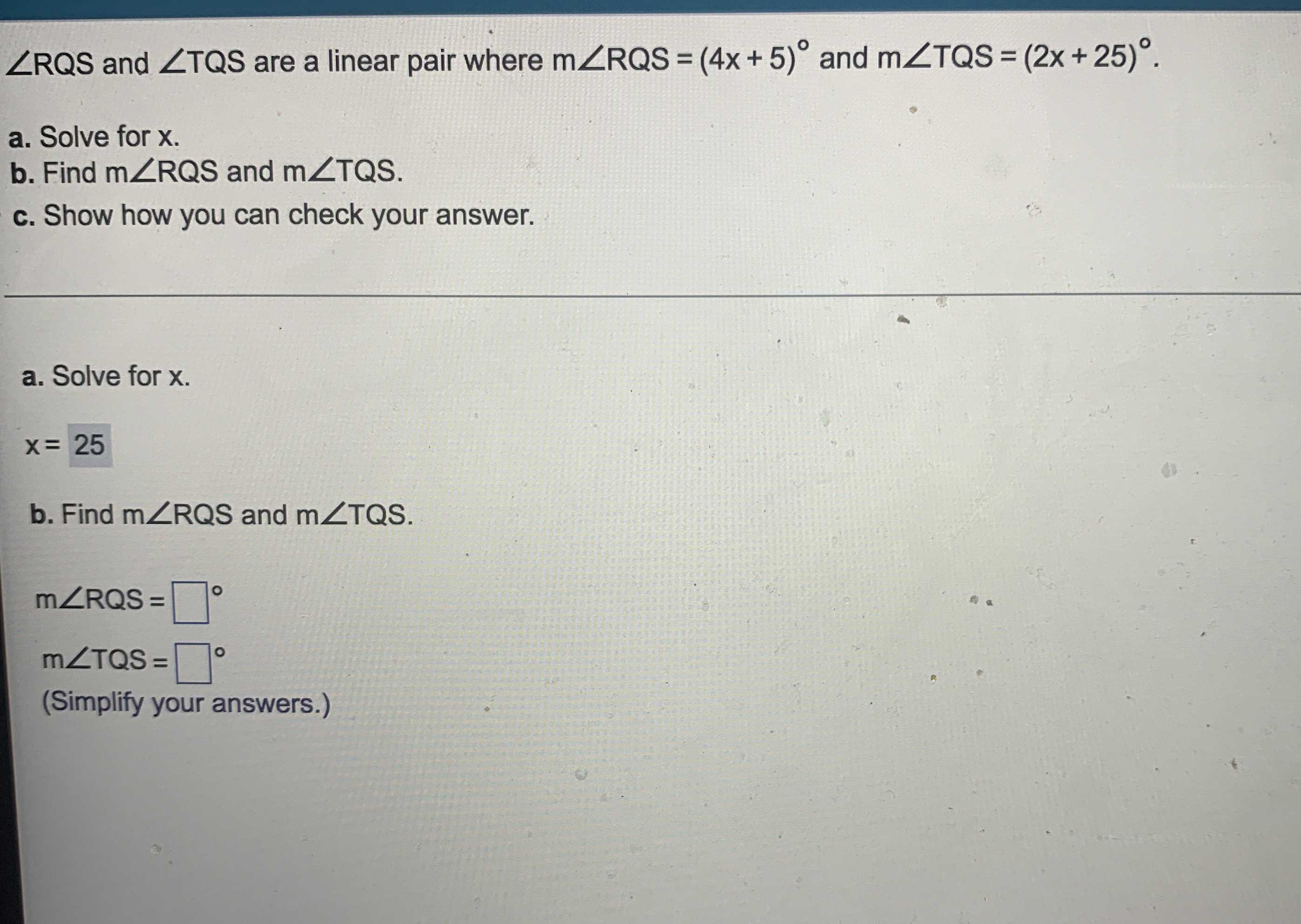$$\angle R Q S$$ and $$\angle T Q S$$ are a linear pair where $$m \angle R Q S = ( 4 x + 5 ) ^ { \circ }$$ and $$m \angle T Q S = ( 2 x + 25 ) ^ { \circ }$$ .

a. Solve for $$x$$ .

b. Find $$m \angle R Q S$$ and $$m \angle T Q S$$ .

Q: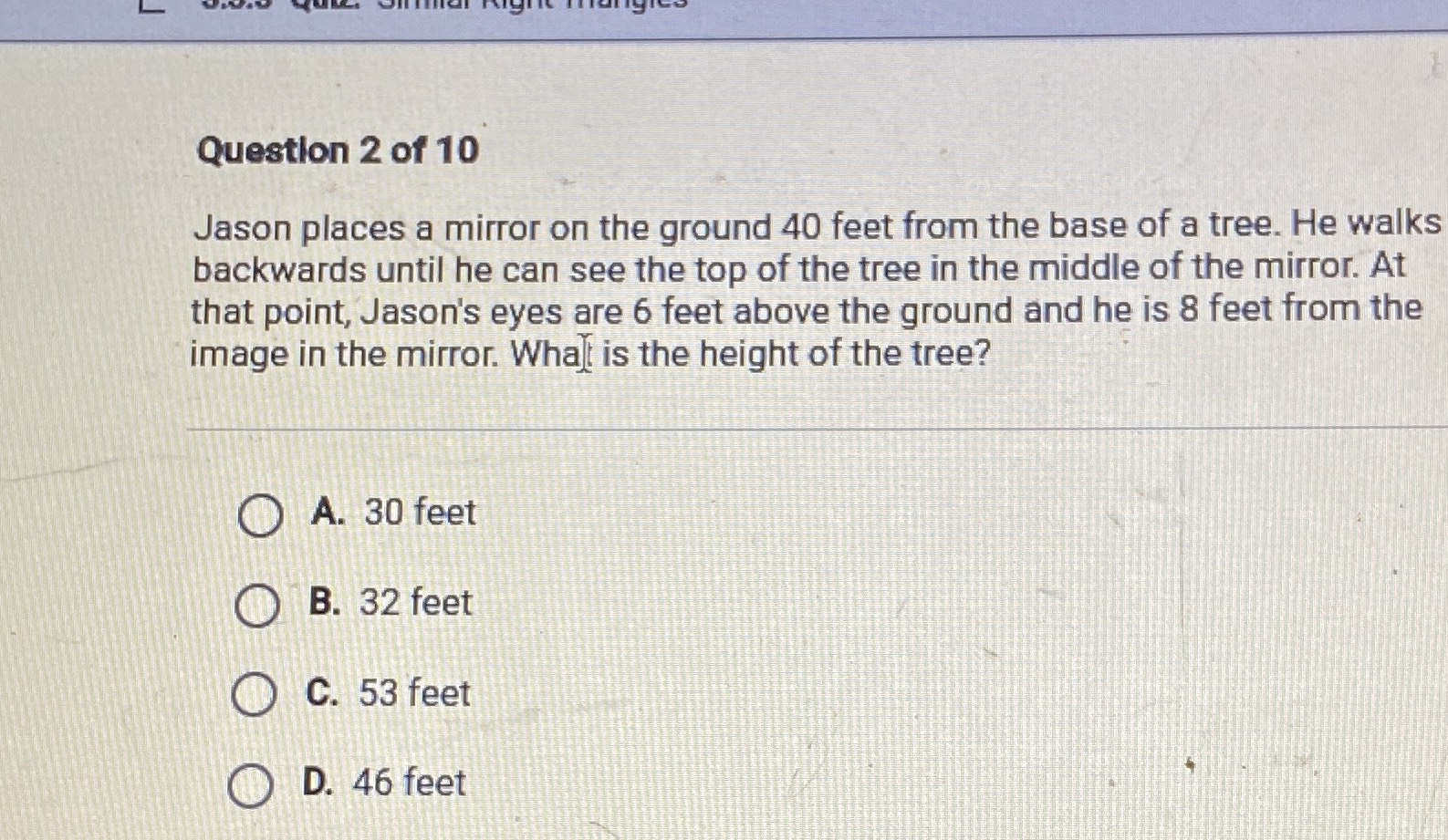Jason places a mirror on the ground $$40$$ feet from the base of a tree. He walks backward until he can see the top of the tree in the middle of the mirror. At that point, Jason's eyes are $$6$$ feet above the ground and he is $$8$$ feet from the image in the mirror. Wha] is the height of the tree? A. $$30$$ feet B. $$32$$ feet C. $$53$$ feet D. $$46$$ feet

A. $$30$$ feet

B. $$32$$ feet

C. $$53$$ feet

D. $$46$$ feet

Q: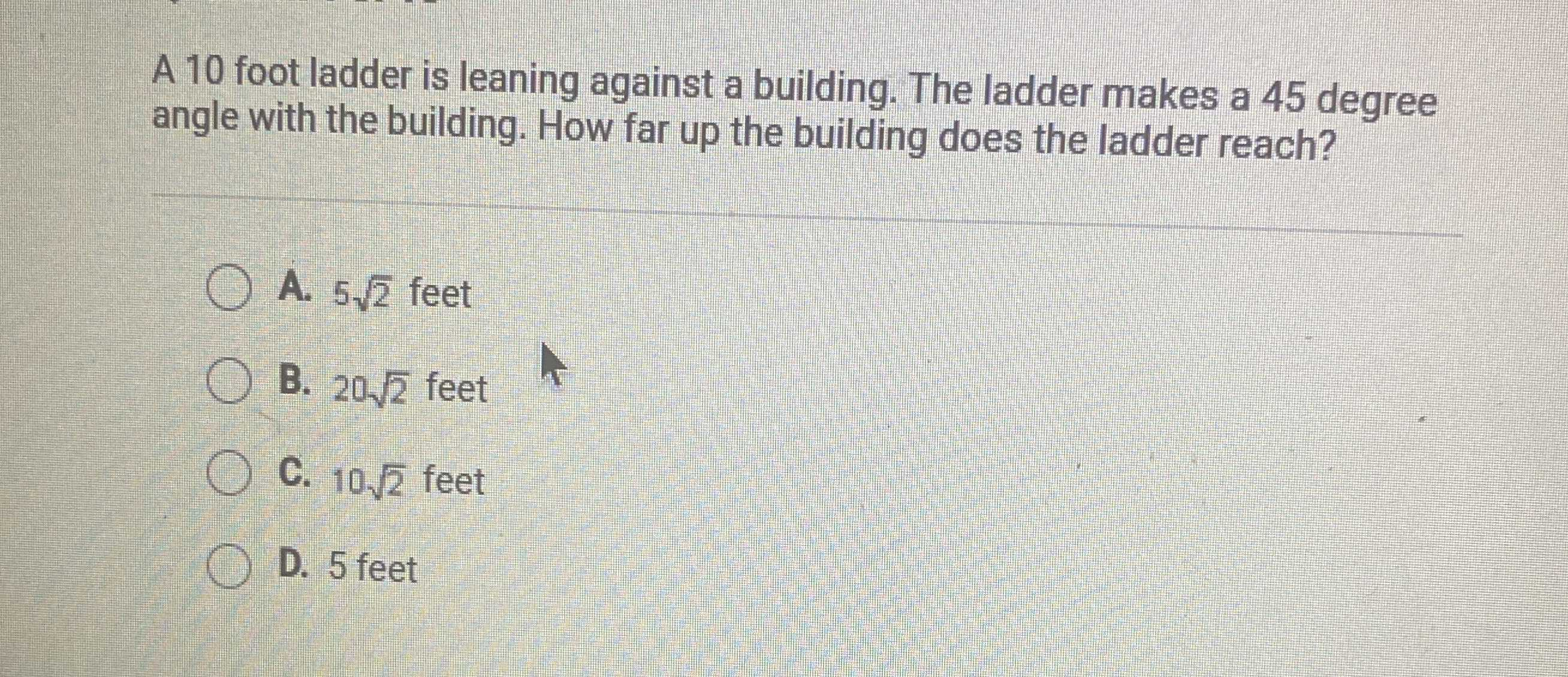$$10$$ foot ladder is leaning against a building. The ladder makes a $$45$$ degree angle with the building. How far up the building does the ladder reach?

A. $$5 \sqrt { 2 }$$ feet

B. $$20 \sqrt { 2 }$$ feet

C. $$10 \sqrt { 2 }$$ feet

D. $$5$$ feet

Q: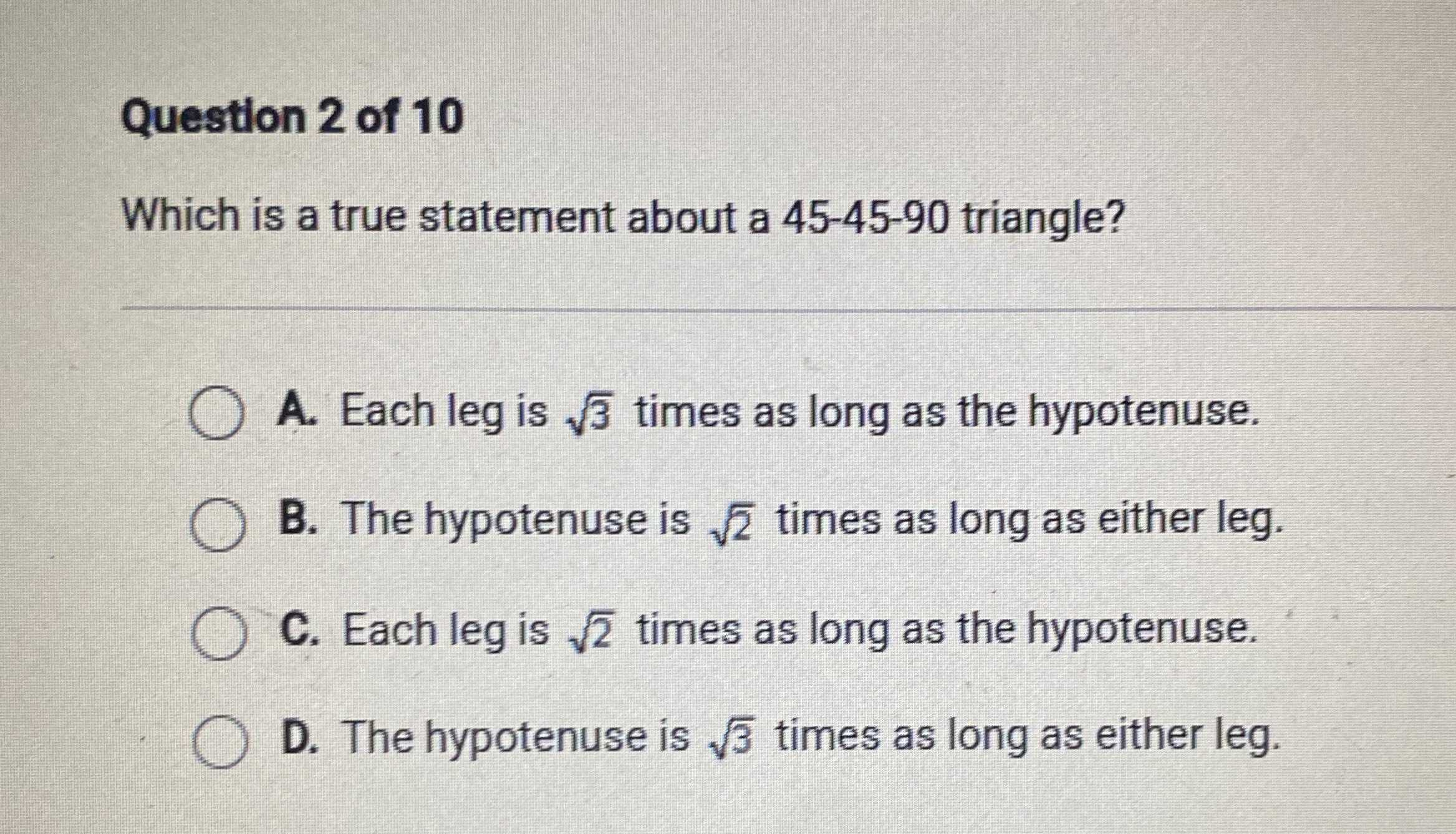Which is a true statement about a $$45 - 45 - 90$$ triangle?

A. Each leg is $$\sqrt { 3 }$$ times as long as the hypotenuse.

B. The hypotenuse is $$\sqrt { 2 }$$ times as long as either leg.

C. Each leg is $$\sqrt { 2 }$$ times as long as the hypotenuse.

D. The hypotenuse is $$\sqrt { 3 }$$ times as long as either leg.

Q: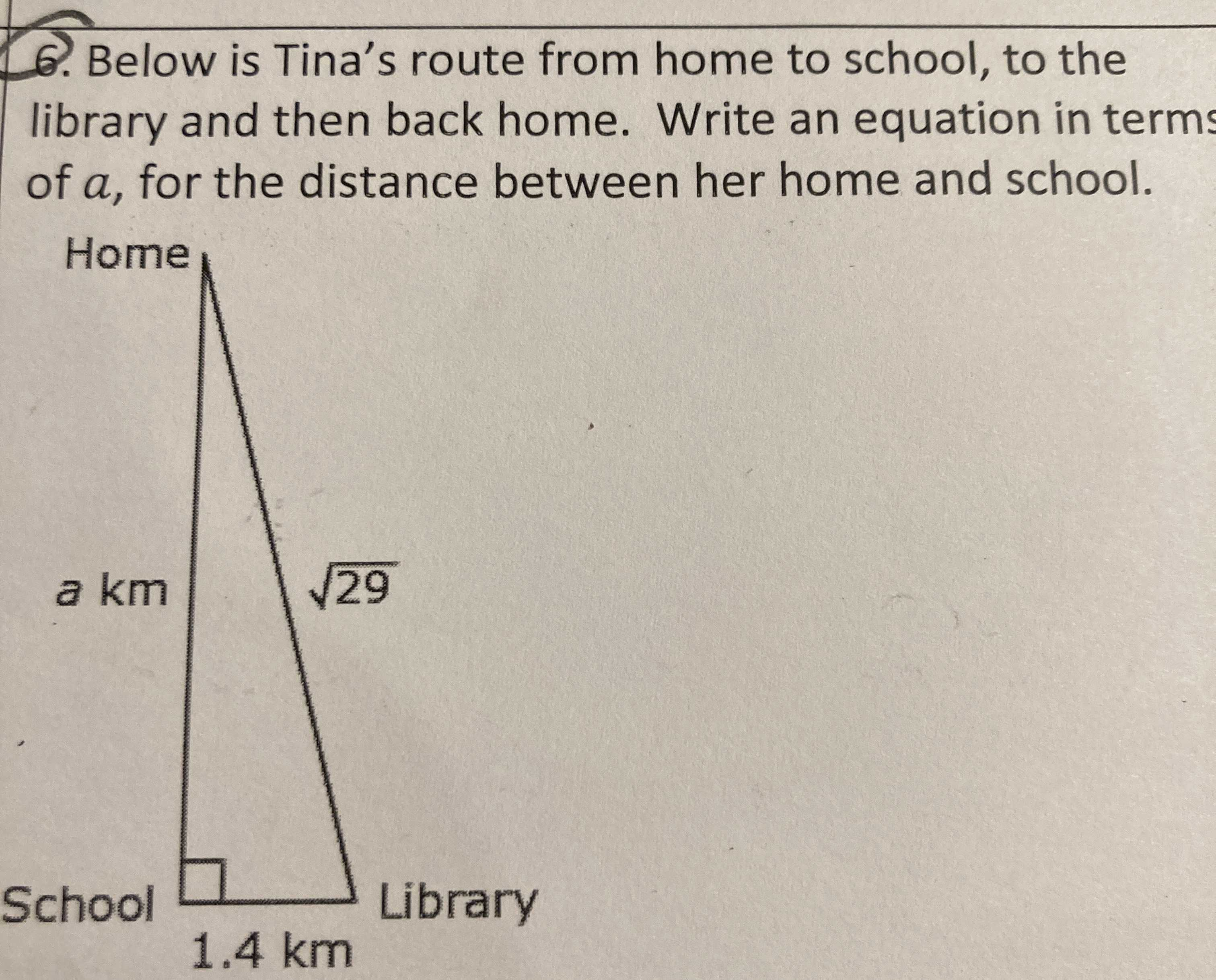Below is Tina's route from home to school, to the library and then back home. Write an equation in terms of $$a$$ , for the distance between her home and school.

Q: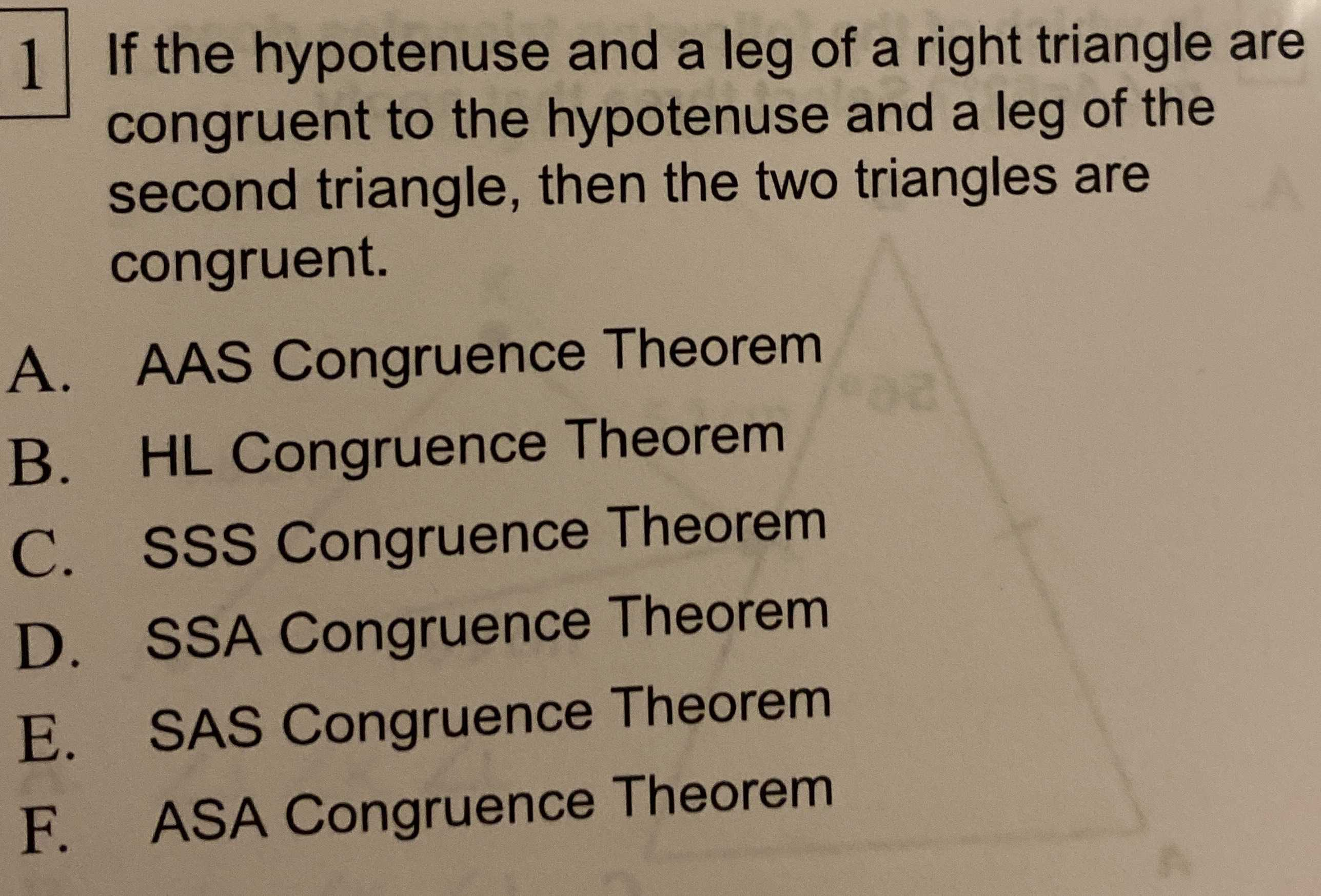If the hypotenuse and a leg of a right triangle are congruent to the hypotenuse and a leg of the second triangle, then the two triangles are congruent.

A. AAS Congruence Theorem

B. HL Congruence Theorem

C. SSS Congruence Theorem

D. SSA Congruence Theorem

E. SAS Congruence Theorem

F. ASA Congruence Theorem

Q: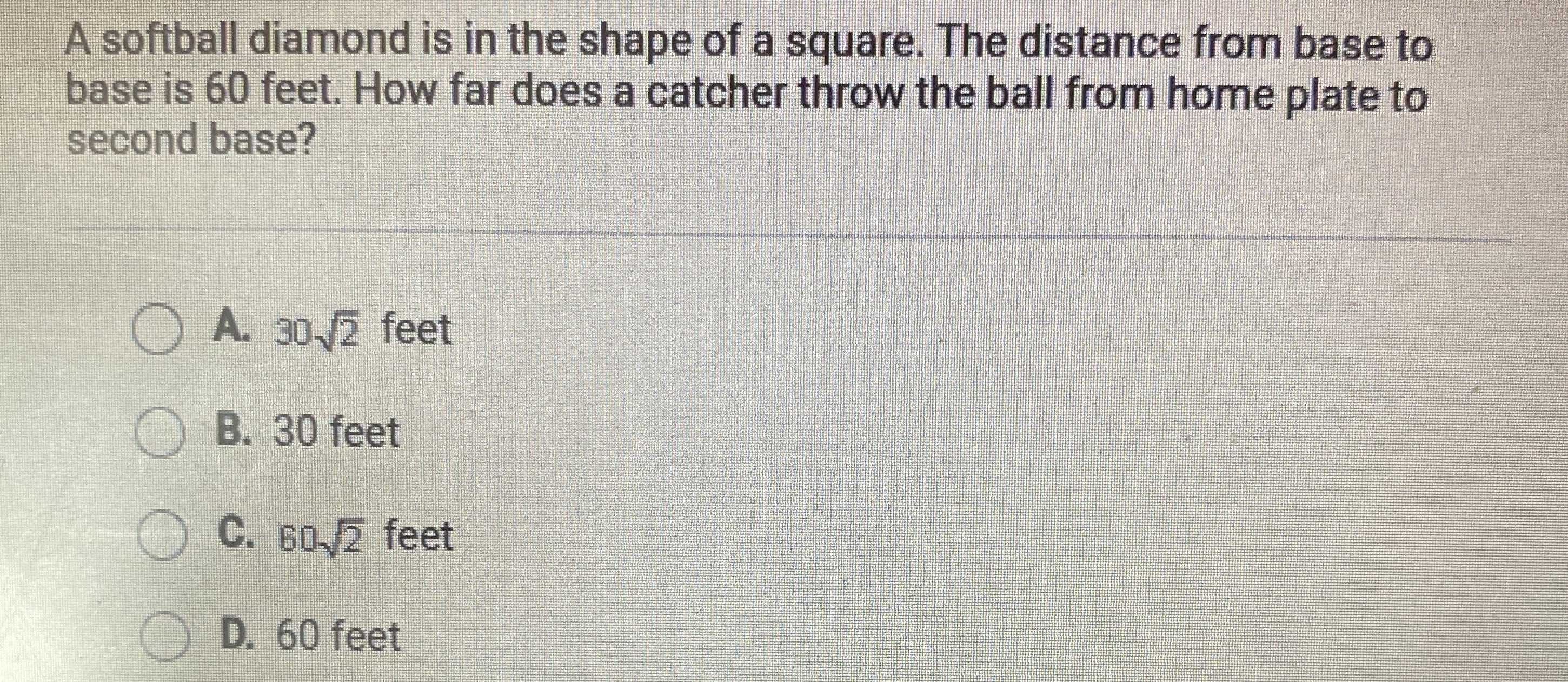A softball diamond is in the shape of a square. The distance from base to base is $$60$$ feet. How far does a catcher throw the ball from home plate to second base?

A. $$30 \sqrt { 2 }$$ feet

B. $$30$$ feet

C. $$60 \sqrt { 2 }$$ feet

D. $$60$$ feet

Q: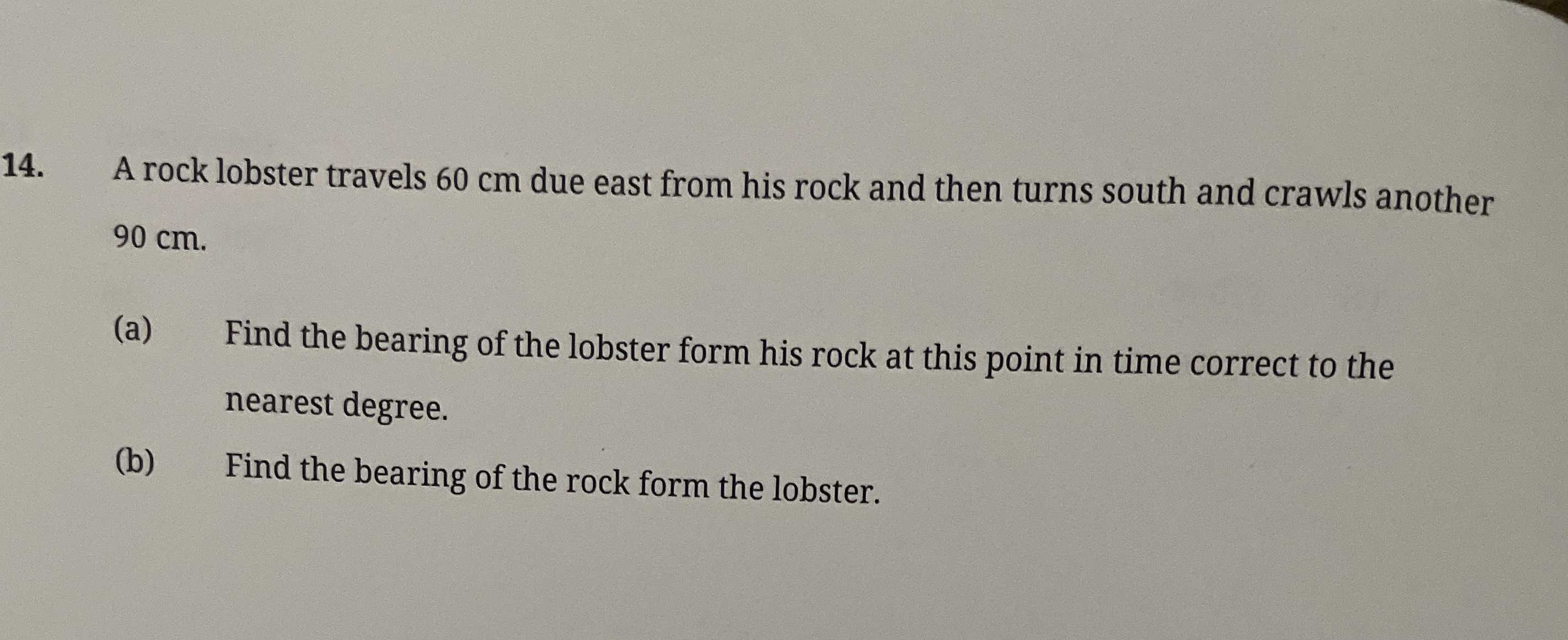A rock lobster travels $$60 cm$$ due east from his rock and then turns south and crawls another

$$90 cm$$ .

(a) Find the bearing of the lobster from his rock at this point in time correct to the nearest degree.

(b) Find the bearing of the rock from the lobster.

Q: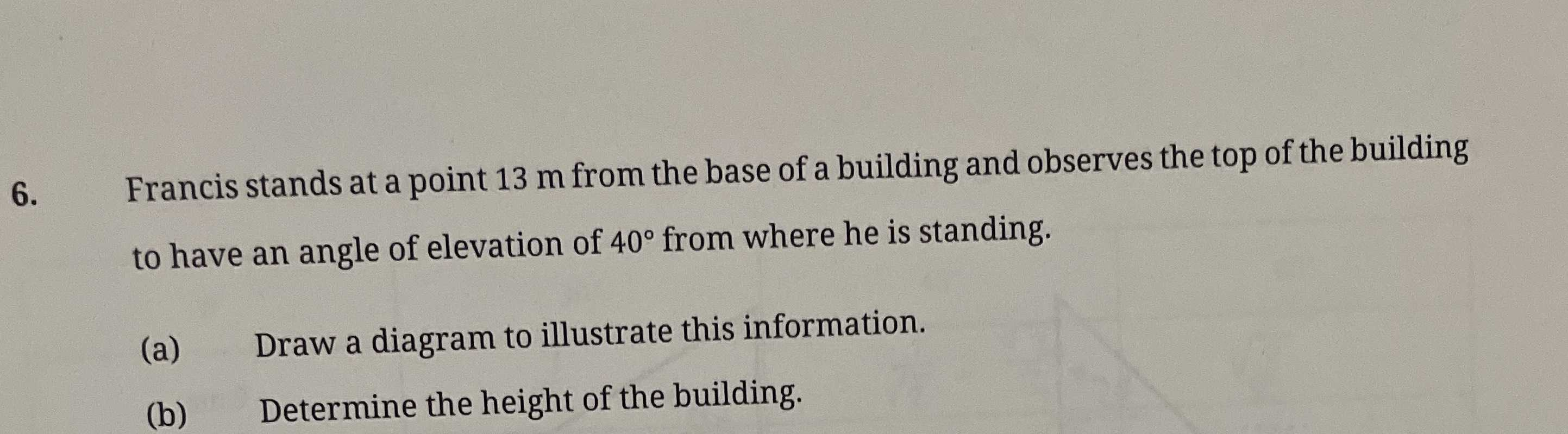6. Francis stands at a point $$13 m$$ from the base of a building and observes the top of the building to have an angle of elevation of $$40 ^ { \circ }$$ from where he is standing.

(a) Draw a diagram to illustrate this information. (b) Determine the height of the building.

Q: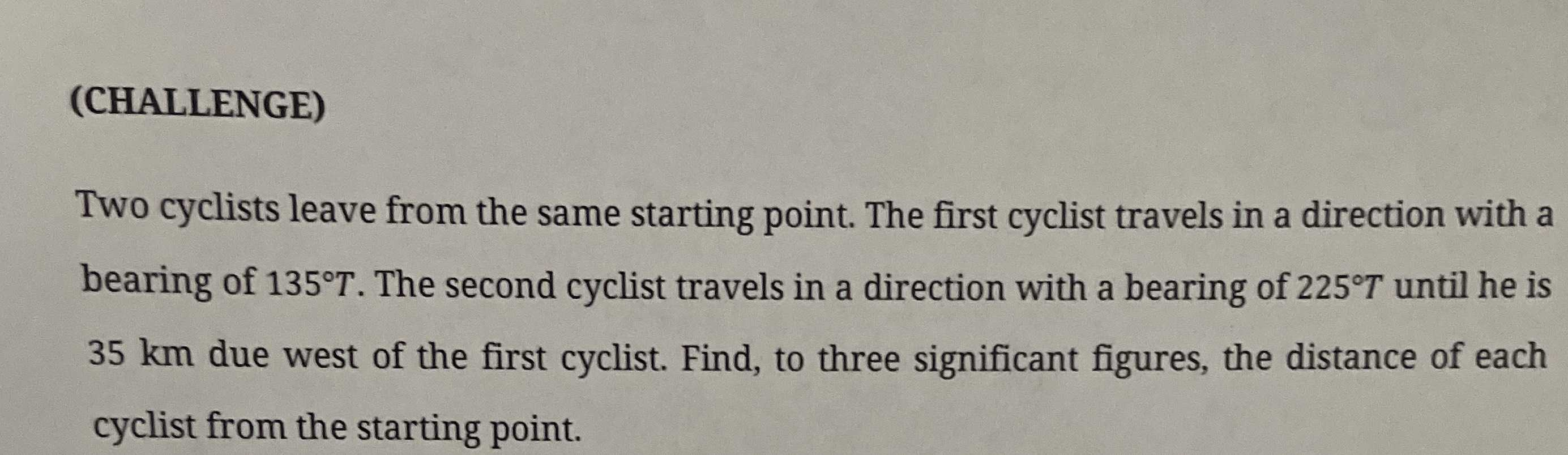Two cyclists leave from the same starting point. The first cyclist travels in a direction with a bearing of $$135 ^ { \circ } T$$ . The second cyclist travels in a direction with a bearing of $$225 ^ { \circ } T$$ until he is $$35 km$$ due west of the first cyclist. Find, to three significant figures, the distance of each cyclist from the starting point.

Q: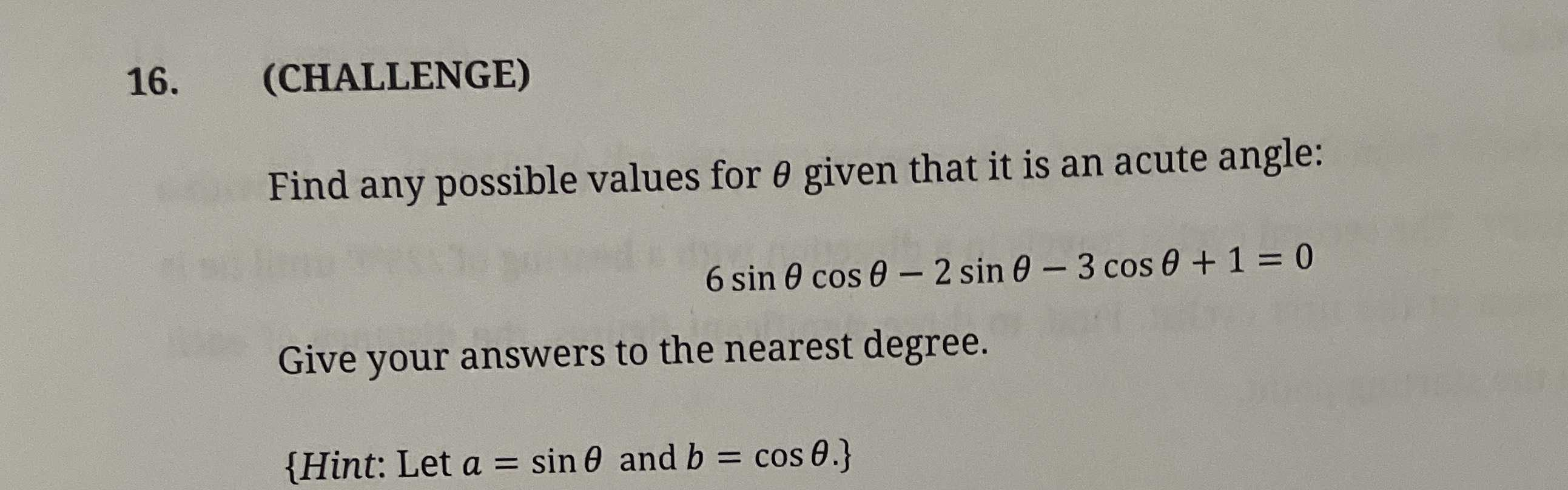Find any possible values for $$\theta$$ given that it is an acute angle:

$$6 \sin \theta \cos \theta - 2 \sin \theta - 3 \cos \theta + 1 = 0$$

$$\{$$ Hint: Let $$a = \sin \theta$$ and $$b = \cos \theta . \}$$

Q: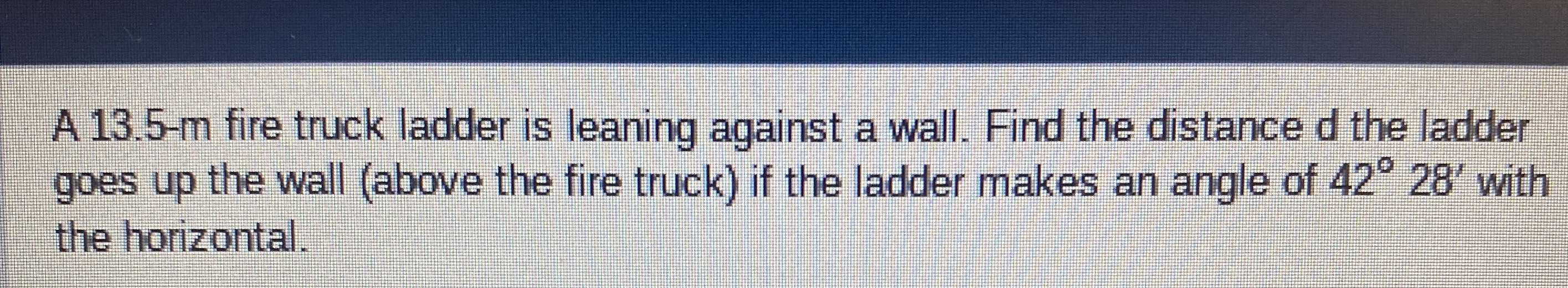A 13.5-m fire truck ladder is leaning against a wall. Find the distance $$d$$ the ladder goes up the wall (above the fire truck) if the ladder makes an angle of $$42 ^ { \circ } 28 ^ { \prime }$$ with the horizontal.

Q: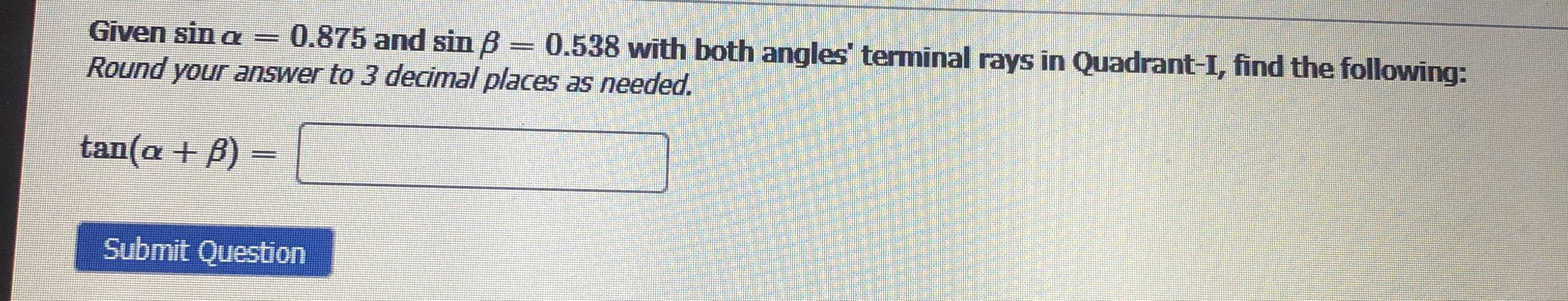Given $$\sin \alpha = 0.875$$ and $$\sin \beta = 0.538$$ with both angles' terminal rays in Quadrant-I, find the following:

$$\tan ( \alpha + \beta ) =$$ _______

Round your answer to $$3$$ decimal places as needed.

Q: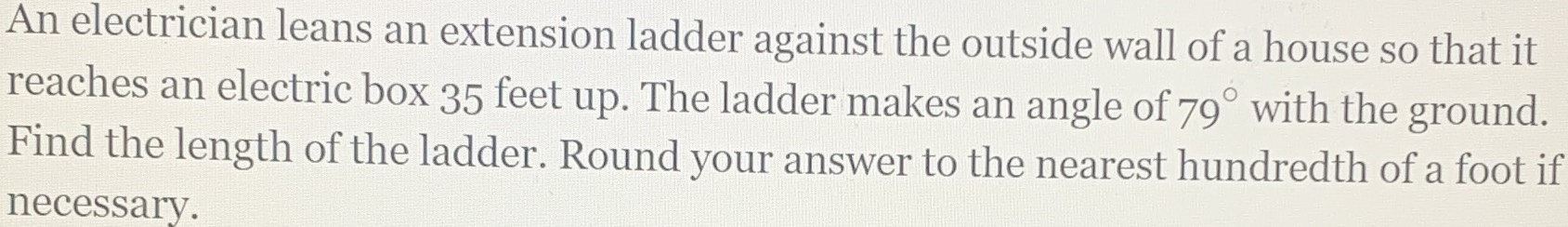An electrician leans an extension ladder against the outside wall of a house so that it reaches an electric box $$35$$ feet up. The ladder makes an angle of $$79 ^ { \circ }$$ with the ground. Find the length of the ladder. Round your answer to the nearest hundredth of a foot if necessary.

Q: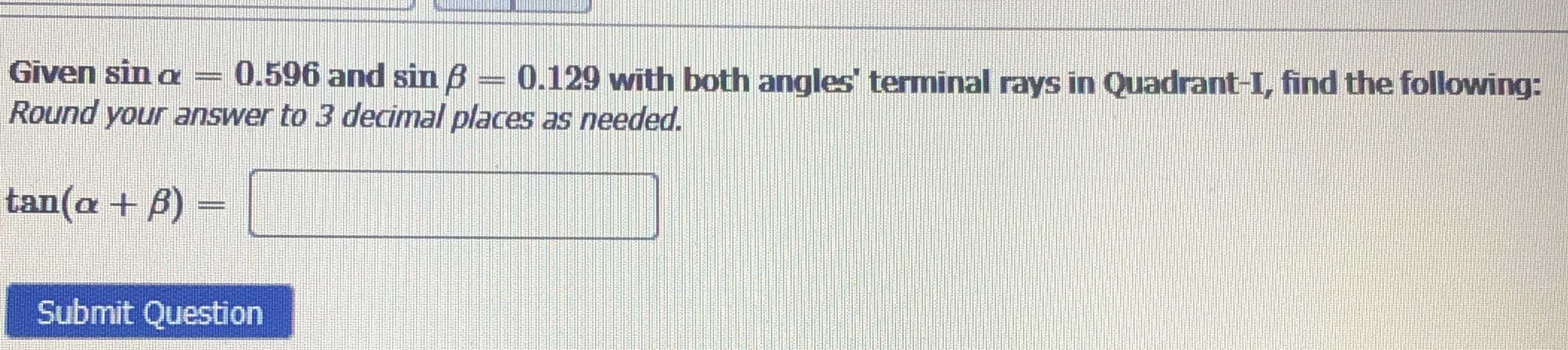Given sin $$\alpha = 0.596$$ and $$\sin \beta = 0.129$$ with both angles' terminal rays in Quadrant-I, find the following:

$$\tan ( \alpha + \beta ) =$$ _______

Round your answer to $$3$$ decimal places as needed.

Q: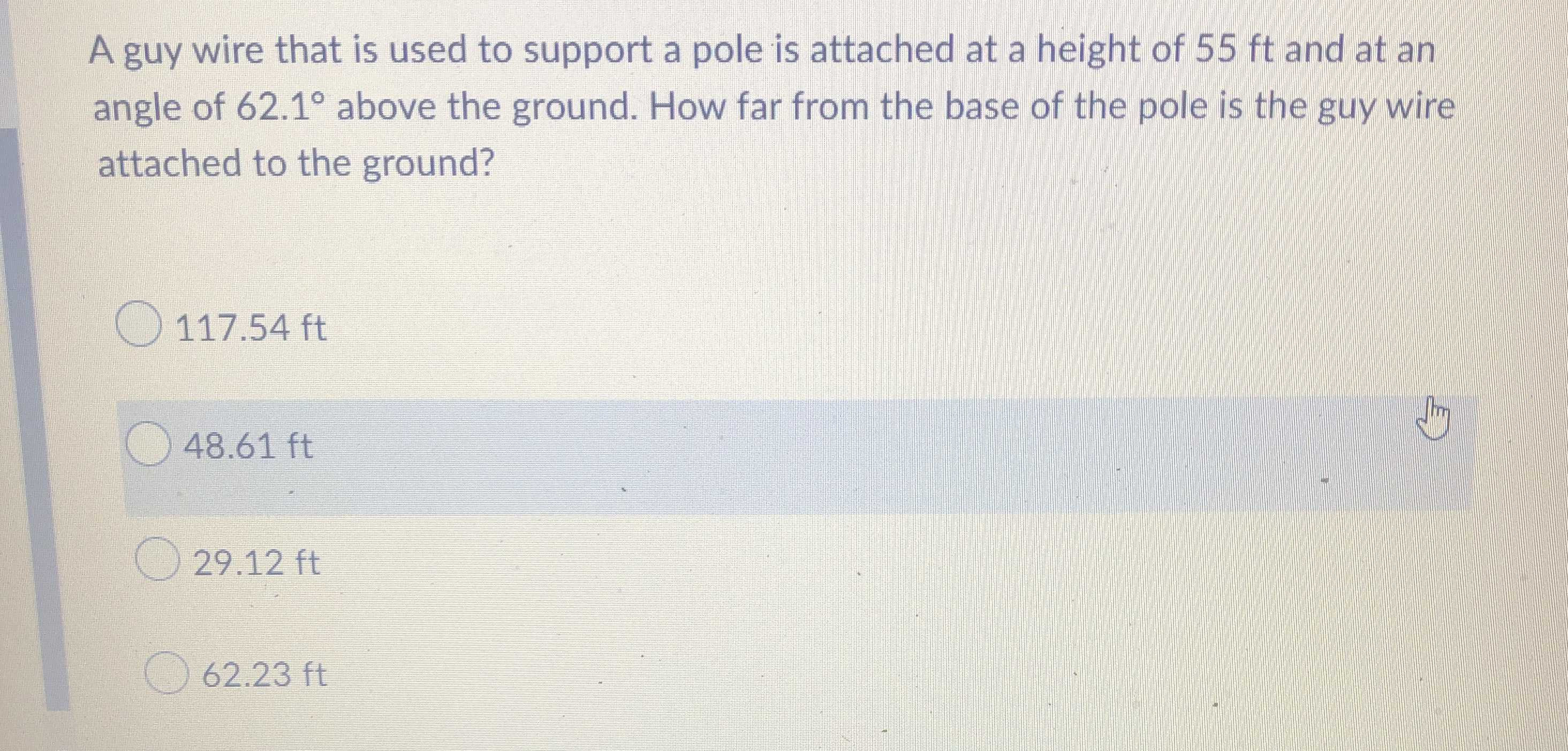A guy wire that is used to support a pole is attached at a height of $$55 ft$$ and at an angle of $$62.1 ^ { \circ }$$ above the ground. How far from the base of the pole is the guy wire attached to the ground?

$$117.54 ft$$

$$48.61 ft$$

$$29.12 ft$$

$$62.23 ft$$

Q: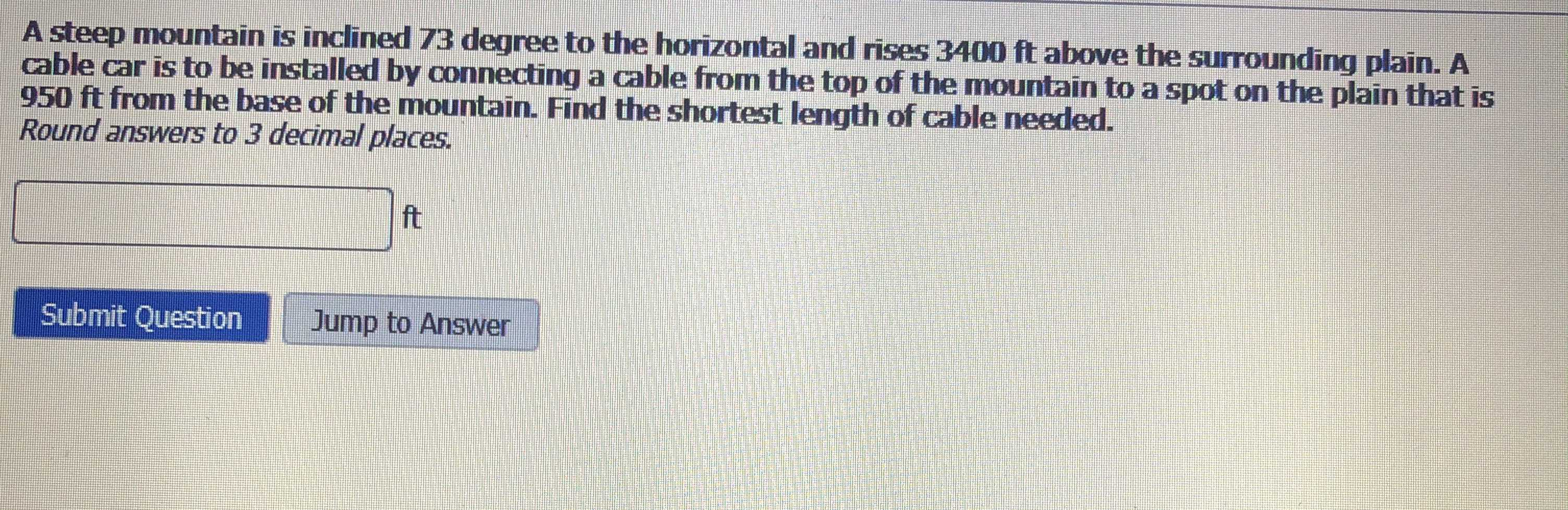A steep mountain is inclined $$73$$ degree to the horizontal and rises $$3400$$ ft above the surrounding plain. A cable car is to be installed by connecting a cable from the top of the mountain to a spot on the plain that is $$950$$ ft from the base of the mountain. Find the shortest length of cable needed. Round answers to $$3$$ decimal places.

Q: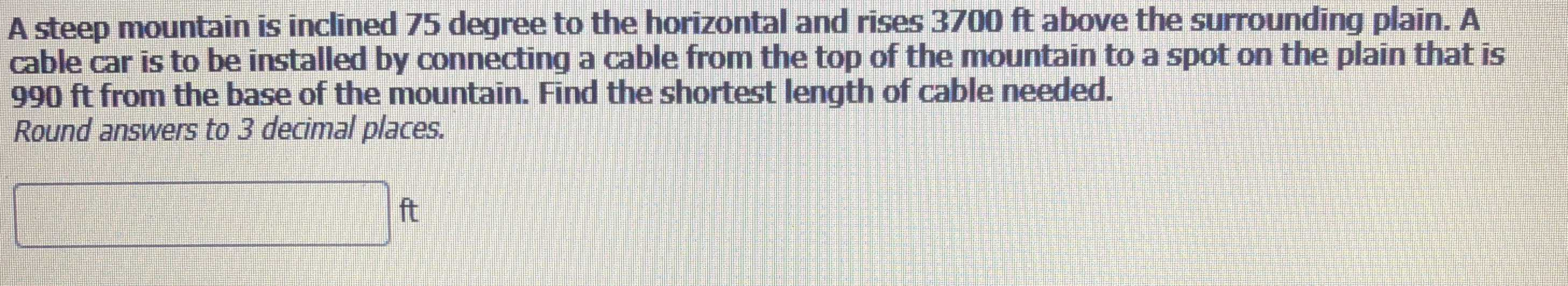A steep mountain is inclined $$75$$ degree to the horizontal and rises $$3700$$ ft above the surrounding plain. A cable car is to be installed by connecting a cable from the top of the mountain to a spot on the plain that is

$$990$$ ft from the base of the mountain. Find the shortest length of cable needed. Round answers to $$3$$ decimal places.

ft

Q: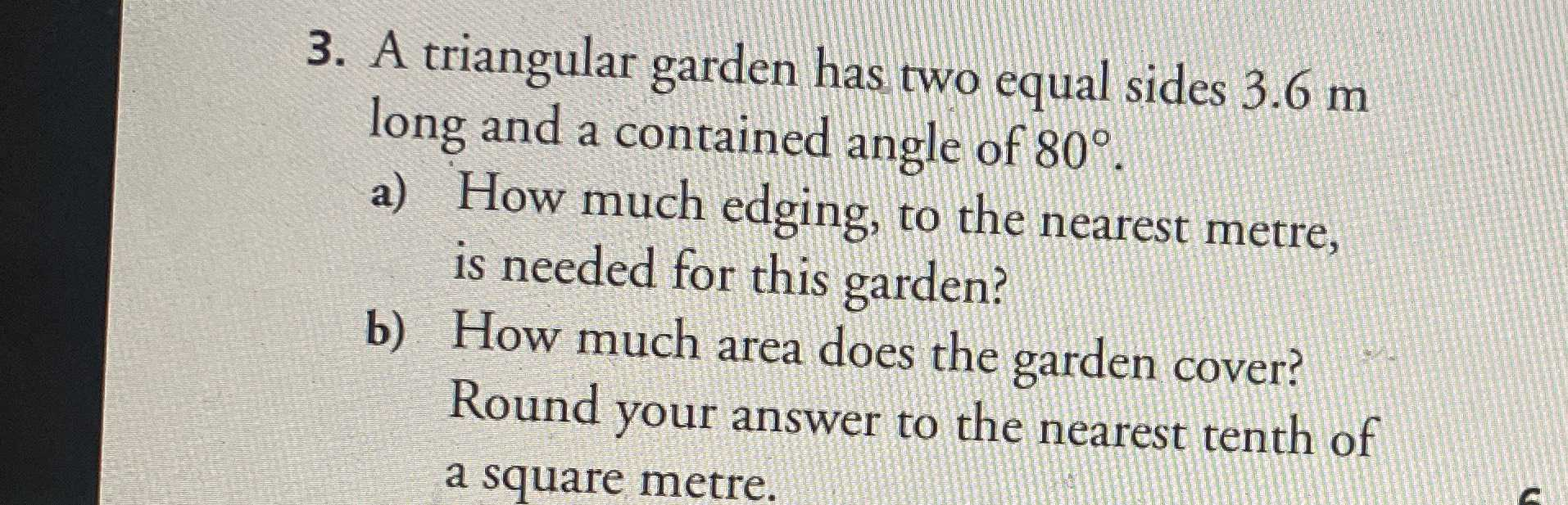A triangular garden has two equal sides $$3.6 m$$ long and a contained angle of $$80 ^ { \circ }$$

a) How much edging, to the nearest meter, is needed for this garden?

b) How much area does the garden cover? Round your answer to the nearest tenth of a square meter.

Q: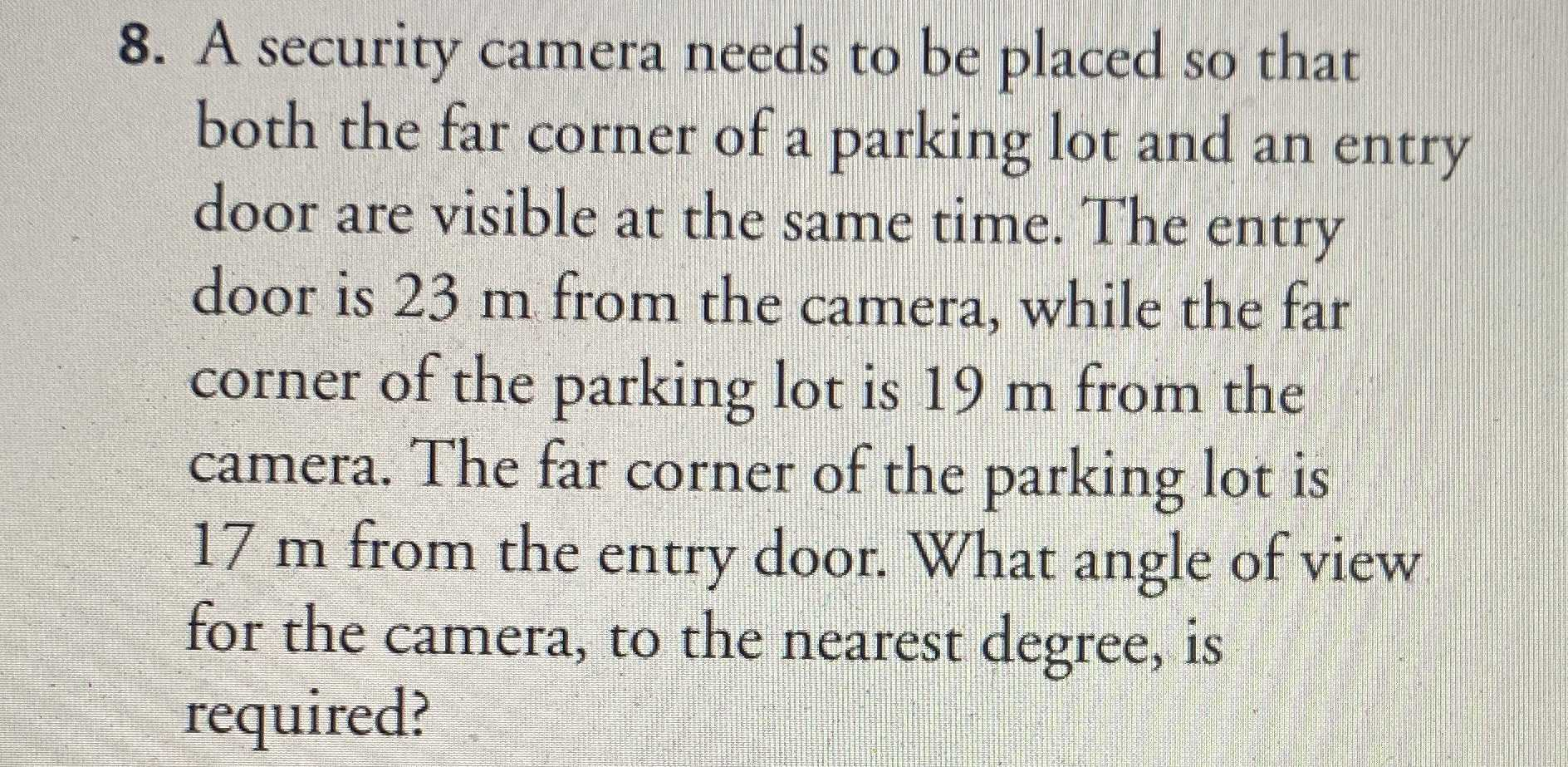A security camera needs to be placed so that both the far corner of a parking lot and an entry door are visible at the same time. The entry door is $$23 m$$ from the camera, while the far corner of the parking lot is $$19 m$$ from the camera. The far corner of the parking lot is $$17 m$$ from the entry door. What angle of view for the camera, to the nearest degree, is required?

Q: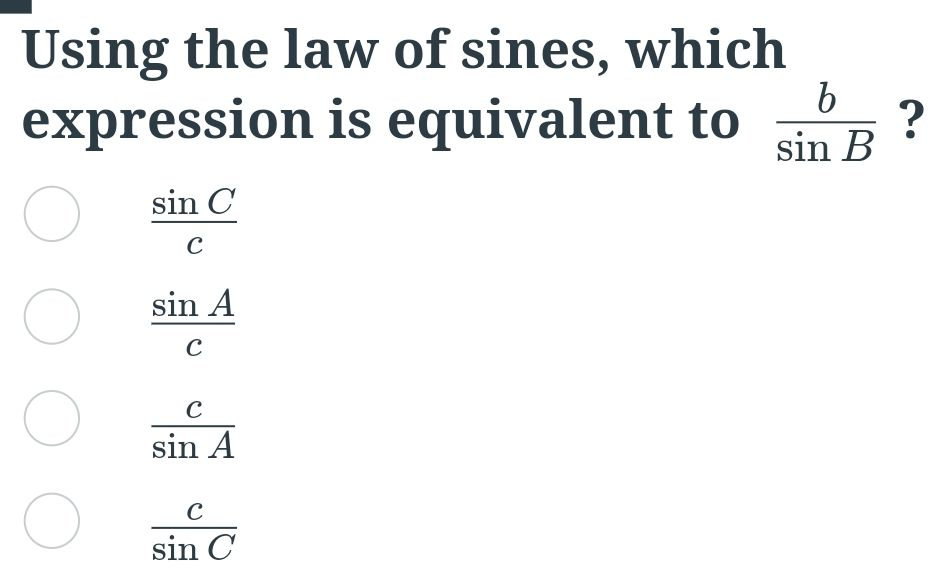Using the law of sines, which expression is equivalent to $$\frac { b } { \sin B }$$ ?

A. $$\frac { \sin C } { c }$$

B. $$\frac { \sin A } { c }$$

C. $$\frac { c } { \sin A }$$

D. $$\frac { c } { \sin C }$$

Q: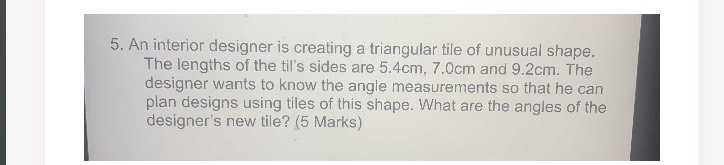An interior designer is creating a triangular tile of unusual shape. The lengths of the til's sides are $$5.4 cm , 7.0 cm$$ and $$9.2 cm$$ . The designer wants to know the angle measurements so that he can plan designs using tiles of this shape. What are the angles of the designer's new tile?

Q: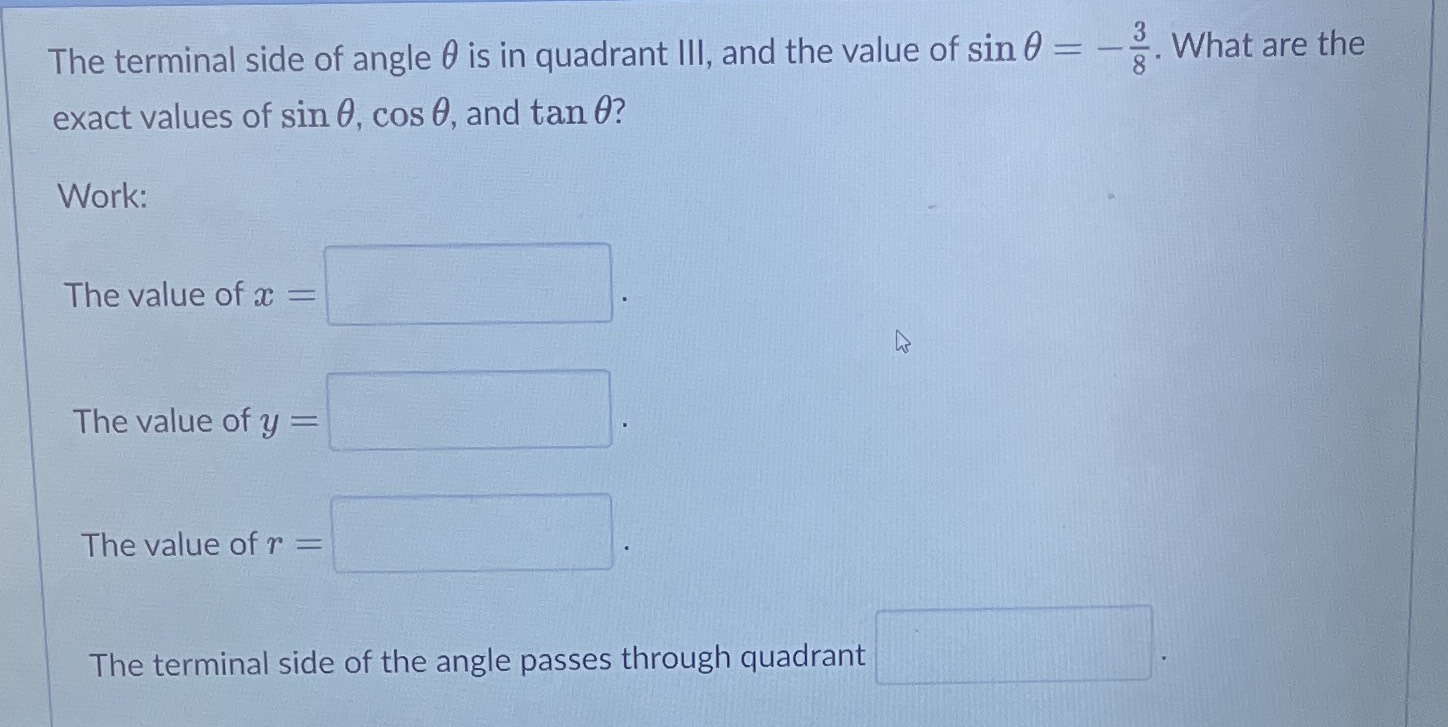The terminal side of angle $$\theta$$ is in quadrant III, and the value of $$\sin \theta = - \frac { 3 } { 8 }$$ . What are the exact values of $$\sin \theta , \cos \theta ,$$ and $$\tan \theta$$ ? Work:

The value of $$x =$$

The value of $$y =$$

The value of $$r =$$

The terminal side of the angle passes through quadrant

Q: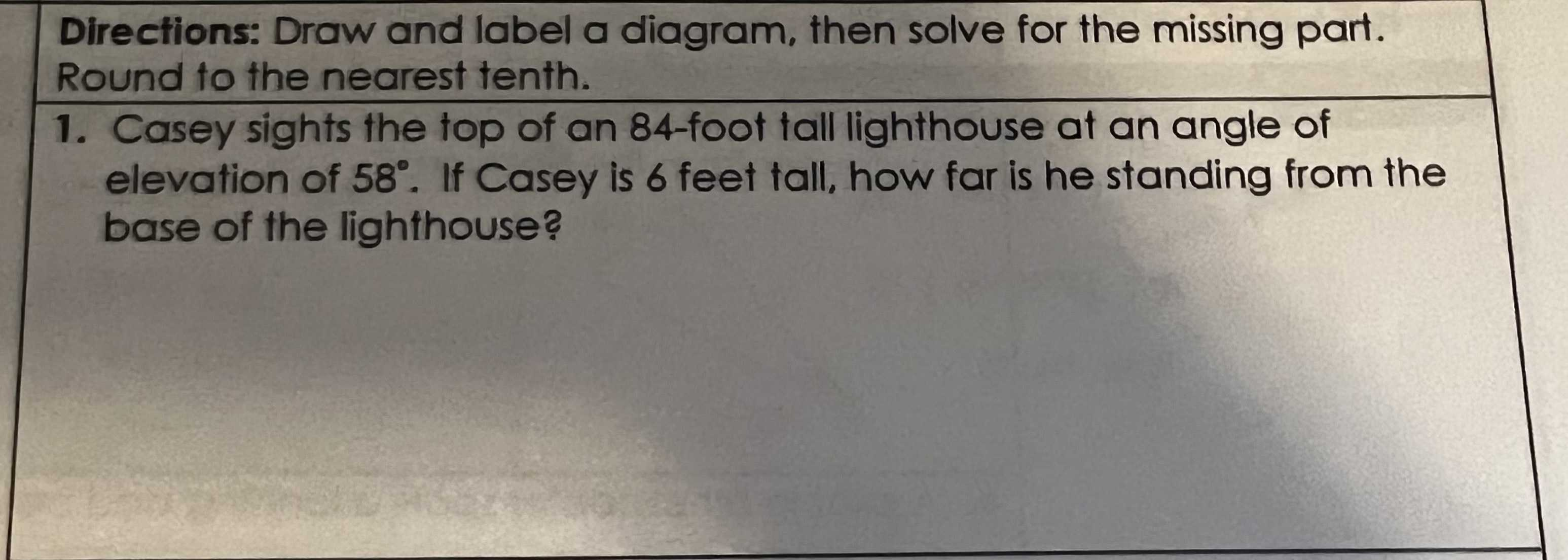Casey sights the top of an $$84$$ -foot tall lighthouse at an angle of elevation of $$58 ^ { \circ }$$ . If Casey is $$6$$ feet tall, how far is he standing from the base of the lighthouse?